Workflow to assess the relationship between environmental parameters and microbial communities.

``````knitr::opts_chunk\$set(eval = FALSE, echo = TRUE)
set.seed(119)
#library(conflicted)
#pacman::p_depends(vegan, local = TRUE)
#pacman::p_depends_reverse(vegan, local = TRUE)
library(phyloseq); packageVersion("phyloseq")
ggpubr, ggvegan, ggfortify, vegan, reactable,
install = FALSE, update = FALSE)

options(scipen=999)
knitr::opts_current\$get(c(
"cache",
"cache.path",
"cache.rebuild",
"dependson",
"autodep"
))``````

# Synopsis

In this workflow we compare the environmental metadata with the microbial community data. Part 1 involves the 16S rRNA community data and Part 2 deals with the ITS community data. Each workflow contains the same major steps:

1. Metadata Normality Tests: Shapiro-Wilk Normality Test to test whether each matadata parameter is normally distributed.
2. Normalize Parameters: R package `bestNormalize` to find and execute the best normalizing transformation.
3. Split Metadata parameters into groups: a) Environmental and edaphic properties, b) Microbial functional responses, and c) Temperature adaptation properties.
4. Autocorrelation Tests: Test all possible pair-wise comparisons, on both normalized and non-normalized data sets, for each group.
5. Remove autocorrelated parameters from each group.
6. Dissimilarity Correlation Tests: Use Mantel Tests to see if any on the metadata groups are significantly correlated with the community data.
7. Best Subset of Variables: Determine which of the metadata parameters from each group are the most strongly correlated with the community data. For this we use the `bioenv` function from the `vegan` package.
8. Distance-based Redundancy Analysis: Ordination analysis of samples and metadata vector overlays using `capscale`, also from the `vegan` package.

## Workflow Input

Files needed to run this workflow can be downloaded from figshare. You will also need the metadata table, which can be accessed below.

In this section we provide access to the complete environmental metadata containing 61 parameters across all 15 samples.

(common) Table 1 | Metadata values for each sample.

# Multivariate Summary

Summary results for of `envfit` and `bioenv` tests for edaphic properties, soil functional response, and temperature adaptation for both 16S rRNA and ITS data sets.

## 16S rRNA

Metadata parameters removed based on autocorrelation results.

• edaphic properties: TEB + DON + Na + Al + Ca
• soil functional response: micN + micNP + enzCN + enzCP + BPase + CEase + LPase + Nase + Pase
• temperature adaptation: NUE + PUE + SI

Metadata parameters removed from capscale analysis based on Degrees of Freedom.

• edaphic properties: Mg + Mn
• soil functional response: NONE

(16S rRNA) Table 1 | Significant results of envfit & bioenv tests for the 16S rRNA data set.

## ITS

Metadata parameters removed based on autocorrelation results.

• edaphic properties: TEB + DON + Na + Al + Ca
• soil functional response: micN + micNP + enzCN + enzCP + BPase + CEase + LPase + Nase + Pase
• temperature adaptation: NUE + PUE + PQ10 + SI

Metadata parameters removed from capscale analysis based on Degrees of Freedom.

• edaphic properties: Mg + Mn + Na + Al + Fe + K
• soil functional response: NONE

(ITS) Table 1 | Significant results of envfit & bioenv tests for the ITS data set.

# 16s rRNA

## Data Formating

1. Transform `ps` Objects

The first step is to transform the phyloseq objects.

Code
``````samp_ps <- c("ssu18_ps_work", "ssu18_ps_pime", "ssu18_ps_perfect",
"ssu18_ps_work_otu", "ssu18_ps_pime_otu", "ssu18_ps_perfect_otu")
for (i in samp_ps) {
tmp_get <- get(i)
tmp_ps <- transform_sample_counts(tmp_get, function(otu) 1e5 * otu/sum(otu))
tmp_ps@phy_tree <- NULL
tmp_ps <- prune_samples(sample_sums(tmp_ps) > 0, tmp_ps)
tmp_tree <- rtree(ntaxa(tmp_ps), rooted = TRUE, tip.label = taxa_names(tmp_ps))
tmp_samp <- data.frame(sample_data(tmp_get))
tmp_samp <- tmp_samp %>% dplyr::rename("TREAT_T" = "TEMP")
tmp_ps <- merge_phyloseq(tmp_ps, sample_data, tmp_tree)
sample_data(tmp_ps) <- tmp_samp
tmp_name <- purrr::map_chr(i, ~ paste0(., "_prop"))
print(tmp_name)
assign(tmp_name, tmp_ps)
rm(list = ls(pattern = "tmp_"))
}``````
1. Format for `mctoolsr`

Then a bit of formatting to make the data compatible with `mctoolsr`.

Code
``````for (i in samp_ps) {
tmp_get <- get(purrr::map_chr(i, ~ paste0(., "_prop")))
tmp_tax <- data.frame(tax_table(tmp_get))
tmp_tax\$ASV_SEQ <- NULL

tmp_col_names <- colnames(tmp_tax)
tmp_tax_merge <- tmp_tax %>% tidyr::unite(taxonomy,
all_of(tmp_col_names),
sep = ";")
tmp_tax_merge <- tmp_tax_merge %>% tibble::rownames_to_column("#OTU_ID")
tmp_otu <- data.frame(t(otu_table(tmp_get)))
tmp_otu <- tmp_otu %>% tibble::rownames_to_column("#OTU_ID")
tmp_otu_tax <- dplyr::left_join(tmp_otu, tmp_tax_merge, by = "#OTU_ID")

tmp_samp <- data.frame(sample_data(tmp_get))
tmp_samp[,c(1,3)] <- NULL
tmp_samp <- tmp_samp %>% tibble::rownames_to_column("#SampleID")

tmp_md <- dplyr::left_join(tmp_samp, tmp_metad, by = "#SampleID")

tmp_otu_name <- purrr::map_chr(i, ~ paste0(., "_otu_tax"))
print(tmp_otu_name)
assign(tmp_otu_name, tmp_otu_tax)

tmp_md_name <- purrr::map_chr(i, ~ paste0(., "_md"))
print(tmp_md_name)
assign(tmp_md_name, tmp_md)

write_delim(tmp_otu_tax, paste(tmp_path, tmp_otu_name, ".txt", sep = ""), delim = "\t")
write_delim(tmp_md, paste(tmp_path, tmp_md_name, ".txt", sep = ""), delim = "\t")
rm(list = ls(pattern = "tmp_"))
}``````
Code
``````for (i in samp_ps) {
tmp_otu_name <- purrr::map_chr(i, ~ paste0(., "_otu_tax"))
tmp_md_name <- purrr::map_chr(i, ~ paste0(., "_md"))
tmp_tax_table_fp <- paste(tmp_path, tmp_otu_name, ".txt", sep = "")
tmp_map_fp <- paste(tmp_path, tmp_md_name, ".txt", sep = "")
tmp_input_name <- purrr::map_chr(i, ~ paste0(., "_mc"))
print(tmp_input_name)
assign(tmp_input_name, tmp_input)
rm(list = ls(pattern = "tmp_"))
}
rm(list = ls(pattern = "_md"))
rm(list = ls(pattern = "_otu_tax"))``````

## Choose Data Set

At this point in the code we need to choose a data set to use, formatted with `mctoolsr`. Remember, there are four choices:

1. FULL, unfiltered data set.
2. Arbitrary filtered data set.
3. PERfect filtered data set.
4. PIME filtered data set.

This way, if we want to test other data sets we only need to change the name here.

``````objects(pattern = "_mc")
ssu18_select_mc <- ssu18_ps_pime_mc``````

## Normality Tests

Before proceeding, we need to test each parameter in the metadata to see which ones are and are not normally distributed. For that, we use the Shapiro-Wilk Normality Test. Here we only need one of the metadata files.

Code
``````temp_md <- ssu18_select_mc\$map_loaded
temp_md[,1:9] <- NULL
shap_results <- NULL

for (i in colnames(temp_md)) {
tmp_shap <- shapiro.test(temp_md[[i]])
tmp_p <- round(tmp_shap\$p.value, digits = 5)
tmp_res <- eval(isTRUE(tmp_shap\$p.value < 0.05))
shap_results <- rbind(shap_results, data.frame(i, tmp_p, tmp_res))
rm(list = ls(pattern = "tmp_"))
}
colnames(shap_results) <- c("parameter", "p-value", "tranform")
shap_results
dplyr::filter(shap_results, tranform == "TRUE")
md_to_tranform <- shap_results\$parameter[shap_results\$tranform == TRUE]
rm(list = ls(pattern = "temp_md"))``````
Show the results of each normality test for metadata parameters

(16S rRNA) Table 2 | Results of the Shapiro-Wilk Normality Tests. P-values in red are significance (p-value < 0.05) meaning the parameter needs to be normalized.

Looks like we need to transform 25 metadata parameters.

## Normalize Parameters

Here we use the R package `bestNormalize` to find and execute the best normalizing transformation. The function will test the following normalizing transformations:

• `arcsinh_x` performs an arcsinh transformation.

• `boxcox` Perform a Box-Cox transformation and center/scale a vector to attempt normalization. `boxcox` estimates the optimal value of lambda for the Box-Cox transformation. The function will return an error if a user attempt to transform nonpositive data.

• `yeojohnson` Perform a Yeo-Johnson Transformation and center/scale a vector to attempt normalization. `yeojohnson` estimates the optimal value of lambda for the Yeo-Johnson transformation. The Yeo-Johnson is similar to the Box-Cox method, however it allows for the transformation of nonpositive data as well.

• `orderNorm` The Ordered Quantile (ORQ) normalization transformation, `orderNorm()`, is a rank-based procedure by which the values of a vector are mapped to their percentile, which is then mapped to the same percentile of the normal distribution. Without the presence of ties, this essentially guarantees that the transformation leads to a uniform distribution.

• `log_x` performs a simple log transformation. The parameter a is essentially estimated by the training set by default (estimated as the minimum possible to some extent epsilon), while the base must be specified beforehand. The default base of the log is 10.

• `sqrt_x` performs a simple square-root transformation. The parameter a is essentially estimated by the training set by default (estimated as the minimum possible), while the base must be specified beforehand.

• `exp_x` performs a simple exponential transformation.

See this GitHub issue (#5) for a description on getting reproducible results. Apparently, you can get different results because the `bestNormalize()` function uses repeated cross-validation (and doesn’t automatically set the seed), so the results will be slightly different each time the function is executed.

Code
``````set.seed(119)
for (i in md_to_tranform) {
tmp_best_norm <- bestNormalize(tmp_md[[i]], r = 1, k = 5, loo = TRUE)
tmp_name <- purrr::map_chr(i, ~ paste0(., "_best_norm_test"))
assign(tmp_name, tmp_best_norm)
print(tmp_name)
rm(list = ls(pattern = "tmp_"))
}``````
Show the chosen transformations
``````
## bestNormalize Chosen transformation of AST ##
orderNorm Transformation with 15 nonmissing obs and no ties
- Original quantiles:
0%    25%    50%    75%   100%
25.800 26.275 28.800 30.280 41.770
_____________________________________

## bestNormalize Chosen transformation of H2O ##
orderNorm Transformation with 15 nonmissing obs and no ties
- Original quantiles:
0%   25%   50%   75%  100%
0.204 0.308 0.370 0.384 0.401
_____________________________________

## bestNormalize Chosen transformation of Al ##
Standardized asinh(x) Transformation with 15 nonmissing obs.:
Relevant statistics:
- mean (before standardization) = 0.0059997
- sd (before standardization) = 0.008280287
_____________________________________

## bestNormalize Chosen transformation of Ca ##
orderNorm Transformation with 15 nonmissing obs and ties
- 10 unique values
- Original quantiles:
0%   25%   50%   75%  100%
25.49 27.19 29.23 34.36 39.79
_____________________________________

## bestNormalize Chosen transformation of Fe ##
Standardized asinh(x) Transformation with 15 nonmissing obs.:
Relevant statistics:
- mean (before standardization) = 0.0119984
- sd (before standardization) = 0.01264569
_____________________________________

## bestNormalize Chosen transformation of TEB ##
orderNorm Transformation with 15 nonmissing obs and ties
- 10 unique values
- Original quantiles:
0%  25%  50%  75% 100%
41.6 43.3 45.1 51.4 61.7
_____________________________________

## bestNormalize Chosen transformation of ECEC ##
orderNorm Transformation with 15 nonmissing obs and ties
- 10 unique values
- Original quantiles:
0%  25%  50%  75% 100%
41.7 43.3 45.3 51.5 61.8
_____________________________________

## bestNormalize Chosen transformation of minNO3 ##
Standardized Box Cox Transformation with 15 nonmissing obs.:
Estimated statistics:
- lambda = -0.3344538
- mean (before standardization) = 1.907818
- sd (before standardization) = 0.2388776
_____________________________________

## bestNormalize Chosen transformation of minTIN ##
orderNorm Transformation with 15 nonmissing obs and ties
- 14 unique values
- Original quantiles:
0%     25%     50%     75%    100%
8.760  14.995  23.080  39.935 110.750
_____________________________________

## bestNormalize Chosen transformation of DOC ##
orderNorm Transformation with 15 nonmissing obs and no ties
- Original quantiles:
0%     25%     50%     75%    100%
30.800  44.070  56.680  64.385 118.740
_____________________________________

## bestNormalize Chosen transformation of DOCN ##
orderNorm Transformation with 15 nonmissing obs and no ties
- Original quantiles:
0%    25%    50%    75%   100%
9.440 10.290 11.150 12.095 19.270
_____________________________________

## bestNormalize Chosen transformation of micCN ##
orderNorm Transformation with 15 nonmissing obs and ties
- 14 unique values
- Original quantiles:
0%   25%   50%   75%  100%
4.420 4.585 4.720 5.415 5.850
_____________________________________

## bestNormalize Chosen transformation of BG_ase ##
orderNorm Transformation with 15 nonmissing obs and no ties
- Original quantiles:
0%    25%    50%    75%   100%
3.010  3.735  4.220  5.795 10.480
_____________________________________

## bestNormalize Chosen transformation of BP_ase ##
Standardized Log_b(x + a) Transformation with 15 nonmissing obs.:
Relevant statistics:
- a = 0
- b = 10
- mean (before standardization) = 0.5217757
- sd (before standardization) = 0.2001498
_____________________________________

## bestNormalize Chosen transformation of CE_ase ##
Standardized Log_b(x + a) Transformation with 15 nonmissing obs.:
Relevant statistics:
- a = 0
- b = 10
- mean (before standardization) = 0.03306978
- sd (before standardization) = 0.1652591
_____________________________________

## bestNormalize Chosen transformation of P_ase ##
orderNorm Transformation with 15 nonmissing obs and no ties
- Original quantiles:
0%    25%    50%    75%   100%
13.020 15.130 19.210 24.455 44.040
_____________________________________

## bestNormalize Chosen transformation of N_ase ##
orderNorm Transformation with 15 nonmissing obs and no ties
- Original quantiles:
0%  25%  50%  75% 100%
2.57 2.93 3.58 5.04 9.43
_____________________________________

## bestNormalize Chosen transformation of XY_ase ##
orderNorm Transformation with 15 nonmissing obs and ties
- 13 unique values
- Original quantiles:
0%  25%  50%  75% 100%
0.66 0.81 1.11 1.39 2.52
_____________________________________

## bestNormalize Chosen transformation of BG_Q10 ##
orderNorm Transformation with 15 nonmissing obs and ties
- 13 unique values
- Original quantiles:
0%   25%   50%   75%  100%
1.310 1.360 1.390 1.545 1.760
_____________________________________

## bestNormalize Chosen transformation of BP_Q10 ##
orderNorm Transformation with 15 nonmissing obs and ties
- 11 unique values
- Original quantiles:
0%   25%   50%   75%  100%
1.340 1.415 1.440 1.530 1.740
_____________________________________

## bestNormalize Chosen transformation of CO2 ##
orderNorm Transformation with 15 nonmissing obs and ties
- 14 unique values
- Original quantiles:
0%    25%    50%    75%   100%
2.080  4.370  5.270 10.585 40.470
_____________________________________

## bestNormalize Chosen transformation of PX_ase ##
orderNorm Transformation with 15 nonmissing obs and no ties
- Original quantiles:
0%     25%     50%     75%    100%
81.250  95.565 119.750 218.370 339.600
_____________________________________

## bestNormalize Chosen transformation of PX_Q10 ##
Standardized Yeo-Johnson Transformation with 15 nonmissing obs.:
Estimated statistics:
- lambda = -4.999946
- mean (before standardization) = 0.1968247
- sd (before standardization) = 0.001294011
_____________________________________

## bestNormalize Chosen transformation of CUEcp ##
Standardized Box Cox Transformation with 15 nonmissing obs.:
Estimated statistics:
- lambda = -0.9999576
- mean (before standardization) = -4.229896
- sd (before standardization) = 0.9115063
_____________________________________

## bestNormalize Chosen transformation of PUE ##
orderNorm Transformation with 15 nonmissing obs and ties
- 7 unique values
- Original quantiles:
0%   25%   50%   75%  100%
0.770 0.880 0.900 0.905 0.920
_____________________________________``````
Show the complete `bestNormalize` results
``````
## Results of bestNormalize for AST ##
Best Normalizing transformation with 15 Observations
Estimated Normality Statistics (Pearson P / df, lower => more normal):
- arcsinh(x): 3.4
- Box-Cox: 1.8
- Center+scale: 4.2
- Exp(x): 21.2667
- Log_b(x+a): 3.4
- orderNorm (ORQ): 0.2
- sqrt(x + a): 4.2
- Yeo-Johnson: 2.8667
Estimation method: Out-of-sample via leave-one-out CV

Based off these, bestNormalize chose:
orderNorm Transformation with 15 nonmissing obs and no ties
- Original quantiles:
0%    25%    50%    75%   100%
25.800 26.275 28.800 30.280 41.770
_____________________________________

## Results of bestNormalize for H2O ##
Best Normalizing transformation with 15 Observations
Estimated Normality Statistics (Pearson P / df, lower => more normal):
- arcsinh(x): 6.8667
- Box-Cox: 5.8
- Center+scale: 5.8
- Exp(x): 5.8
- Log_b(x+a): 6.0667
- orderNorm (ORQ): 0.7333
- sqrt(x + a): 6.8667
- Yeo-Johnson: 5.8
Estimation method: Out-of-sample via leave-one-out CV

Based off these, bestNormalize chose:
orderNorm Transformation with 15 nonmissing obs and no ties
- Original quantiles:
0%   25%   50%   75%  100%
0.204 0.308 0.370 0.384 0.401
_____________________________________

## Results of bestNormalize for Al ##
Best Normalizing transformation with 15 Observations
Estimated Normality Statistics (Pearson P / df, lower => more normal):
- arcsinh(x): 8.2
- Center+scale: 8.2
- Exp(x): 8.2
- Log_b(x+a): 8.2
- orderNorm (ORQ): 8.2
- sqrt(x + a): 8.2
- Yeo-Johnson: 8.2
Estimation method: Out-of-sample via leave-one-out CV

Based off these, bestNormalize chose:
Standardized asinh(x) Transformation with 15 nonmissing obs.:
Relevant statistics:
- mean (before standardization) = 0.0059997
- sd (before standardization) = 0.008280287
_____________________________________

## Results of bestNormalize for Ca ##
Best Normalizing transformation with 15 Observations
Estimated Normality Statistics (Pearson P / df, lower => more normal):
- arcsinh(x): 1.8
- Box-Cox: 1.8
- Center+scale: 3.4
- Exp(x): 18.0667
- Log_b(x+a): 1.8
- orderNorm (ORQ): 0.2
- sqrt(x + a): 1.8
- Yeo-Johnson: 1.2667
Estimation method: Out-of-sample via leave-one-out CV

Based off these, bestNormalize chose:
orderNorm Transformation with 15 nonmissing obs and ties
- 10 unique values
- Original quantiles:
0%   25%   50%   75%  100%
25.49 27.19 29.23 34.36 39.79
_____________________________________

## Results of bestNormalize for Fe ##
Best Normalizing transformation with 15 Observations
Estimated Normality Statistics (Pearson P / df, lower => more normal):
- arcsinh(x): 6.3333
- Center+scale: 6.3333
- Exp(x): 6.3333
- Log_b(x+a): 6.3333
- orderNorm (ORQ): 6.3333
- sqrt(x + a): 6.3333
- Yeo-Johnson: 6.3333
Estimation method: Out-of-sample via leave-one-out CV

Based off these, bestNormalize chose:
Standardized asinh(x) Transformation with 15 nonmissing obs.:
Relevant statistics:
- mean (before standardization) = 0.0119984
- sd (before standardization) = 0.01264569
_____________________________________

## Results of bestNormalize for TEB ##
Best Normalizing transformation with 15 Observations
Estimated Normality Statistics (Pearson P / df, lower => more normal):
- arcsinh(x): 2.3333
- Box-Cox: 1.2667
- Center+scale: 6.0667
- Exp(x): 18.0667
- Log_b(x+a): 2.3333
- orderNorm (ORQ): 0.4667
- sqrt(x + a): 5.5333
- Yeo-Johnson: 1.5333
Estimation method: Out-of-sample via leave-one-out CV

Based off these, bestNormalize chose:
orderNorm Transformation with 15 nonmissing obs and ties
- 10 unique values
- Original quantiles:
0%  25%  50%  75% 100%
41.6 43.3 45.1 51.4 61.7
_____________________________________

## Results of bestNormalize for ECEC ##
Best Normalizing transformation with 15 Observations
Estimated Normality Statistics (Pearson P / df, lower => more normal):
- arcsinh(x): 1.2667
- Box-Cox: 1.2667
- Center+scale: 6.0667
- Exp(x): 18.0667
- Log_b(x+a): 1.2667
- orderNorm (ORQ): 0.4667
- sqrt(x + a): 3.9333
- Yeo-Johnson: 1.5333
Estimation method: Out-of-sample via leave-one-out CV

Based off these, bestNormalize chose:
orderNorm Transformation with 15 nonmissing obs and ties
- 10 unique values
- Original quantiles:
0%  25%  50%  75% 100%
41.7 43.3 45.3 51.5 61.8
_____________________________________

## Results of bestNormalize for minNO3 ##
Best Normalizing transformation with 15 Observations
Estimated Normality Statistics (Pearson P / df, lower => more normal):
- arcsinh(x): 1.8
- Box-Cox: 1.2667
- Center+scale: 3.9333
- Exp(x): 21.2667
- Log_b(x+a): 1.8
- orderNorm (ORQ): 1.2667
- sqrt(x + a): 1.8
- Yeo-Johnson: 1.2667
Estimation method: Out-of-sample via leave-one-out CV

Based off these, bestNormalize chose:
Standardized Box Cox Transformation with 15 nonmissing obs.:
Estimated statistics:
- lambda = -0.3344538
- mean (before standardization) = 1.907818
- sd (before standardization) = 0.2388776
_____________________________________

## Results of bestNormalize for minTIN ##
Best Normalizing transformation with 15 Observations
Estimated Normality Statistics (Pearson P / df, lower => more normal):
- arcsinh(x): 1.8
- Box-Cox: 1.8
- Center+scale: 3.9333
- Exp(x): 21.2667
- Log_b(x+a): 1.8
- orderNorm (ORQ): 0.7333
- sqrt(x + a): 1.8
- Yeo-Johnson: 1.8
Estimation method: Out-of-sample via leave-one-out CV

Based off these, bestNormalize chose:
orderNorm Transformation with 15 nonmissing obs and ties
- 14 unique values
- Original quantiles:
0%     25%     50%     75%    100%
8.760  14.995  23.080  39.935 110.750
_____________________________________

## Results of bestNormalize for DOC ##
Best Normalizing transformation with 15 Observations
Estimated Normality Statistics (Pearson P / df, lower => more normal):
- arcsinh(x): 2.3333
- Box-Cox: 1.2667
- Center+scale: 2.8667
- Exp(x): 21.2667
- Log_b(x+a): 2.3333
- orderNorm (ORQ): 0.4667
- sqrt(x + a): 2.8667
- Yeo-Johnson: 1.2667
Estimation method: Out-of-sample via leave-one-out CV

Based off these, bestNormalize chose:
orderNorm Transformation with 15 nonmissing obs and no ties
- Original quantiles:
0%     25%     50%     75%    100%
30.800  44.070  56.680  64.385 118.740
_____________________________________

## Results of bestNormalize for DOCN ##
Best Normalizing transformation with 15 Observations
Estimated Normality Statistics (Pearson P / df, lower => more normal):
- arcsinh(x): 2.3333
- Box-Cox: 0.7333
- Center+scale: 3.9333
- Exp(x): 21.2667
- Log_b(x+a): 2.3333
- orderNorm (ORQ): 0.2
- sqrt(x + a): 3.1333
- Yeo-Johnson: 0.2
Estimation method: Out-of-sample via leave-one-out CV

Based off these, bestNormalize chose:
orderNorm Transformation with 15 nonmissing obs and no ties
- Original quantiles:
0%    25%    50%    75%   100%
9.440 10.290 11.150 12.095 19.270
_____________________________________

## Results of bestNormalize for micCN ##
Best Normalizing transformation with 15 Observations
Estimated Normality Statistics (Pearson P / df, lower => more normal):
- arcsinh(x): 3.1333
- Box-Cox: 3.1333
- Center+scale: 3.1333
- Exp(x): 5.5333
- Log_b(x+a): 3.1333
- orderNorm (ORQ): 0.2
- sqrt(x + a): 3.1333
- Yeo-Johnson: 3.1333
Estimation method: Out-of-sample via leave-one-out CV

Based off these, bestNormalize chose:
orderNorm Transformation with 15 nonmissing obs and ties
- 14 unique values
- Original quantiles:
0%   25%   50%   75%  100%
4.420 4.585 4.720 5.415 5.850
_____________________________________

## Results of bestNormalize for BG_ase ##
Best Normalizing transformation with 15 Observations
Estimated Normality Statistics (Pearson P / df, lower => more normal):
- arcsinh(x): 2.8667
- Box-Cox: 2.3333
- Center+scale: 5
- Exp(x): 21.2667
- Log_b(x+a): 2.8667
- orderNorm (ORQ): 0.4667
- sqrt(x + a): 2.8667
- Yeo-Johnson: 2.3333
Estimation method: Out-of-sample via leave-one-out CV

Based off these, bestNormalize chose:
orderNorm Transformation with 15 nonmissing obs and no ties
- Original quantiles:
0%    25%    50%    75%   100%
3.010  3.735  4.220  5.795 10.480
_____________________________________

## Results of bestNormalize for BP_ase ##
Best Normalizing transformation with 15 Observations
Estimated Normality Statistics (Pearson P / df, lower => more normal):
- arcsinh(x): 1.5333
- Box-Cox: 1.5333
- Center+scale: 3.1333
- Exp(x): 11.9333
- Log_b(x+a): 0.7333
- orderNorm (ORQ): 0.7333
- sqrt(x + a): 3.1333
- Yeo-Johnson: 1.5333
Estimation method: Out-of-sample via leave-one-out CV

Based off these, bestNormalize chose:
Standardized Log_b(x + a) Transformation with 15 nonmissing obs.:
Relevant statistics:
- a = 0
- b = 10
- mean (before standardization) = 0.5217757
- sd (before standardization) = 0.2001498
_____________________________________

## Results of bestNormalize for CE_ase ##
Best Normalizing transformation with 15 Observations
Estimated Normality Statistics (Pearson P / df, lower => more normal):
- arcsinh(x): 1
- Box-Cox: 0.7333
- Center+scale: 1.8
- Exp(x): 4.2
- Log_b(x+a): 0.4667
- orderNorm (ORQ): 1
- sqrt(x + a): 1
- Yeo-Johnson: 0.4667
Estimation method: Out-of-sample via leave-one-out CV

Based off these, bestNormalize chose:
Standardized Log_b(x + a) Transformation with 15 nonmissing obs.:
Relevant statistics:
- a = 0
- b = 10
- mean (before standardization) = 0.03306978
- sd (before standardization) = 0.1652591
_____________________________________

## Results of bestNormalize for P_ase ##
Best Normalizing transformation with 15 Observations
Estimated Normality Statistics (Pearson P / df, lower => more normal):
- arcsinh(x): 2.0667
- Box-Cox: 1.2667
- Center+scale: 3.6667
- Exp(x): 21.2667
- Log_b(x+a): 2.0667
- orderNorm (ORQ): 0.2
- sqrt(x + a): 2.3333
- Yeo-Johnson: 1.2667
Estimation method: Out-of-sample via leave-one-out CV

Based off these, bestNormalize chose:
orderNorm Transformation with 15 nonmissing obs and no ties
- Original quantiles:
0%    25%    50%    75%   100%
13.020 15.130 19.210 24.455 44.040
_____________________________________

## Results of bestNormalize for N_ase ##
Best Normalizing transformation with 15 Observations
Estimated Normality Statistics (Pearson P / df, lower => more normal):
- arcsinh(x): 1.2667
- Box-Cox: 1.8
- Center+scale: 3.1333
- Exp(x): 17.8
- Log_b(x+a): 1.2667
- orderNorm (ORQ): 0.2
- sqrt(x + a): 3.1333
- Yeo-Johnson: 1.2667
Estimation method: Out-of-sample via leave-one-out CV

Based off these, bestNormalize chose:
orderNorm Transformation with 15 nonmissing obs and no ties
- Original quantiles:
0%  25%  50%  75% 100%
2.57 2.93 3.58 5.04 9.43
_____________________________________

## Results of bestNormalize for XY_ase ##
Best Normalizing transformation with 15 Observations
Estimated Normality Statistics (Pearson P / df, lower => more normal):
- arcsinh(x): 0.7333
- Box-Cox: 0.7333
- Center+scale: 2.8667
- Exp(x): 6.3333
- Log_b(x+a): 0.7333
- orderNorm (ORQ): 0.2
- sqrt(x + a): 1.8
- Yeo-Johnson: 0.7333
Estimation method: Out-of-sample via leave-one-out CV

Based off these, bestNormalize chose:
orderNorm Transformation with 15 nonmissing obs and ties
- 13 unique values
- Original quantiles:
0%  25%  50%  75% 100%
0.66 0.81 1.11 1.39 2.52
_____________________________________

## Results of bestNormalize for BG_Q10 ##
Best Normalizing transformation with 15 Observations
Estimated Normality Statistics (Pearson P / df, lower => more normal):
- arcsinh(x): 2.3333
- Box-Cox: 2.3333
- Center+scale: 2.3333
- Exp(x): 6.0667
- Log_b(x+a): 2.3333
- orderNorm (ORQ): 0.2
- sqrt(x + a): 2.3333
- Yeo-Johnson: 2.3333
Estimation method: Out-of-sample via leave-one-out CV

Based off these, bestNormalize chose:
orderNorm Transformation with 15 nonmissing obs and ties
- 13 unique values
- Original quantiles:
0%   25%   50%   75%  100%
1.310 1.360 1.390 1.545 1.760
_____________________________________

## Results of bestNormalize for BP_Q10 ##
Best Normalizing transformation with 15 Observations
Estimated Normality Statistics (Pearson P / df, lower => more normal):
- arcsinh(x): 2.6
- Box-Cox: 2.6
- Center+scale: 2.6
- Exp(x): 2.6
- Log_b(x+a): 2.6
- orderNorm (ORQ): 0.7333
- sqrt(x + a): 2.6
- Yeo-Johnson: 2.3333
Estimation method: Out-of-sample via leave-one-out CV

Based off these, bestNormalize chose:
orderNorm Transformation with 15 nonmissing obs and ties
- 11 unique values
- Original quantiles:
0%   25%   50%   75%  100%
1.340 1.415 1.440 1.530 1.740
_____________________________________

## Results of bestNormalize for CO2 ##
Best Normalizing transformation with 15 Observations
Estimated Normality Statistics (Pearson P / df, lower => more normal):
- arcsinh(x): 1.5333
- Box-Cox: 2.6
- Center+scale: 11.9333
- Exp(x): 21.2667
- Log_b(x+a): 1.5333
- orderNorm (ORQ): 1.2667
- sqrt(x + a): 3.4
- Yeo-Johnson: 2.0667
Estimation method: Out-of-sample via leave-one-out CV

Based off these, bestNormalize chose:
orderNorm Transformation with 15 nonmissing obs and ties
- 14 unique values
- Original quantiles:
0%    25%    50%    75%   100%
2.080  4.370  5.270 10.585 40.470
_____________________________________

## Results of bestNormalize for PX_ase ##
Best Normalizing transformation with 15 Observations
Estimated Normality Statistics (Pearson P / df, lower => more normal):
- arcsinh(x): 2.3333
- Box-Cox: 1.2667
- Center+scale: 7.6667
- Exp(x): 21.2667
- Log_b(x+a): 2.3333
- orderNorm (ORQ): 0.2
- sqrt(x + a): 2.8667
- Yeo-Johnson: 1.2667
Estimation method: Out-of-sample via leave-one-out CV

Based off these, bestNormalize chose:
orderNorm Transformation with 15 nonmissing obs and no ties
- Original quantiles:
0%     25%     50%     75%    100%
81.250  95.565 119.750 218.370 339.600
_____________________________________

## Results of bestNormalize for PX_Q10 ##
Best Normalizing transformation with 15 Observations
Estimated Normality Statistics (Pearson P / df, lower => more normal):
- arcsinh(x): 14.6
- Box-Cox: 4.2
- Center+scale: 21.2667
- Exp(x): 21.2667
- Log_b(x+a): 11.9333
- orderNorm (ORQ): 2.0667
- sqrt(x + a): 21.2667
- Yeo-Johnson: 1.5333
Estimation method: Out-of-sample via leave-one-out CV

Based off these, bestNormalize chose:
Standardized Yeo-Johnson Transformation with 15 nonmissing obs.:
Estimated statistics:
- lambda = -4.999946
- mean (before standardization) = 0.1968247
- sd (before standardization) = 0.001294011
_____________________________________

## Results of bestNormalize for CUEcp ##
Best Normalizing transformation with 15 Observations
Estimated Normality Statistics (Pearson P / df, lower => more normal):
- arcsinh(x): 3.9333
- Box-Cox: 1.5333
- Center+scale: 3.9333
- Exp(x): 3.9333
- Log_b(x+a): 2.0667
- orderNorm (ORQ): 1.5333
- sqrt(x + a): 2.0667
- Yeo-Johnson: 2.0667
Estimation method: Out-of-sample via leave-one-out CV

Based off these, bestNormalize chose:
Standardized Box Cox Transformation with 15 nonmissing obs.:
Estimated statistics:
- lambda = -0.9999576
- mean (before standardization) = -4.229896
- sd (before standardization) = 0.9115063
_____________________________________

## Results of bestNormalize for PUE ##
Best Normalizing transformation with 15 Observations
Estimated Normality Statistics (Pearson P / df, lower => more normal):
- arcsinh(x): 8.2
- Box-Cox: 9.8
- Center+scale: 8.2
- Exp(x): 3.9333
- Log_b(x+a): 9.8
- orderNorm (ORQ): 2.0667
- sqrt(x + a): 9.8
- Yeo-Johnson: 9.8
Estimation method: Out-of-sample via leave-one-out CV

Based off these, bestNormalize chose:
orderNorm Transformation with 15 nonmissing obs and ties
- 7 unique values
- Original quantiles:
0%   25%   50%   75%  100%
0.770 0.880 0.900 0.905 0.920
_____________________________________``````

Great, now we can add the normalized transformed data back to our `mctoolsr` metadata file.

Code
``````ssu18_select_mc_norm <- ssu18_select_mc

for (i in md_to_tranform) {
tmp_get <- get(purrr::map_chr(i, ~ paste0(i, "_best_norm_test")))
tmp_new_data <- tmp_get\$x.t
rm(list = ls(pattern = "tmp_"))
}     ``````

And rerun the Shapiro Tests.

Code
``````temp_md_norm <- ssu18_select_mc_norm\$map_loaded
temp_md_norm[,1:9] <- NULL
shap_results_norm <- NULL

for (i in colnames(temp_md_norm)) {
tmp_shap <- shapiro.test(temp_md_norm[[i]])
tmp_p <- round(tmp_shap\$p.value, digits = 5)
tmp_res <- eval(isTRUE(tmp_shap\$p.value < 0.05))
shap_results_norm <- rbind(shap_results_norm, data.frame(i, tmp_p, tmp_res))
rm(list = ls(pattern = "tmp_"))
}
colnames(shap_results_norm) <- c("parameter", "p-value", "tranform")
shap_results_norm
rm(list = ls(pattern = "temp_md_norm"))``````

And check if there are any parameters that are still significant for the normality test.

``shap_results\$parameter[shap_results_norm\$tranform == TRUE]``
`` "Al" "Fe"``

Ok. Looks like `bestNormalize` was unable to find a suitable transformation for `Al` and `Fe`. This is likely because there is very little variation in these metadata and/or there are too few significant digits.

Finally, here is a new summary table that includes all of the normalized data.

(16S rRNA) Table 3 | Results of bestNormalize function applied to each parameter.

## Autocorrelation Tests

Next, we test the metadata for autocorrelations. Do we do this on the original data or the transformed data? No idea, so let’s do both.

We need to split the data into different groups.

1. Environmental and edaphic properties
2. Microbial functional responses

We first create lists of metadata parameters.

Code
``````div <- c("PLOT", "TREAT", "TREAT_T", "PAIR", "Observed", "Shannon_exp",
"InvSimpson", "ATAP")

edaphic <- c("AST", "H2O", "N", "P", "Al",
"Ca", "Fe", "K", "Mg", "Mn", "Na", "TEB", "ECEC", "pH",
"NH4", "NO3", "PO4", "DOC", "DON", "DOCN")

soil_funct <- c("micC", "micN", "micP", "micCN", "micCP", "micNP",
"AG_ase", "BG_ase", "BP_ase", "CE_ase", "P_ase", "N_ase",
"S_ase", "XY_ase",  "LP_ase", "PX_ase", "CO2",
"enzCN", "enzCP", "enzNP")

temp_adapt <- c("AG_Q10", "BG_Q10", "BP_Q10", "CE_Q10", "P_Q10", "N_Q10",
"S_Q10", "XY_Q10", "LP_Q10", "PX_Q10", "CUEcn", "CUEcp",
"NUE","PUE", "Tmin", "SI")

# NoT uSed: minPO4, minNH4, minNO3, minTIN``````

And then use the lists to split the data sets by metadata group. Here, we do this for the original metadata and the metadata after normalization.

Code
``````select_md <- c("ssu18_select_mc", "ssu18_select_mc_norm")
for (i in select_md) {
#tmp_get <- get(purrr::map_chr(i, ~ paste0(i, "_mc")))
tmp_get <- get(i)
tmp_div <- tmp_md_all %>% dplyr::select(all_of(div))
tmp_div <- tmp_div %>% tibble::rownames_to_column("SampleID")
## edaphic
tmp_sub_edaphic <- tmp_md_all %>% dplyr::select(all_of(edaphic))
tmp_sub_edaphic <- tmp_sub_edaphic %>% tibble::rownames_to_column("SampleID")
tmp_edaphic <- dplyr::left_join(tmp_div, tmp_sub_edaphic, by = "SampleID")
tmp_edaphic <- tmp_edaphic %>% tibble::column_to_rownames("SampleID")
## soil_funct
tmp_sub_soil_funct <- tmp_md_all %>% dplyr::select(all_of(soil_funct))
tmp_sub_soil_funct <- tmp_sub_soil_funct %>% tibble::rownames_to_column("SampleID")
tmp_soil_funct <- dplyr::left_join(tmp_div, tmp_sub_soil_funct, by = "SampleID")
tmp_soil_funct <- tmp_soil_funct %>% tibble::column_to_rownames("SampleID")
## combine
edaphic = tmp_edaphic,
soil_funct = tmp_soil_funct,
tmp_name <- purrr::map_chr(i, ~ paste0(., "_split"))
print(tmp_name)
assign(tmp_name, tmp_list)
rm(list = ls(pattern = "tmp_"))
}``````

### Generate Autocorrelation Plots

A little housekeeping to get rid of parameters we don’t need (e.g., plot number, pair, etc.).

Code
``````edaphic_cor <- ssu18_select_mc_split\$edaphic
edaphic_cor[,1:8] <- NULL
edaphic_norm_cor <- ssu18_select_mc_norm_split\$edaphic
edaphic_norm_cor[,1:8] <- NULL

soil_funct_cor <- ssu18_select_mc_split\$soil_funct
soil_funct_cor[,1:8] <- NULL
soil_funct_norm_cor <- ssu18_select_mc_norm_split\$soil_funct
soil_funct_norm_cor[,1:8] <- NULL

And finally the code to create the plots.

Code
``````for (i in objects(pattern = "_cor\$")) {
tmp_get <- get(i)
tmp_cormat <- round(cor(tmp_get), 2)
tmp_melted_cormat <- reshape2::melt(tmp_cormat)

tmp_get_lower_tri <- function(tmp_cormat){
tmp_cormat[upper.tri(tmp_cormat)] <- NA
return(tmp_cormat)
}
# Get upper triangle of the correlation matrix
tmp_get_upper_tri <- function(tmp_cormat){
tmp_cormat[lower.tri(tmp_cormat)] <- NA
return(tmp_cormat)
}
tmp_upper_tri <- tmp_get_upper_tri(tmp_cormat)
tmp_melted_cormat <- reshape2::melt(tmp_upper_tri, na.rm = TRUE)
ggplot(data = tmp_melted_cormat, aes(x = Var1, y = Var2, fill = value)) +
geom_tile()

tmp_ggheatmap <- ggplot(data = tmp_melted_cormat, aes(Var2, Var1, fill = value)) +
geom_tile(color = "white") +
scale_fill_gradient2(low = "blue", high = "red", mid = "white",
midpoint = 0, limit = c(-1,1), space = "Lab",
name="Pearson\nCorrelation") +
theme_minimal() +
theme(axis.text.x = element_text(angle = 45, vjust = 1,
size = 7, hjust = 1),
axis.text.y = element_text(vjust = 1, size = 7, hjust = 1)) +
coord_fixed() +
geom_text(aes(Var2, Var1, label = value), color = "black", size = 1.75) +
theme(
axis.title.x = element_blank(),
axis.title.y = element_blank(),
panel.grid.major = element_blank(),
panel.border = element_blank(),
panel.background = element_blank(),
axis.ticks = element_blank(),
legend.justification = c(1, 0),
legend.position = c(0.6, 0.7),
legend.direction = "horizontal") +
guides(fill = guide_colorbar(barwidth = 7, barheight = 1,
title.position = "top",
title.hjust = 0.5))
tmp_name <- purrr::map_chr(i, ~ paste0(., "_ggheatmap"))
assign(tmp_name, tmp_ggheatmap)
print(tmp_name)
rm(list = ls(pattern = "tmp_"))
}
objects(pattern = "_ggheatmap")``````

## Autocorrelation Plots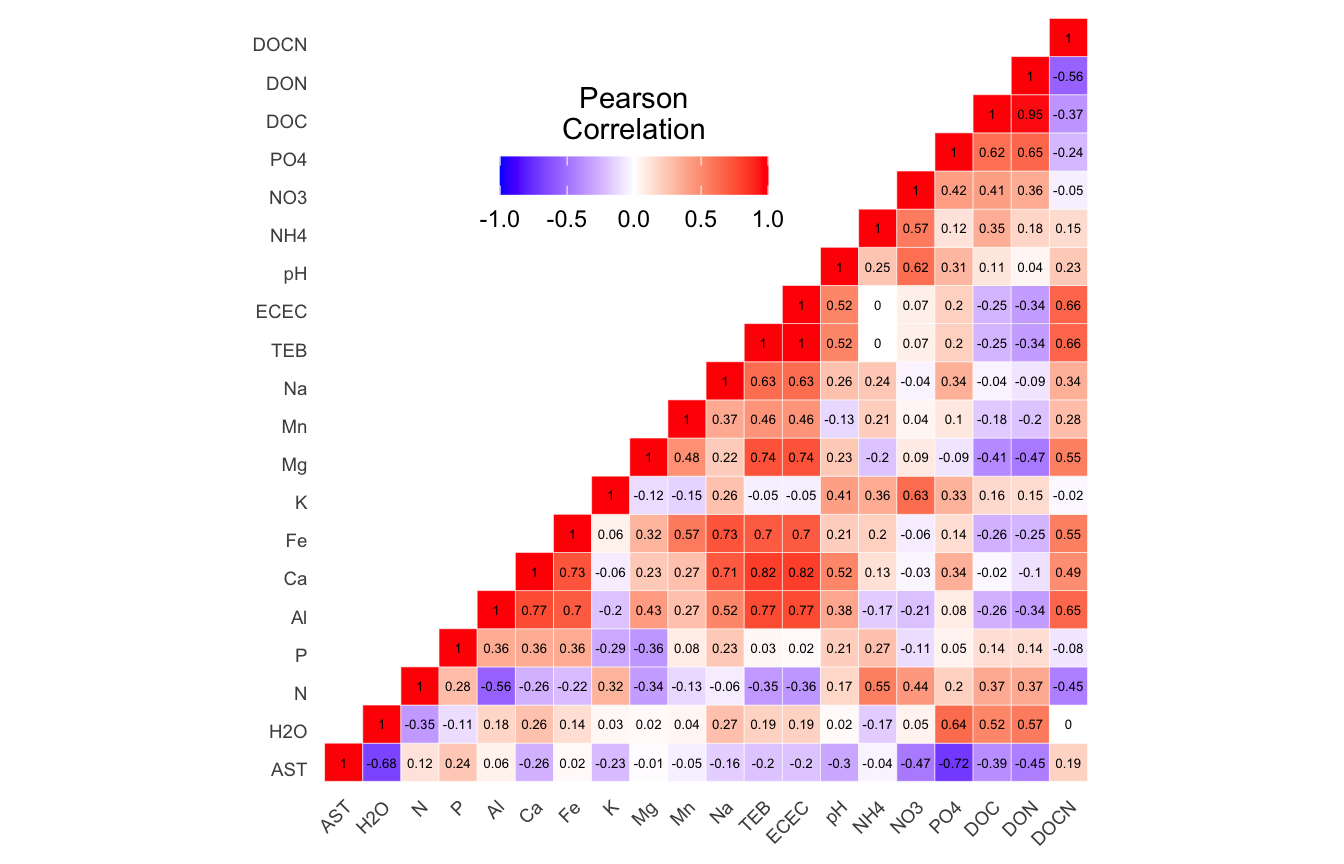(16S rRNA) Figure 1 | Results of autocorrelation analysis for non-normalized Environmental and edaphic parameters.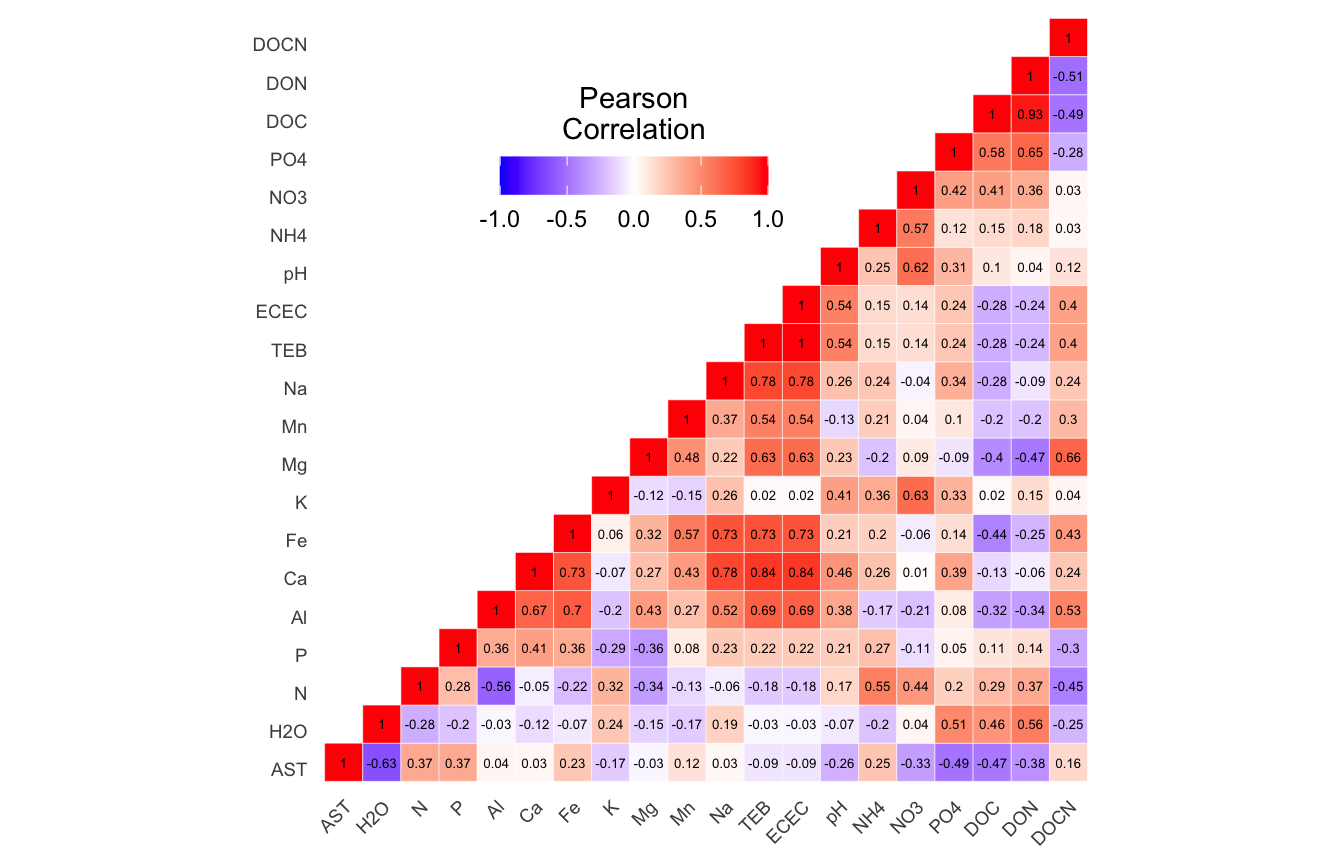(16S rRNA) Figure 2 | Results of autocorrelation analysis for normalized Environmental and edaphic parameters.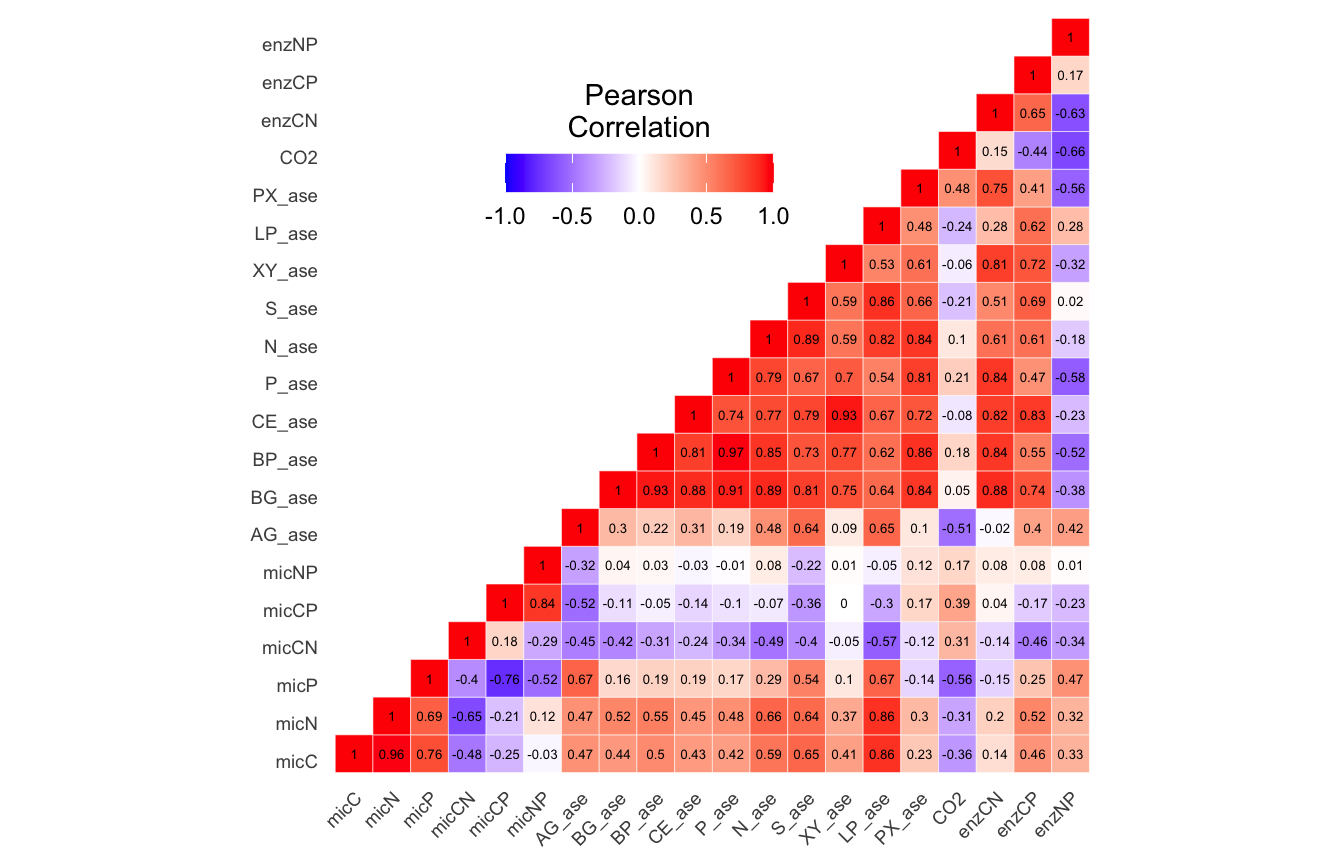(16S rRNA) Figure 3 | Results of autocorrelation analysis for non-normalized Functional response parameters.(16S rRNA) Figure 4 | Results of autocorrelation analysis for normalized Functional response parameters.(16S rRNA) Figure 5 | Results of autocorrelation analysis for non-normalized Temperature adaptation parameters.(16S rRNA) Figure 6 | Results of autocorrelation analysis for normalized Temperature adaptation parameters.

Now we can remove parameters based on the autocorrelation analysis:

1. Environmental and edaphic properties: TEB, DON, Na, Al, Ca.
2. Microbial functional responses: micN, micNP, enzCN, enzCP, BPase, CEase, LPase, Nase, Pase.
3. Temperature adaptation properties: NUE, PUE, SI.
``````edaphic_remove <- c("TEB", "DON", "Na", "Al", "Ca")
soil_funct_remove <- c("micN", "micNP", "enzCN", "enzCP", "BP_ase",
"CE_ase", "LP_ase", "N_ase", "P_ase")
Code
``````tmp_df <- ssu18_select_mc_split
tmp_df\$edaphic <- tmp_df\$edaphic[, ! names(tmp_df\$edaphic) %in%  edaphic_remove]
tmp_df\$soil_funct <- tmp_df\$soil_funct[, ! names(tmp_df\$soil_funct) %in%  soil_funct_remove]
ssu18_select_mc_split_no_ac <- tmp_df
rm(list = ls(pattern = "tmp_"))

tmp_df <- ssu18_select_mc_norm_split
tmp_df\$edaphic <- tmp_df\$edaphic[, ! names(tmp_df\$edaphic) %in%  edaphic_remove]
tmp_df\$soil_funct <- tmp_df\$soil_funct[, ! names(tmp_df\$soil_funct) %in%  soil_funct_remove]
ssu18_select_mc_norm_split_no_ac <- tmp_df
rm(list = ls(pattern = "tmp_"))``````

## Dissimilarity Correlation Tests

Let’s see if any on the metadata groups are significantly correlated with the community data. Basically, we create distance matrices for the community data and each metadata group and then run Mantel tests for all comparisons. For the community data we calculate Bray-Curtis distances for the community data and Euclidean distances for the metadata. We use the function `mantel.test` from the `ape` package and `mantel` from the `vegan` package for the analyses.

In summary, we test both `mantel.test` and `mantel` on Bray-Curtis distance community distances against Euclidean distances for each metadata group (`edaphic`, `soil_funct`, `temp_adapt`) a) before normalizing and before removing autocorrelated parameters, b) before normalizing and after removing autocorrelated parameters, c) after normalizing and before removing autocorrelated parameters, and d) after normalizing and after removing autocorrelated parameters.

Code
``````man_df <- c("ssu18_select_mc_split", "ssu18_select_mc_split_no_ac",
"ssu18_select_mc_norm_split", "ssu18_select_mc_norm_split_no_ac")

for (i in man_df) {
tmp_get <- get(i)
method = "bray", binary = FALSE,
diag = TRUE, upper = TRUE, na.rm = FALSE))
# EDAPHIC
tmp_dm_md_edaphic <- as.matrix(vegdist(tmp_get\$edaphic[, 8:ncol(tmp_get\$edaphic)],
method ="euclidean", binary = FALSE,
diag = TRUE, upper = TRUE, na.rm = FALSE))

tmp_man1_edaphic <- mantel.test(tmp_dm_otu, tmp_dm_md_edaphic, nperm = 999,
graph = FALSE, alternative = "two.sided")
tmp_man2_edaphic <- mantel(tmp_dm_otu, tmp_dm_md_edaphic, permutations = 999)
# SOIL FUNCT
tmp_dm_md_soil_funct <- as.matrix(vegdist(tmp_get\$soil_funct[, 8:ncol(tmp_get\$soil_funct)],
method = "euclidean", binary = FALSE,
diag = TRUE, upper = TRUE, na.rm = FALSE))

tmp_man1_soil_funct <- mantel.test(tmp_dm_otu, tmp_dm_md_soil_funct, nperm = 999,
graph = FALSE, alternative = "two.sided")
tmp_man2_soil_funct <- mantel(tmp_dm_otu, tmp_dm_md_soil_funct, permutations = 999)

method = "euclidean", binary = FALSE,
diag = TRUE, upper = TRUE, na.rm = FALSE))

graph = FALSE, alternative = "two.sided")

tmp_name <- purrr::map_chr(i, ~ paste0(., "_mantel_tests"))
tmp_df <- list(edaphic_ape_man = tmp_man1_edaphic,
edaphic_vegan_man = tmp_man2_edaphic,
soil_funct_ape_man = tmp_man1_soil_funct,
soil_funct_vegan_man = tmp_man2_soil_funct,
assign(tmp_name, tmp_df)
print(tmp_name)
rm(list = ls(pattern = "tmp_"))
}``````

### Dissimilarity Correlation Results

(16S rRNA) Table 4 | Summary of Dissimilarity Correlation Tests using `mantel.test` from the ape package and `mantel` from the vegan. P-values in red indicate significance (p-value < 0.05)

Moving on.

## Best Subset of Variables

Now we want to know which of the metadata parameters are the most strongly correlated with the community data. For this we use the `bioenv` function from the `vegan` package. `bioenv`Best Subset of Environmental Variables with Maximum (Rank) Correlation with Community Dissimilarities—finds the best subset of environmental variables, so that the Euclidean distances of scaled environmental variables have the maximum (rank) correlation with community dissimilarities.

Next we will use the metadata set where autocorrelated parameters were removed and the remainder of the parameters were normalized (where applicable based on the Shapiro tests).

We run `bioenv` against the three groups of metadata parameters. We then run `bioenv` again, but this time against the individual parameters identified as significantly correlated.

### Edaphic Properties

Code
``````tmp_comm <- data.frame(t(ssu18_select_mc_norm_split_no_ac\$data_loaded))
tmp_env <- data.frame(ssu18_select_mc_norm_split_no_ac\$edaphic)
tmp_env[,1:8] <- NULL

edaphic_bioenv <- bioenv(wisconsin(tmp_comm), tmp_env,
method = "spearman", index = "bray",
upto = ncol(tmp_env), metric = "euclidean")

bioenv_list <- edaphic_bioenv\$models[[edaphic_bioenv\$whichbest]]\$best
bioenv_best <- bioenvdist(edaphic_bioenv, which = "best")

for (i in bioenv_list) {
tmp_dp <- data.frame(edaphic_bioenv\$x)
tmp_md <- as.matrix(vegdist(tmp_dp[[i]],
method = "euclidean", binary = FALSE,
diag = TRUE, upper = TRUE, na.rm = FALSE))
tmp_man <- mantel(bioenv_best, tmp_md,
permutations = 999, method = "spearman")
tmp_md_name <- names(tmp_dp)[[i]]
tmp_name <- purrr::map_chr(tmp_md_name, ~ paste0(., "_bioenv_mantel_test"))
assign(tmp_name, tmp_man)
rm(list = ls(pattern = "tmp_"))
}
objects(pattern = "_bioenv_mantel_test")
edaphic_bioenv_ind_mantel <- list(AST = AST_bioenv_mantel_test)
rm(list = ls(pattern = "_bioenv_mantel_test"))``````
``````
Call:
bioenv(comm = wisconsin(tmp_comm), env = tmp_env, method = "spearman",      index = "bray", upto = ncol(tmp_env), metric = "euclidean")

Subset of environmental variables with best correlation to community data.

Correlations:    spearman
Dissimilarities: bray
Metric:          euclidean

Best model has 1 parameters (max. 15 allowed):
AST
with correlation  0.6808861 ``````
Show the results of individual edaphic metadata Mantel tests
``````\$AST

Mantel statistic based on Spearman's rank correlation rho

Call:
mantel(xdis = bioenv_best, ydis = tmp_md, method = "spearman",      permutations = 999)

Mantel statistic r:     1
Significance: 0.001

Upper quantiles of permutations (null model):
90%   95% 97.5%   99%
0.177 0.226 0.271 0.326
Permutation: free
Number of permutations: 999``````

`bioenv` found the following edaphic properties significantly correlated with the community data: AST

### Soil Functional Response

Code
``````tmp_comm <- data.frame(t(ssu18_select_mc_norm_split_no_ac\$data_loaded))
tmp_env <- data.frame(ssu18_select_mc_norm_split_no_ac\$soil_funct)
tmp_env[,1:8] <- NULL

soil_funct_bioenv <- bioenv(wisconsin(tmp_comm), tmp_env,
method = "spearman", index = "bray",
upto = ncol(tmp_env), metric = "euclidean")

bioenv_list <- soil_funct_bioenv\$models[[soil_funct_bioenv\$whichbest]]\$best
bioenv_best <- bioenvdist(soil_funct_bioenv, which = "best")

for (i in bioenv_list) {
tmp_dp <- data.frame(soil_funct_bioenv\$x)
tmp_md <- as.matrix(vegdist(tmp_dp[[i]],
method = "euclidean", binary = FALSE,
diag = TRUE, upper = TRUE, na.rm = FALSE))
tmp_man <- mantel(bioenv_best, tmp_md,
permutations = 999, method = "spearman")
tmp_md_name <- names(tmp_dp)[[i]]
tmp_name <- purrr::map_chr(tmp_md_name, ~ paste0(., "_bioenv_mantel_test"))
assign(tmp_name, tmp_man)
rm(list = ls(pattern = "tmp_"))
}
objects(pattern = "_bioenv_mantel_test")

soil_funct_bioenv_ind_mantel <- list(AG_ase = AG_ase_bioenv_mantel_test,
enzNP = enzNP_bioenv_mantel_test,
S_ase = S_ase_bioenv_mantel_test,
PX_ase = PX_ase_bioenv_mantel_test,
XY_ase = XY_ase_bioenv_mantel_test)
rm(list = ls(pattern = "_bioenv_mantel_test"))``````
``````
Call:
bioenv(comm = wisconsin(tmp_comm), env = tmp_env, method = "spearman",      index = "bray", upto = ncol(tmp_env), metric = "euclidean")

Subset of environmental variables with best correlation to community data.

Correlations:    spearman
Dissimilarities: bray
Metric:          euclidean

Best model has 5 parameters (max. 11 allowed):
AG_ase S_ase XY_ase PX_ase enzNP
with correlation  0.7727866 ``````
Show the results of individual functional response metadata Mantel tests
``````\$AG_ase

Mantel statistic based on Spearman's rank correlation rho

Call:
mantel(xdis = bioenv_best, ydis = tmp_md, method = "spearman",      permutations = 999)

Mantel statistic r: 0.5588
Significance: 0.001

Upper quantiles of permutations (null model):
90%   95% 97.5%   99%
0.186 0.235 0.281 0.330
Permutation: free
Number of permutations: 999

\$enzNP

Mantel statistic based on Spearman's rank correlation rho

Call:
mantel(xdis = bioenv_best, ydis = tmp_md, method = "spearman",      permutations = 999)

Mantel statistic r: 0.4623
Significance: 0.006

Upper quantiles of permutations (null model):
90%   95% 97.5%   99%
0.219 0.280 0.336 0.413
Permutation: free
Number of permutations: 999

\$S_ase

Mantel statistic based on Spearman's rank correlation rho

Call:
mantel(xdis = bioenv_best, ydis = tmp_md, method = "spearman",      permutations = 999)

Mantel statistic r: 0.6139
Significance: 0.001

Upper quantiles of permutations (null model):
90%   95% 97.5%   99%
0.223 0.305 0.384 0.466
Permutation: free
Number of permutations: 999

\$PX_ase

Mantel statistic based on Spearman's rank correlation rho

Call:
mantel(xdis = bioenv_best, ydis = tmp_md, method = "spearman",      permutations = 999)

Mantel statistic r: 0.6115
Significance: 0.001

Upper quantiles of permutations (null model):
90%   95% 97.5%   99%
0.176 0.222 0.289 0.346
Permutation: free
Number of permutations: 999

\$XY_ase

Mantel statistic based on Spearman's rank correlation rho

Call:
mantel(xdis = bioenv_best, ydis = tmp_md, method = "spearman",      permutations = 999)

Mantel statistic r: 0.456
Significance: 0.002

Upper quantiles of permutations (null model):
90%   95% 97.5%   99%
0.189 0.258 0.304 0.347
Permutation: free
Number of permutations: 999``````

`bioenv` found the following soil functions significantly correlated with the community data: AG_ase, enzNP, S_ase, PX_ase, XY_ase

Code
``````tmp_comm <- data.frame(t(ssu18_select_mc_norm_split_no_ac\$data_loaded))
tmp_env[,1:8] <- NULL

method = "spearman", index = "bray",
upto = ncol(tmp_env), metric = "euclidean")

bioenv_best <- bioenvdist(temp_adapt_bioenv, which = "best")

for (i in bioenv_list) {
tmp_md <- as.matrix(vegdist(tmp_dp[[i]],
method = "euclidean", binary = FALSE,
diag = TRUE, upper = TRUE, na.rm = FALSE))
tmp_man <- mantel(bioenv_best, tmp_md,
permutations = 999, method = "spearman")
tmp_md_name <- names(tmp_dp)[[i]]
tmp_name <- purrr::map_chr(tmp_md_name, ~ paste0(., "_bioenv_mantel_test"))
assign(tmp_name, tmp_man)
rm(list = ls(pattern = "tmp_"))
}
objects(pattern = "_bioenv_mantel_test")

LP_Q10 = LP_Q10_bioenv_mantel_test,
P_Q10 = P_Q10_bioenv_mantel_test,
S_Q10 = S_Q10_bioenv_mantel_test,
Tmin = Tmin_bioenv_mantel_test)
rm(list = ls(pattern = "_bioenv_mantel_test"))``````
``````
Call:
bioenv(comm = wisconsin(tmp_comm), env = tmp_env, method = "spearman",      index = "bray", upto = ncol(tmp_env), metric = "euclidean")

Subset of environmental variables with best correlation to community data.

Correlations:    spearman
Dissimilarities: bray
Metric:          euclidean

Best model has 5 parameters (max. 13 allowed):
P_Q10 S_Q10 LP_Q10 CUEcp Tmin
with correlation  0.4237093 ``````
``````\$CUEcp

Mantel statistic based on Spearman's rank correlation rho

Call:
mantel(xdis = bioenv_best, ydis = tmp_md, method = "spearman",      permutations = 999)

Mantel statistic r: 0.325
Significance: 0.013

Upper quantiles of permutations (null model):
90%   95% 97.5%   99%
0.188 0.226 0.280 0.331
Permutation: free
Number of permutations: 999

\$LP_Q10

Mantel statistic based on Spearman's rank correlation rho

Call:
mantel(xdis = bioenv_best, ydis = tmp_md, method = "spearman",      permutations = 999)

Mantel statistic r: 0.3772
Significance: 0.005

Upper quantiles of permutations (null model):
90%   95% 97.5%   99%
0.203 0.272 0.306 0.351
Permutation: free
Number of permutations: 999

\$P_Q10

Mantel statistic based on Spearman's rank correlation rho

Call:
mantel(xdis = bioenv_best, ydis = tmp_md, method = "spearman",      permutations = 999)

Mantel statistic r: 0.5177
Significance: 0.001

Upper quantiles of permutations (null model):
90%   95% 97.5%   99%
0.158 0.211 0.261 0.309
Permutation: free
Number of permutations: 999

\$S_Q10

Mantel statistic based on Spearman's rank correlation rho

Call:
mantel(xdis = bioenv_best, ydis = tmp_md, method = "spearman",      permutations = 999)

Mantel statistic r: 0.4395
Significance: 0.001

Upper quantiles of permutations (null model):
90%   95% 97.5%   99%
0.159 0.205 0.252 0.301
Permutation: free
Number of permutations: 999

\$Tmin

Mantel statistic based on Spearman's rank correlation rho

Call:
mantel(xdis = bioenv_best, ydis = tmp_md, method = "spearman",      permutations = 999)

Mantel statistic r: 0.4044
Significance: 0.005

Upper quantiles of permutations (null model):
90%   95% 97.5%   99%
0.207 0.267 0.305 0.359
Permutation: free
Number of permutations: 999``````

`bioenv` found the following temperature adaptations significantly correlated with the community data: CUEcp, LP_Q10, P_Q10, S_Q10, Tmin

## Distance-based Redundancy

Now we turn our attention to distance-based redundancy analysis (dbRDA), an ordination method similar to Redundancy Analysis (rda) but it allows non-Euclidean dissimilarity indices, such as Manhattan or Bray–Curtis distance. For this, we use `capscale` from the `vegan` package. `capscale` is a constrained versions of metric scaling (principal coordinates analysis), which are based on the Euclidean distance but can be used, and are more useful, with other dissimilarity measures. The functions can also perform unconstrained principal coordinates analysis, optionally using extended dissimilarities.

For each of the three metadata subsets, we perform the following steps:

1. Run `rankindex` to compare metadata and community dissimilarity indices for gradient detection. This will help us select the best dissimilarity metric to use.
2. Run `capscale` for distance-based redundancy analysis.
3. Run `envfit` to fit environmental parameters onto the ordination. This function basically calculates correlation scores between the metadata parameters and the ordination axes.
4. Select metadata parameters significant for `bioenv` (see above) and/or `envfit` analyses.
5. Run `envfit` on ASVs.
6. Plot the ordination and vector overlays.

### Edaphic Properties

Code
``````tmp_md <- ssu18_select_mc_norm_split_no_ac\$edaphic
tmp_md\$TREAT_T <- as.character(tmp_md\$TREAT_T)
edaphic_rank <- rankindex(tmp_md[, 8:ncol(tmp_md)], tmp_comm,
indices = c("euc", "man", "gow","bra", "kul"),
stepacross = FALSE, method = "spearman")``````
``````      euc       man       gow       bra       kul
0.3171263 0.4336927 0.1028302 0.4336927 0.4336927 ``````

Let’s run `capscale` using Bray-Curtis. Note, we have 15 metadata parameters in this group but, for some reason, `capscale` only works with 13 parameters. This may have to do with degrees of freedom?

• Starting properties: AST, H2O, N, P, Al, Ca, Fe, K, Mg, Mn, Na, TEB, ECEC, pH, NH4, NO3, PO4, DOC, DON, DOCN
• Autocorrelated removed: TEB, DON, Na, Al, Ca
• Remove for capscale: Mg, Mn
``````edaphic_cap <- capscale(tmp_comm ~ AST + H2O + N + P + Fe + K + ECEC +
pH + NH4 + NO3 + PO4 + DOC + DOCN,
tmp_md, dist = "bray")
colnames(tmp_md)``````
``````Call: capscale(formula = tmp_comm ~ AST + H2O + N + P + Fe + K + ECEC +
pH + NH4 + NO3 + PO4 + DOC + DOCN, data = tmp_md, distance = "bray")

Inertia Proportion Rank
Total         1.67890    1.00000
Constrained   1.63037    0.97110   13
Unconstrained 0.04853    0.02890    1
Inertia is squared Bray distance
Species scores projected from 'tmp_comm'

Eigenvalues for constrained axes:
CAP1   CAP2   CAP3   CAP4   CAP5   CAP6   CAP7   CAP8   CAP9  CAP10  CAP11
0.6287 0.2955 0.1984 0.1104 0.1085 0.0661 0.0536 0.0511 0.0359 0.0277 0.0247
CAP12  CAP13
0.0218 0.0080

Eigenvalues for unconstrained axes:
MDS1
0.04853 ``````

Now we can look at the variance against each principal component.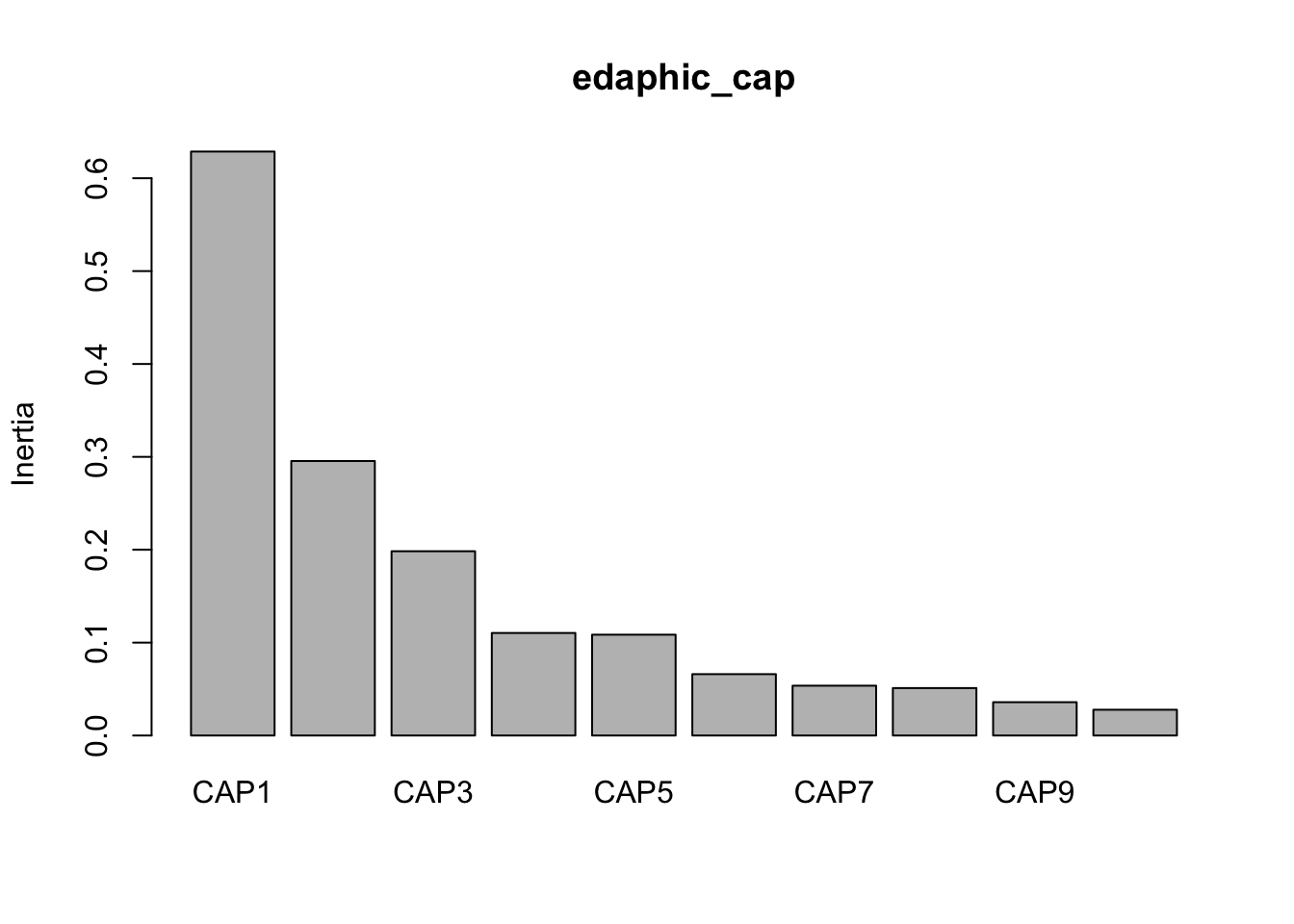And then make some quick and dirty plots. This will also come in handy later when we need to parse out data a better plot visualization. The `ggplot` function `autoplot` stores these data in a more accessible way than the raw results from `capscale`

``base::plot(edaphic_cap) ``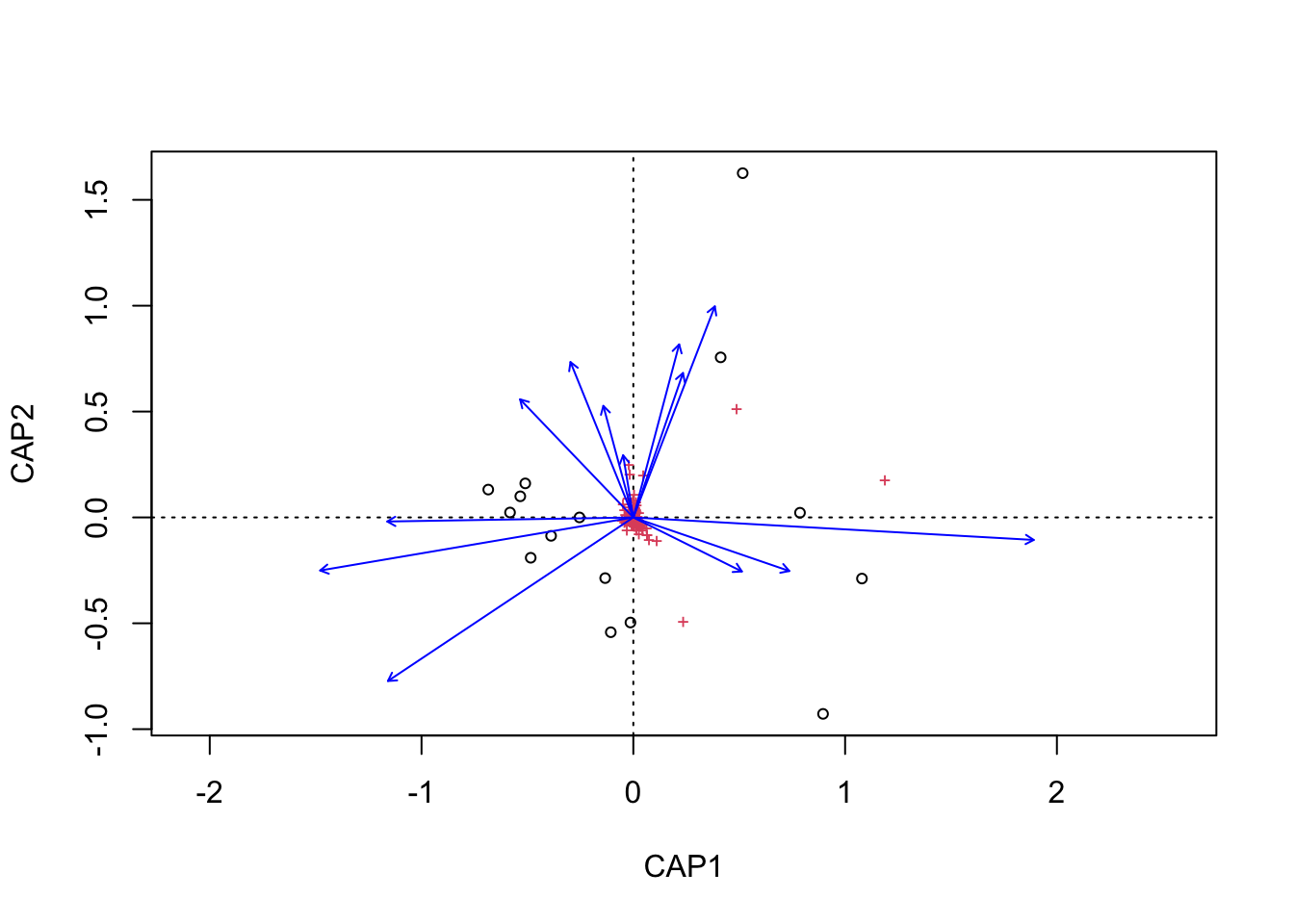``````tmp_auto_plt <- ggplot2::autoplot(edaphic_cap, arrows = TRUE)
tmp_auto_plt``````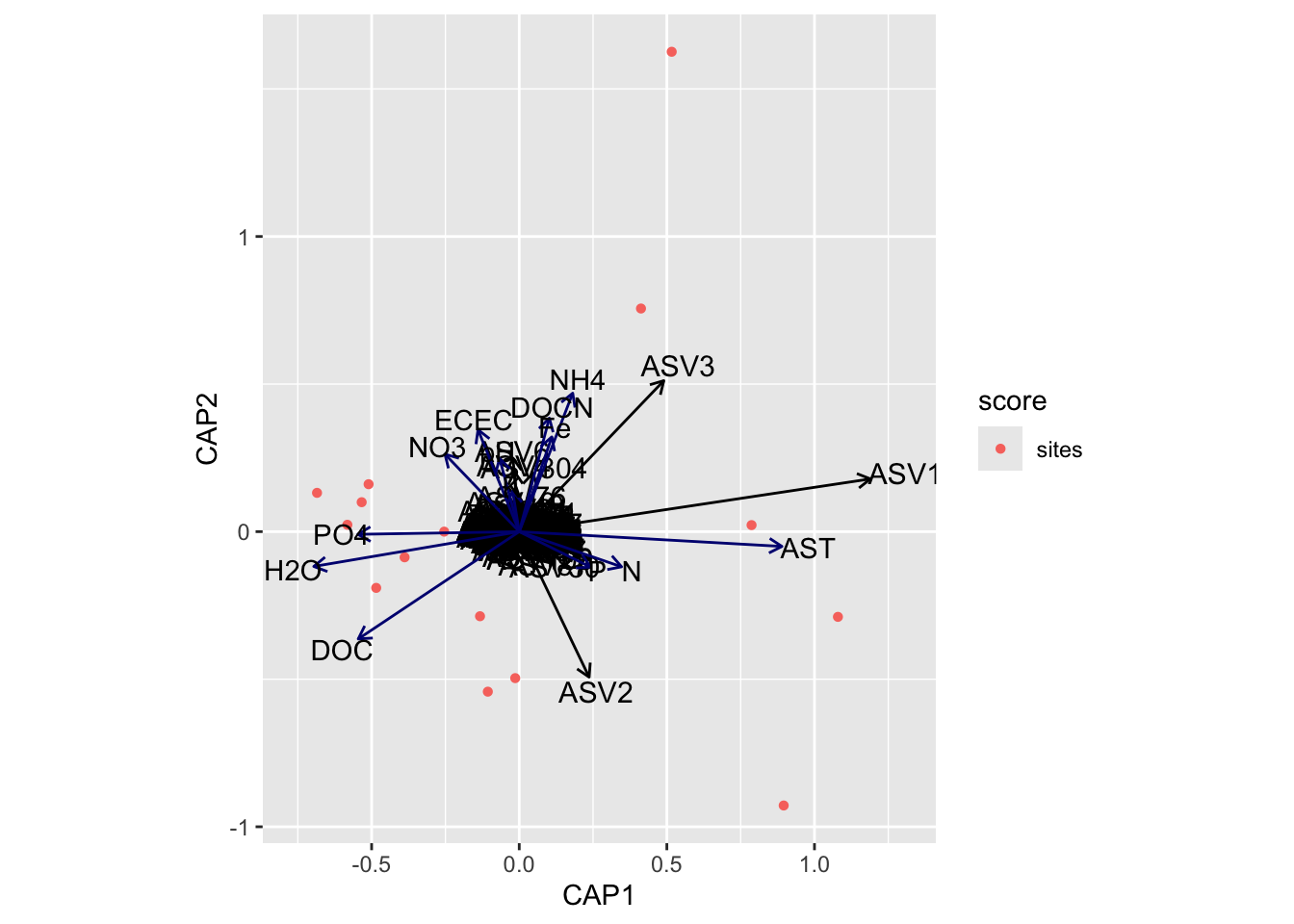Next, we need to grab capscale scores for the samples and create a data frame of the first two dimensions. We will also need to add some of the sample details to the data frame. For this we use the vegan function `scores` which gets species or site scores from the ordination.

Code
``````tmp_samp_scores <- dplyr::filter(tmp_auto_plt\$plot_env\$obj, Score == "sites")
tmp_samp_scores[,1] <- NULL
tmp_samp_scores <- tmp_samp_scores %>% dplyr::rename(SampleID = Label)

tmp_md_sub <- tmp_md[, 1:4]
tmp_md_sub <- tmp_md_sub %>% tibble::rownames_to_column("SampleID")
edaphic_plot_data <- dplyr::left_join(tmp_md_sub, tmp_samp_scores, by = "SampleID")``````

Now we have a new data frame that contains sample details and capscale values.

``````          SampleID PLOT TREAT TREAT_T PAIR        CAP1           CAP2
1  P10_D00_010_C0E  P10     C       0    E -0.51023381  0.16071056348
2  P02_D00_010_C0A  P02     C       0    A -0.48448722 -0.19055366181
3  P04_D00_010_C0B  P04     C       0    B -0.58218619  0.02325887477
4  P06_D00_010_C0C  P06     C       0    C -0.53370906  0.09973976767
5  P08_D00_010_C0D  P08     C       0    D -0.68492943  0.13143857676
6  P01_D00_010_W3A  P01     W       3    A -0.13286443 -0.28661650806
7  P03_D00_010_W3B  P03     W       3    B -0.25415783  0.00002258673
8  P05_D00_010_W3C  P05     W       3    C -0.38829574 -0.08695154559
9  P07_D00_010_W3D  P07     W       3    D -0.01360582 -0.49632449304
10 P09_D00_010_W3E  P09     W       3    E -0.10639798 -0.54219706978
11 P01_D00_010_W8A  P01     W       8    A  0.78689725  0.02214444812
12 P03_D00_010_W8B  P03     W       8    B  0.89574754 -0.92786190328
13 P05_D00_010_W8C  P05     W       8    C  1.07945322 -0.28874425650
14 P07_D00_010_W8D  P07     W       8    D  0.41234456  0.75597843926
15 P09_D00_010_W8E  P09     W       8    E  0.51642495  1.62595618128``````

We can then do the same with the metadata vectors. Here though we only need the scores and parameter name.

Code
``````edaphic_md_scores <- dplyr::filter(tmp_auto_plt\$plot_env\$obj, Score == "biplot")
edaphic_md_scores[,1] <- NULL
edaphic_md_scores <- edaphic_md_scores %>%
dplyr::mutate(parameters = Label, .before = CAP1) %>%
tibble::column_to_rownames("Label")``````
``````     parameters        CAP1         CAP2
AST         AST  0.97882379 -0.055074169
H2O         H2O -0.76548607 -0.129779379
N             N  0.38130076 -0.131035479
P             P  0.26536024 -0.132125558
Fe           Fe  0.12138586  0.353093177
K             K -0.02490580  0.151975508
ECEC       ECEC -0.15365335  0.379848234
pH           pH -0.07327989  0.272676813
NH4         NH4  0.19922925  0.516074241
NO3         NO3 -0.27681227  0.288729283
PO4         PO4 -0.60140669 -0.009980058
DOC         DOC -0.59979130 -0.399930429
DOCN       DOCN  0.11210058  0.422975484``````

Let’s run some quick correlations of metadata with ordination axes to see which parameters are significant. For this we use the vegan function `envfit`.

Code
``````tmp_samp_scores_sub <- edaphic_plot_data[, 6:7]
tmp_samp_scores_sub <- as.matrix(tmp_samp_scores_sub)
tmp_param_list <- edaphic_md_scores\$parameters

tmp_md_sub <- subset(tmp_md,  select =  tmp_param_list)

envfit_edaphic_md <- envfit(tmp_samp_scores_sub, tmp_md_sub,
perm = 1000, choices = c(1, 2))``````
``````
***VECTORS

CAP1     CAP2     r2   Pr(>r)
AST   0.99825 -0.05912 0.8287 0.000999 ***
H2O  -0.98657 -0.16333 0.5189 0.009990 **
N     0.94537 -0.32599 0.1403 0.398601
P     0.89518 -0.44571 0.0759 0.602398
Fe    0.32411  0.94602 0.1194 0.432567
K    -0.16554  0.98620 0.0204 0.890110
ECEC -0.37874  0.92550 0.1444 0.368631
pH   -0.26344  0.96468 0.0685 0.672328
NH4   0.35944  0.93317 0.2621 0.152847
NO3  -0.69392  0.72006 0.1380 0.417582
PO4  -0.99991 -0.01327 0.3118 0.090909 .
DOC  -0.83396 -0.55183 0.4459 0.023976 *
DOCN  0.25463  0.96704 0.1640 0.318681
---
Signif. codes:  0 '***' 0.001 '**' 0.01 '*' 0.05 '.' 0.1 ' ' 1
Permutation: free
Number of permutations: 1000``````
Code
``````edaphic_md_signif_hits <- base::subset(envfit_edaphic_md\$vectors\$pvals,
c(envfit_edaphic_md\$vectors\$pvals
< 0.05 & envfit_edaphic_md\$vectors\$r > 0.4))
edaphic_md_signif_hits <- data.frame(edaphic_md_signif_hits)
edaphic_md_signif_hits <- rownames(edaphic_md_signif_hits)
edaphic_md_signif <- edaphic_md_scores[edaphic_md_scores\$parameters %in% edaphic_md_signif_hits,]
edaphic_md_signif\$parameters``````

`envfit` found that AST, H2O, DOC were significantly correlated.

Now let’s see if the same parameters are significant for the `envfit` and `bioenv` analyses.

`````` "Significant parameters from bioenv analysis."
 "AST"
_____________________________________
 "Significant parameters from envfit analysis."
 "AST" "H2O" "DOC"
_____________________________________
 "Found in bioenv but not envfit."
character(0)
_____________________________________
 "Found in envfit but not bioenv."
 "H2O" "DOC"
_____________________________________
 "Found in envfit and bioenv."
 "AST" "H2O" "DOC"``````
Code
``````new_edaphic_md_signif_hits <- edaphic_sig_diff
#new_edaphic_md_signif_hits <- append(edaphic_md_signif_hits, edaphic_sig_diff)
edaphic_md_signif_all <- edaphic_md_scores[edaphic_md_scores\$parameters %in% new_edaphic_md_signif_hits,]``````

Check. Next, we run `envfit` for the ASVs.

Code
``````envfit_edaphic_asv <- envfit(tmp_samp_scores_sub,
tmp_comm[, order(colSums(-tmp_comm))][, 1:10],
perm = 1000, choices = c(1, 2))
edaphic_asv_scores <- dplyr::filter(tmp_auto_plt\$plot_env\$obj, Score == "species")
edaphic_asv_scores <- edaphic_asv_scores %>%
dplyr::mutate(parameters = Label, .before = CAP1) %>%
tibble::column_to_rownames("Label")
edaphic_asv_scores[,1] <- NULL``````
``````
***VECTORS

CAP1     CAP2     r2   Pr(>r)
ASV1   0.99502  0.09969 0.6491 0.005994 **
ASV2   0.54850 -0.83615 0.8984 0.000999 ***
ASV3   0.79245  0.60994 0.9429 0.000999 ***
ASV4  -0.12958  0.99157 0.2913 0.120879
ASV5  -0.56363 -0.82603 0.1586 0.362637
ASV6  -0.10651  0.99431 0.7559 0.000999 ***
ASV8  -0.84329  0.53746 0.4370 0.030969 *
ASV7  -0.52510 -0.85104 0.0681 0.655345
ASV9   0.51828 -0.85521 0.2559 0.185814
ASV10 -0.34456  0.93876 0.8331 0.000999 ***
---
Signif. codes:  0 '***' 0.001 '**' 0.01 '*' 0.05 '.' 0.1 ' ' 1
Permutation: free
Number of permutations: 1000``````
Code
``````edaphic_asv_signif_hits <- base::subset(envfit_edaphic_asv\$vectors\$pvals,
c(envfit_edaphic_asv\$vectors\$pvals
< 0.05 & envfit_edaphic_asv\$vectors\$r > 0.5))
edaphic_asv_signif_hits <- data.frame(edaphic_asv_signif_hits)
edaphic_asv_signif_hits <- rownames(edaphic_asv_signif_hits)
edaphic_asv_signif <- edaphic_asv_scores[edaphic_asv_scores\$parameters %in% edaphic_asv_signif_hits,]``````
``````      parameters        CAP1        CAP2 variable_type
ASV3        ASV3  0.48974117  0.51152737           ASV
ASV6        ASV6 -0.02123424  0.24919713           ASV
ASV2        ASV2  0.23670463 -0.49287683           ASV
ASV10      ASV10 -0.02578511  0.09240257           ASV
ASV1        ASV1  1.18949655  0.17867402           ASV``````
Code
``````edaphic_md_signif_all\$variable_type <- "metadata"
edaphic_asv_signif\$variable_type <- "ASV"
edaphic_bioplot_data <- rbind(edaphic_md_signif_all, edaphic_asv_signif)``````

The last thing to do is categorize parameters scores and ASV scores into different variable types for plotting.

Code
``````edaphic_bioplot_data_md <- subset(edaphic_bioplot_data,
edaphic_bioplot_data_asv <- subset(edaphic_bioplot_data,
edaphic_bioplot_data\$variable_type == "ASV")``````
Show the code for the plot

### Soil Functional Response

Code
``````tmp_md <- ssu18_select_mc_norm_split_no_ac\$soil_funct
tmp_md\$TREAT_T <- as.character(tmp_md\$TREAT_T)
soil_funct_rank <- rankindex(tmp_md[, 8:ncol(tmp_md)], tmp_comm,
indices = c("euc", "man", "gow","bra", "kul"),
stepacross = FALSE, method = "spearman")``````
``````      euc       man       gow       bra       kul
0.5840452 0.7341800 0.2168775 0.7341800 0.7341800 ``````

Let’s run `capscale` using Bray-Curtis. Note, we have 11 metadata parameters in this group but, for some reason, `capscale` only works with 13 parameters. This may have to do with degrees of freedom?

• Starting properties: micC, micN, micP, micCN, micCP, micNP, AG_ase, BG_ase, BP_ase, CE_ase, P_ase, N_ase, S_ase, XY_ase, LP_ase, PX_ase, CO2, enzCN, enzCP, enzNP
• Autocorrelated removed: micN, micNP, enzCN, enzCP, BP_ase, CE_ase, LP_ase, N_ase, P_ase
• Remove for capscale: NONE
``````soil_funct_cap <- capscale(tmp_comm ~ micC + micP + micCN + micCP + AG_ase + BG_ase +
S_ase + XY_ase + PX_ase + CO2 + enzNP,
tmp_md, dist = "bray")``````
``````Call: capscale(formula = tmp_comm ~ micC + micP + micCN + micCP +
AG_ase + BG_ase + S_ase + XY_ase + PX_ase + CO2 + enzNP, data = tmp_md,
distance = "bray")

Inertia Proportion Rank
Total         1.67890    1.00000
Constrained   1.52672    0.90936   11
Unconstrained 0.15218    0.09064    3
Inertia is squared Bray distance
Species scores projected from 'tmp_comm'

Eigenvalues for constrained axes:
CAP1   CAP2   CAP3   CAP4   CAP5   CAP6   CAP7   CAP8   CAP9  CAP10  CAP11
0.6202 0.2950 0.1827 0.1164 0.0950 0.0555 0.0491 0.0468 0.0290 0.0246 0.0126

Eigenvalues for unconstrained axes:
MDS1    MDS2    MDS3
0.06264 0.05568 0.03386 ``````

Now we can look at the variance against each principal component.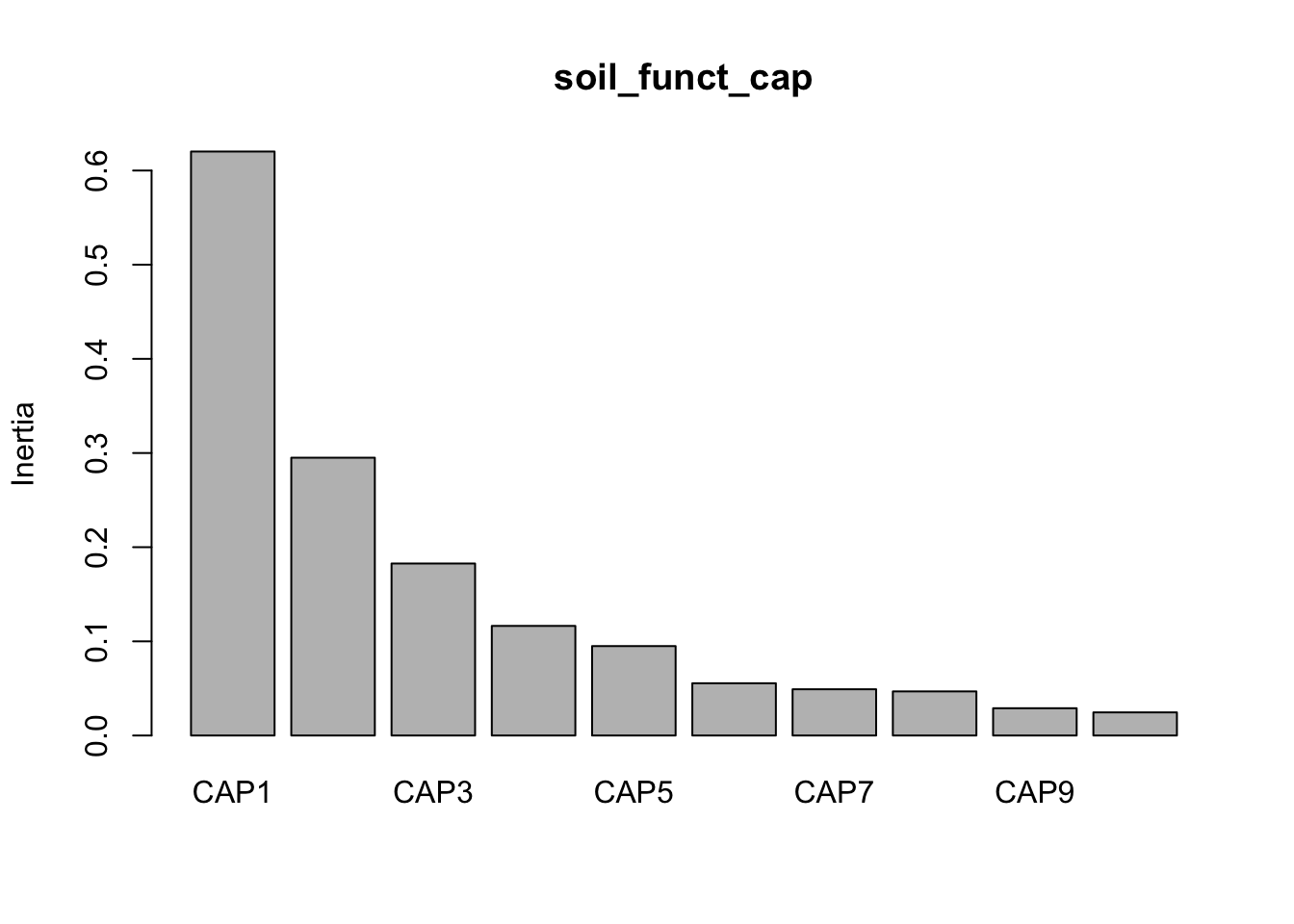And then make some quick and dirty plots. This will also come in handy later when we need to parse out data a better plot visualization. The `ggplot` function `autoplot` stores these data in a more accessible way than the raw results from `capscale`

``base::plot(soil_funct_cap) ``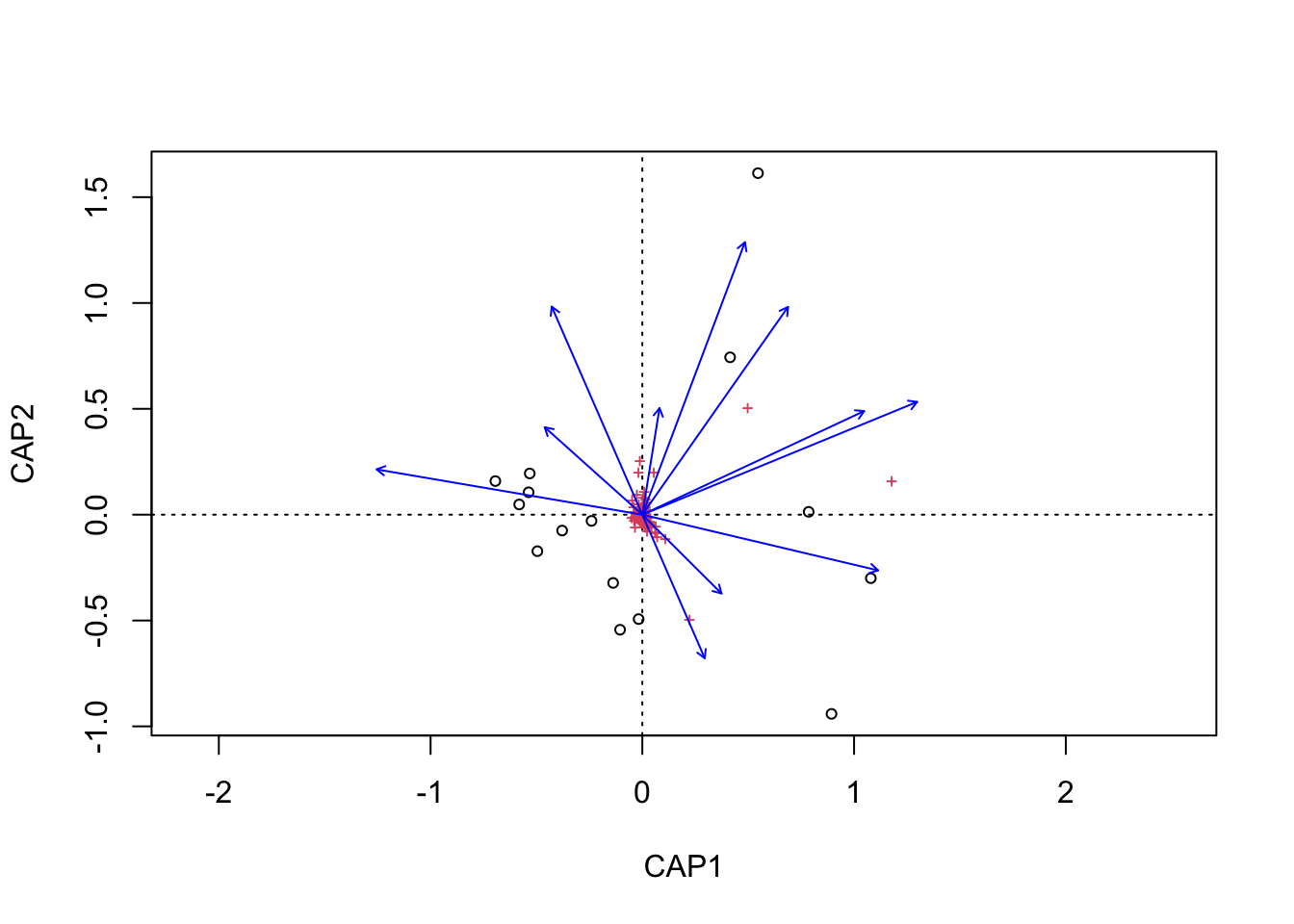``````tmp_auto_plt <- autoplot(soil_funct_cap, arrows = TRUE)
tmp_auto_plt``````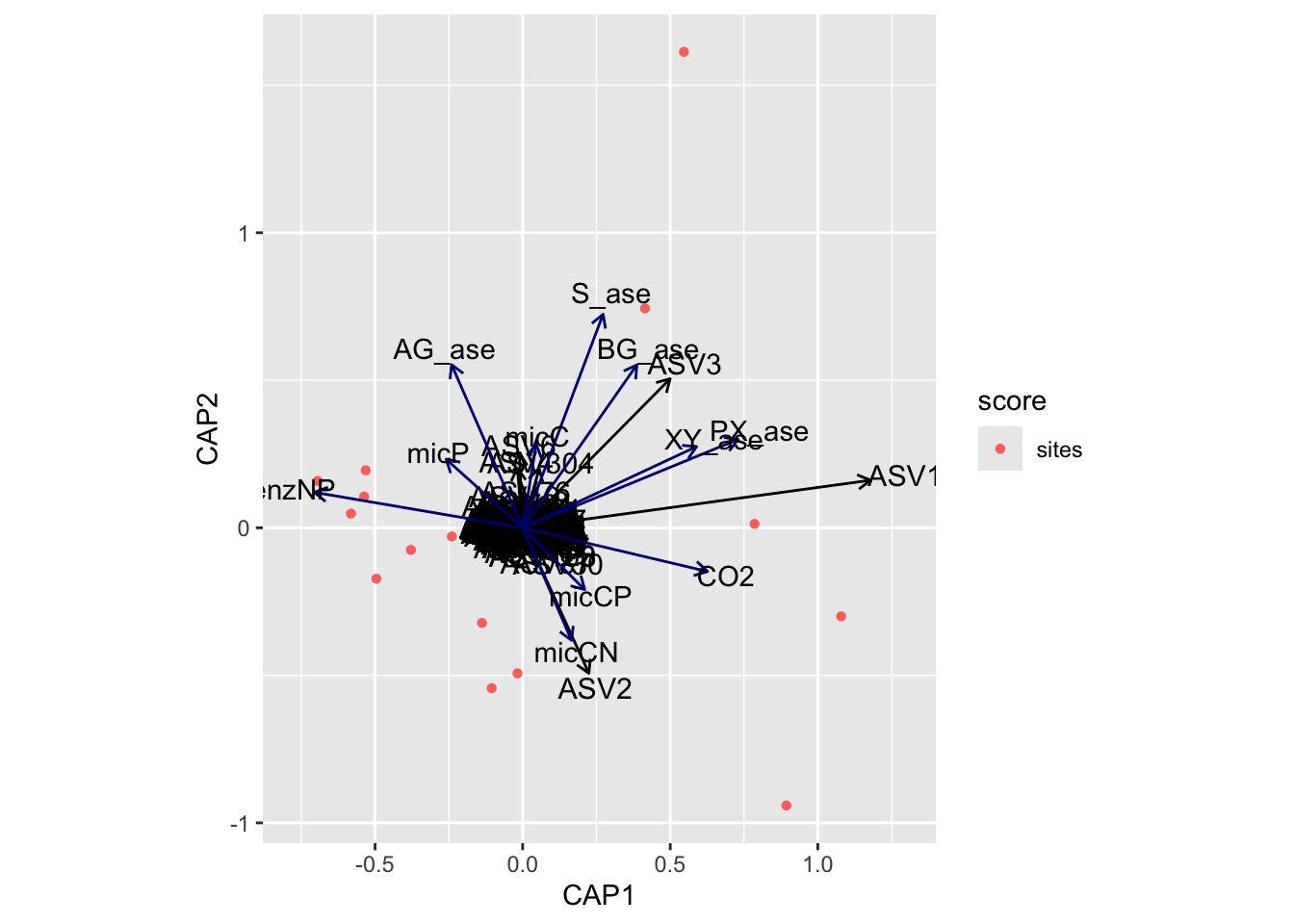Next, we need to grab capscale scores for the samples and create a data frame of the first two dimensions. We will also need to add some of the sample details to the data frame. For this we use the vegan function `scores` which gets species or site scores from the ordination.

Code
``````tmp_samp_scores <- dplyr::filter(tmp_auto_plt\$plot_env\$obj, Score == "sites")
tmp_samp_scores[,1] <- NULL
tmp_samp_scores <- tmp_samp_scores %>% dplyr::rename(SampleID = Label)

tmp_md_sub <- tmp_md[, 1:4]
tmp_md_sub <- tmp_md_sub %>% tibble::rownames_to_column("SampleID")
soil_funct_plot_data <- dplyr::left_join(tmp_md_sub, tmp_samp_scores,
by = "SampleID")``````

Now we have a new data frame that contains sample details and capscale values.

``````          SampleID PLOT TREAT TREAT_T PAIR        CAP1        CAP2
1  P10_D00_010_C0E  P10     C       0    E -0.53163678  0.19467717
2  P02_D00_010_C0A  P02     C       0    A -0.49565928 -0.17246578
3  P04_D00_010_C0B  P04     C       0    B -0.58149488  0.04815608
4  P06_D00_010_C0C  P06     C       0    C -0.53700662  0.10581563
5  P08_D00_010_C0D  P08     C       0    D -0.69408551  0.15871504
6  P01_D00_010_W3A  P01     W       3    A -0.13789308 -0.32241637
7  P03_D00_010_W3B  P03     W       3    B -0.23991258 -0.02936566
8  P05_D00_010_W3C  P05     W       3    C -0.37861217 -0.07503400
9  P07_D00_010_W3D  P07     W       3    D -0.01748558 -0.49326034
10 P09_D00_010_W3E  P09     W       3    E -0.10498973 -0.54355896
11 P01_D00_010_W8A  P01     W       8    A  0.78557974  0.01332924
12 P03_D00_010_W8B  P03     W       8    B  0.89342218 -0.94105534
13 P05_D00_010_W8C  P05     W       8    C  1.07904998 -0.30014637
14 P07_D00_010_W8D  P07     W       8    D  0.41449841  0.74331915
15 P09_D00_010_W8E  P09     W       8    E  0.54622591  1.61329051``````

We can then do the same with the metadata vectors. Here though we only need the scores and parameter name.

Code
``````soil_funct_md_scores <- dplyr::filter(tmp_auto_plt\$plot_env\$obj, Score == "biplot")
soil_funct_md_scores[,1] <- NULL
soil_funct_md_scores <- soil_funct_md_scores %>%
dplyr::mutate(parameters = Label, .before = CAP1) %>%
tibble::column_to_rownames("Label")``````
``````       parameters        CAP1       CAP2
micC         micC  0.05033428  0.3114850
micP         micP -0.28522874  0.2555622
micCN       micCN  0.18231162 -0.4195484
micCP       micCP  0.23124711 -0.2302962
AG_ase     AG_ase -0.26468219  0.6079572
BG_ase     BG_ase  0.42574674  0.6072615
S_ase       S_ase  0.29987944  0.7961890
XY_ase     XY_ase  0.64821470  0.3027833
PX_ase     PX_ase  0.80304377  0.3296233
CO2           CO2  0.68903357 -0.1628577
enzNP       enzNP -0.77637066  0.1324578``````

Let’s run some quick correlations of metadata with ordination axes to see which parameters are significant. For this we use the vegan function `envfit`.

Code
``````tmp_samp_scores_sub <- soil_funct_plot_data[, 6:7]
tmp_samp_scores_sub <- as.matrix(tmp_samp_scores_sub)
tmp_param_list <- soil_funct_md_scores\$parameters

tmp_md_sub <- subset(tmp_md,  select =  tmp_param_list)

envfit_soil_funct_md <- envfit(tmp_samp_scores_sub, tmp_md_sub,
perm = 1000, choices = c(1, 2))``````
``````
***VECTORS

CAP1     CAP2     r2   Pr(>r)
micC    0.16467  0.98635 0.1012 0.511489
micP   -0.74314  0.66913 0.1473 0.385614
micCN   0.39228 -0.91985 0.2111 0.244755
micCP   0.70633 -0.70788 0.1070 0.504496
AG_ase -0.39292  0.91957 0.4435 0.025974 *
BG_ase  0.57346  0.81923 0.5604 0.006993 **
S_ase   0.35512  0.93482 0.7371 0.001998 **
XY_ase  0.90321  0.42921 0.5193 0.008991 **
PX_ase  0.92246  0.38609 0.7640 0.000999 ***
CO2     0.97421 -0.22565 0.5041 0.012987 *
enzNP  -0.98661  0.16307 0.6242 0.003996 **
---
Signif. codes:  0 '***' 0.001 '**' 0.01 '*' 0.05 '.' 0.1 ' ' 1
Permutation: free
Number of permutations: 1000``````
Code
``````soil_funct_md_signif_hits <- base::subset(envfit_soil_funct_md\$vectors\$pvals,
c(envfit_soil_funct_md\$vectors\$pvals
< 0.05 & envfit_soil_funct_md\$vectors\$r > 0.4))
soil_funct_md_signif_hits <- data.frame(soil_funct_md_signif_hits)
soil_funct_md_signif_hits <- rownames(soil_funct_md_signif_hits)
soil_funct_md_signif <- soil_funct_md_scores[soil_funct_md_scores\$parameters %in%
soil_funct_md_signif_hits,]
soil_funct_md_signif\$parameters``````

`envfit` found that AG_ase, BG_ase, S_ase, XY_ase, PX_ase, CO2, enzNP were significantly correlated.

Now let’s see if the same parameters are significant for the `envfit` and `bioenv` analyses.

`````` "Significant parameters from bioenv analysis."
 "AG_ase" "enzNP"  "S_ase"  "PX_ase" "XY_ase"
_____________________________________
 "Significant parameters from envfit analysis."
 "AG_ase" "BG_ase" "S_ase"  "XY_ase" "PX_ase" "CO2"    "enzNP"
_____________________________________
 "Found in bioenv but not envfit."
character(0)
_____________________________________
 "Found in envfit but not bioenv."
 "BG_ase" "CO2"
_____________________________________
 "Found in envfit and bioenv."
 "AG_ase" "BG_ase" "S_ase"  "XY_ase" "PX_ase" "CO2"    "enzNP" ``````
Code
``````#new_soil_funct_md_signif_hits <- append(soil_funct_md_signif_hits, soil_funct_sig_diff)
new_soil_funct_md_signif_hits <- soil_funct_sig_diff
soil_funct_md_signif_all <- soil_funct_md_scores[soil_funct_md_scores\$parameters %in%
new_soil_funct_md_signif_hits,]``````

Check. Next, we run `envfit` for the ASVs.

Code
``````envfit_soil_funct_asv <- envfit(tmp_samp_scores_sub,
tmp_comm[, order(colSums(-tmp_comm))][, 1:10],
perm = 1000, choices = c(1, 2))
soil_funct_asv_scores <- dplyr::filter(tmp_auto_plt\$plot_env\$obj, Score == "species")
soil_funct_asv_scores <- soil_funct_asv_scores %>%
dplyr::mutate(parameters = Label, .before = CAP1) %>%
tibble::column_to_rownames("Label")
soil_funct_asv_scores[,1] <- NULL``````
``````
***VECTORS

CAP1     CAP2     r2   Pr(>r)
ASV1   0.99572  0.09244 0.6448 0.008991 **
ASV2   0.53392 -0.84554 0.9003 0.000999 ***
ASV3   0.80024  0.59968 0.9414 0.000999 ***
ASV4  -0.10124  0.99486 0.2814 0.142857
ASV5  -0.56790 -0.82310 0.1663 0.313686
ASV6  -0.08053  0.99675 0.7514 0.000999 ***
ASV8  -0.83447  0.55105 0.4303 0.017982 *
ASV7  -0.57807 -0.81599 0.0697 0.619381
ASV9   0.49314 -0.86995 0.2612 0.147852
ASV10 -0.32480  0.94578 0.8408 0.000999 ***
---
Signif. codes:  0 '***' 0.001 '**' 0.01 '*' 0.05 '.' 0.1 ' ' 1
Permutation: free
Number of permutations: 1000``````
Code
``````soil_funct_asv_signif_hits <- base::subset(envfit_soil_funct_asv\$vectors\$pvals,
c(envfit_soil_funct_asv\$vectors\$pvals
< 0.05 & envfit_soil_funct_asv\$vectors\$r > 0.5))
soil_funct_asv_signif_hits <- data.frame(soil_funct_asv_signif_hits)
soil_funct_asv_signif_hits <- rownames(soil_funct_asv_signif_hits)
soil_funct_asv_signif <- soil_funct_asv_scores[soil_funct_asv_scores\$parameters %in%
soil_funct_asv_signif_hits,]``````
``````      parameters        CAP1        CAP2 variable_type
ASV3        ASV3  0.49966533  0.50499834           ASV
ASV6        ASV6 -0.01098408  0.25326180           ASV
ASV2        ASV2  0.22435017 -0.49303800           ASV
ASV10      ASV10 -0.02420323  0.09642322           ASV
ASV1        ASV1  1.17846608  0.16123440           ASV``````
Code
``````soil_funct_md_signif_all\$variable_type <- "metadata"
soil_funct_asv_signif\$variable_type <- "ASV"
soil_funct_bioplot_data <- rbind(soil_funct_md_signif_all, soil_funct_asv_signif)``````

The last thing to do is categorize parameters scores and ASV scores into different variable types for plotting.

Code
``````soil_funct_bioplot_data_md <- subset(soil_funct_bioplot_data,
soil_funct_bioplot_data_asv <- subset(soil_funct_bioplot_data,
soil_funct_bioplot_data\$variable_type == "ASV")``````
Show the code for the plot
``````soil_funct_cap_vals <- data.frame(soil_funct_cap\$CCA\$eig[1:2])
soil_funct_cap1 <- signif((soil_funct_cap_vals[1,] * 100), digits=3)
soil_funct_cap2 <- signif((soil_funct_cap_vals[2,] * 100), digits=3)

cpa1_lab <- paste("CAP1", " (", soil_funct_cap1, "%)", sep = "")
cpa2_lab <- paste("CAP2", " (", soil_funct_cap2, "%)", sep = "")

swel_col <- c("#2271B2", "#71B222", "#B22271")

soil_funct_plot <- ggplot(soil_funct_plot_data) +
geom_point(mapping = aes(x = CAP1, y = CAP2, shape = TREAT,
colour = TREAT_T), size = 4) +
scale_colour_manual(values = swel_col) +
#  geom_text(data = soil_funct_plot_data, aes(x = CAP1, y = CAP2, #UNCOMMENT to add sample labels
#                              label = SampleID), size = 3) +
geom_segment(aes(x = 0, y = 0, xend = CAP1, yend = CAP2),
data = soil_funct_bioplot_data_md, linetype = "solid",
arrow = arrow(length = unit(0.3, "cm")), size = 0.4,
color = "#191919") +
geom_text(data = soil_funct_bioplot_data_md,
aes(x = CAP1, y = CAP2, label = parameters), size = 3,
nudge_x = 0.1, nudge_y = 0.05) +
#geom_segment(aes(x = 0, y = 0, xend = CAP1, yend = CAP2),
#             data = soil_funct_bioplot_data_asv, linetype = "solid",
#             arrow = arrow(length = unit(0.3, "cm")), size = 0.2,
#             color = "#676767") +
#geom_text(data = soil_funct_bioplot_data_asv,
#          aes(x = CAP1, y = CAP2, label = parameters), size = 2.5,
#          nudge_x = 0.05, nudge_y = 0.05) +
theme_classic(base_size = 12) +
labs(title = "Capscale Analysis",
subtitle = "Soil Functional Response",
x = cpa1_lab,
y = cpa2_lab)
soil_funct_plot <- soil_funct_plot + coord_fixed() + theme(aspect.ratio=1)
soil_funct_plot
height = 16, width = 20, units = 'cm', res = 600, bg = "white")
soil_funct_plot
invisible(dev.off())
height = 5, width = 6)
soil_funct_plot
dev.off()``````

Code
``````tmp_md <- ssu18_select_mc_norm_split_no_ac\$temp_adapt
tmp_md\$TREAT_T <- as.character(tmp_md\$TREAT_T)
indices = c("euc", "man", "gow","bra", "kul"),
stepacross = FALSE, method = "spearman")``````
``````      euc       man       gow       bra       kul
0.2732117 0.3330085 0.3456355 0.3330085 0.3330085 ``````

Let’s run `capscale` using Bray-Curtis. Note, we have 13 metadata parameters in this group but, for some reason, `capscale` only works with 13 parameters. This may have to do with degrees of freedom?

• Starting properties: AG_Q10, BG_Q10, BP_Q10, CE_Q10, P_Q10, N_Q10, S_Q10, XY_Q10, LP_Q10, PX_Q10, CUEcn, CUEcp, NUE, PUE, Tmin, SI
• Autocorrelated removed: NUE, PUE, SI
• Remove for capscale: NONE
``````temp_adapt_cap <- capscale(tmp_comm ~  AG_Q10 + BG_Q10 + BP_Q10 + CE_Q10 +
P_Q10 + N_Q10 + S_Q10 + XY_Q10 +
LP_Q10 + PX_Q10 + CUEcn + CUEcp + Tmin,
tmp_md, dist = "bray")``````
``````Call: capscale(formula = tmp_comm ~ AG_Q10 + BG_Q10 + BP_Q10 + CE_Q10 +
P_Q10 + N_Q10 + S_Q10 + XY_Q10 + LP_Q10 + PX_Q10 + CUEcn + CUEcp +
Tmin, data = tmp_md, distance = "bray")

Inertia Proportion Rank
Total         1.67890    1.00000
Constrained   1.57681    0.93919   13
Unconstrained 0.10209    0.06081    1
Inertia is squared Bray distance
Species scores projected from 'tmp_comm'

Eigenvalues for constrained axes:
CAP1   CAP2   CAP3   CAP4   CAP5   CAP6   CAP7   CAP8   CAP9  CAP10  CAP11
0.6298 0.2776 0.1985 0.1087 0.0849 0.0614 0.0528 0.0450 0.0352 0.0273 0.0244
CAP12  CAP13
0.0218 0.0095

Eigenvalues for unconstrained axes:
MDS1
0.10209 ``````

Now we can look at the variance against each principal component.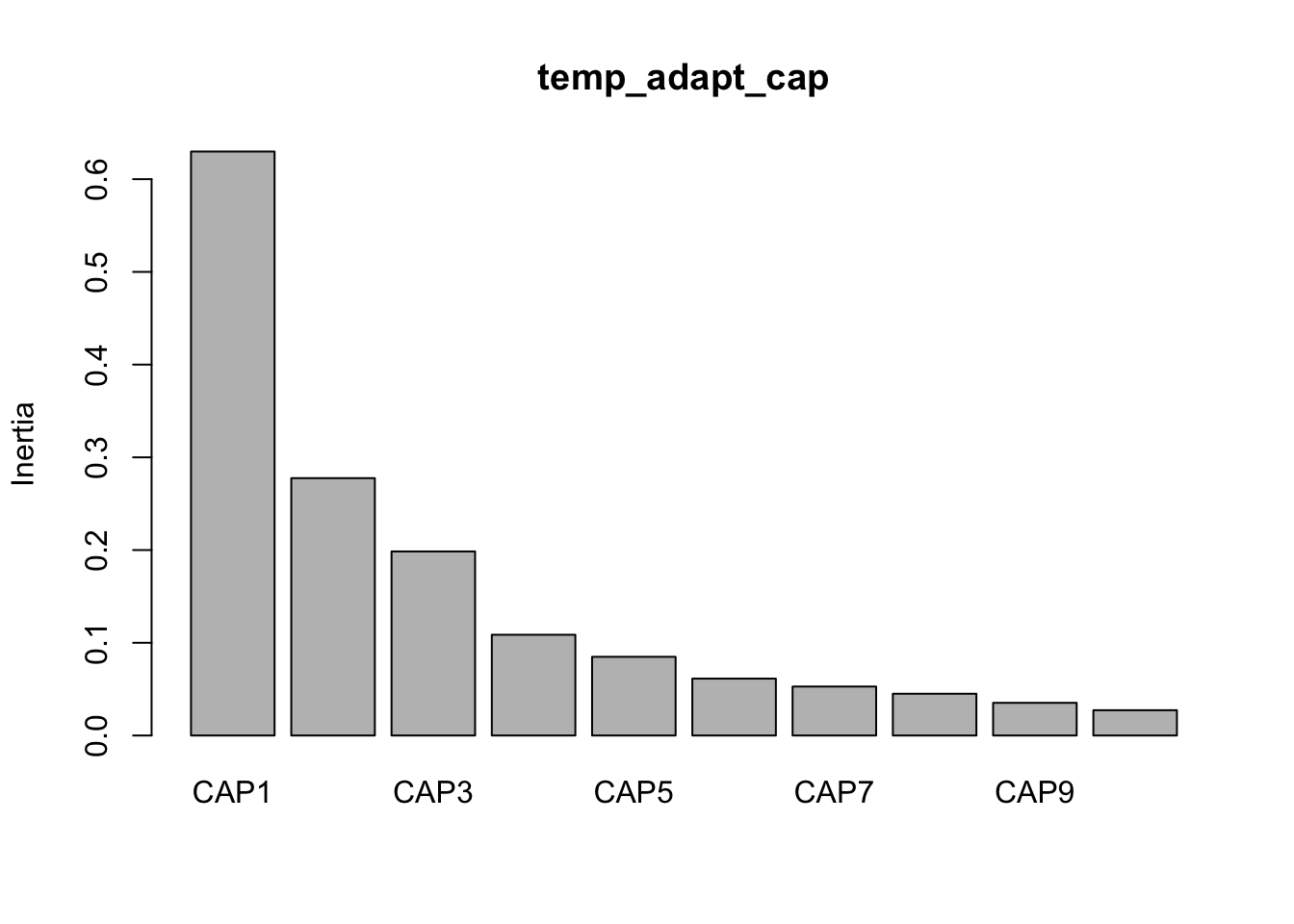And then make some quick and dirty plots. This will also come in handy later when we need to parse out data a better plot visualization. The `ggplot` function `autoplot` stores these data in a more accessible way than the raw results from `capscale`

``base::plot(temp_adapt_cap) ``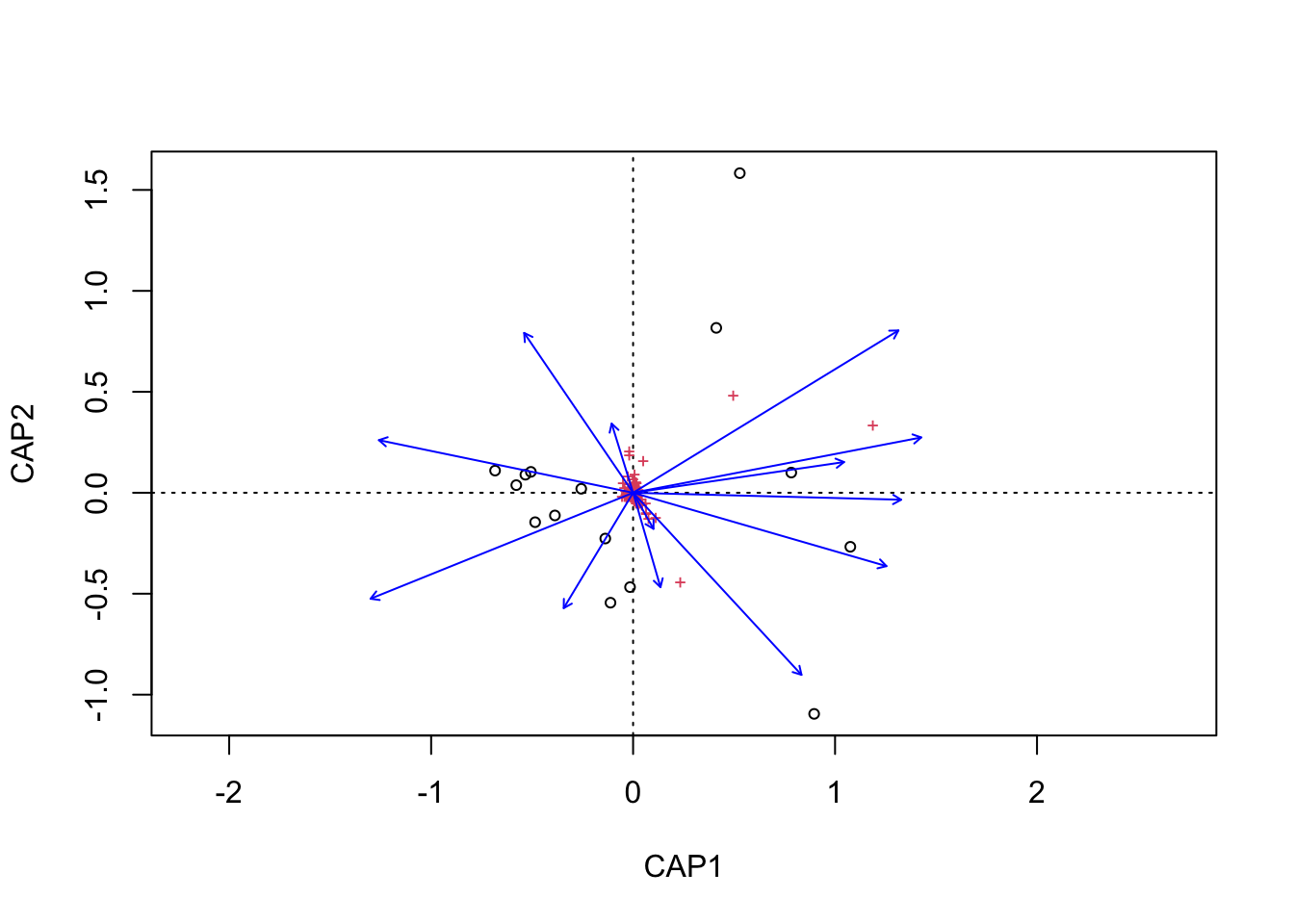``````tmp_auto_plt <- autoplot(temp_adapt_cap, arrows = TRUE)
tmp_auto_plt``````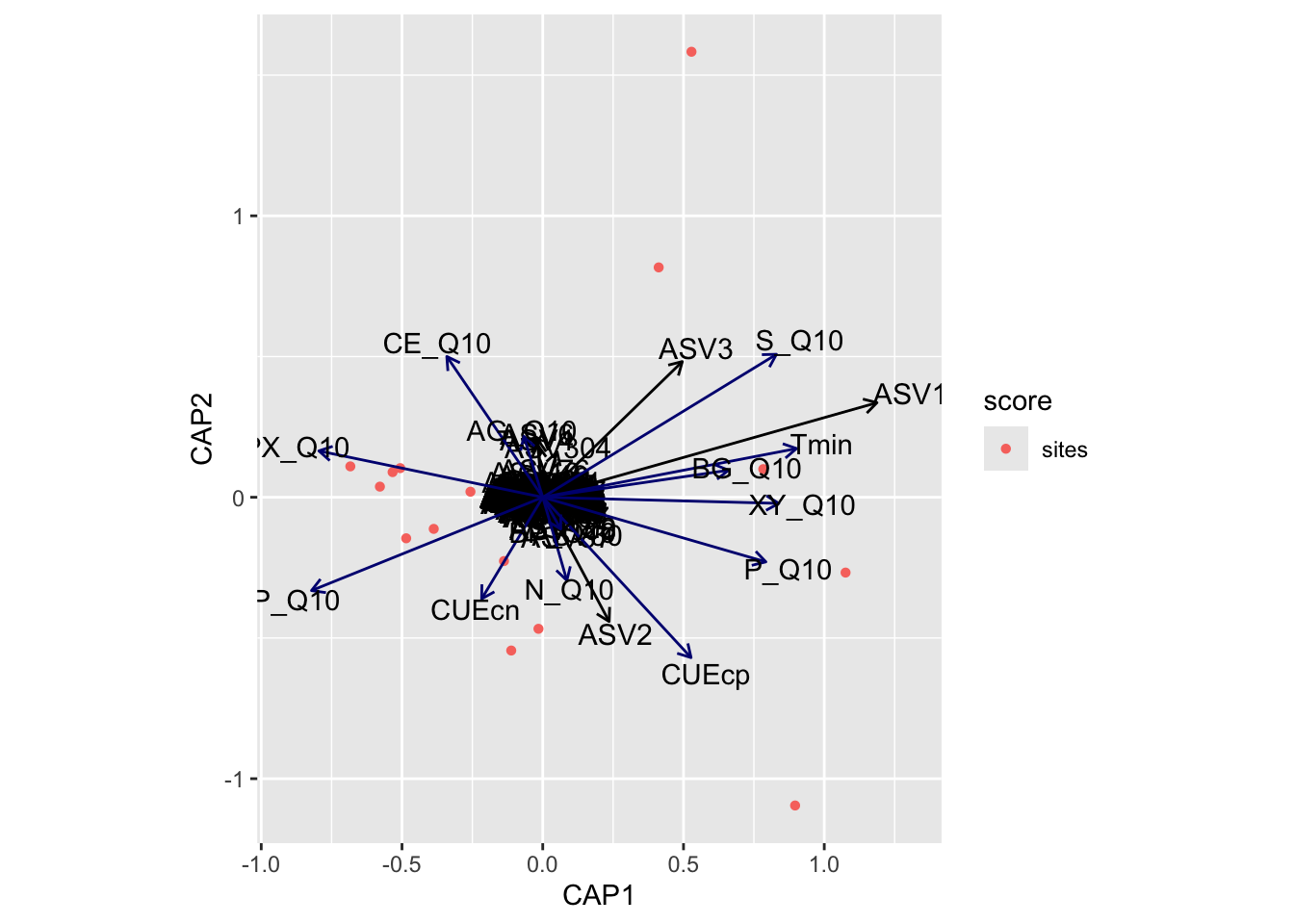Next, we need to grab capscale scores for the samples and create a data frame of the first two dimensions. We will also need to add some of the sample details to the data frame. For this we use the vegan function `scores` which gets species or site scores from the ordination.

Code
``````tmp_samp_scores <- dplyr::filter(tmp_auto_plt\$plot_env\$obj, Score == "sites")
tmp_samp_scores[,1] <- NULL
tmp_samp_scores <- tmp_samp_scores %>% dplyr::rename(SampleID = Label)

tmp_md_sub <- tmp_md[, 1:4]
tmp_md_sub <- tmp_md_sub %>% tibble::rownames_to_column("SampleID")
by = "SampleID")``````

Now we have a new data frame that contains sample details and capscale values.

``````          SampleID PLOT TREAT TREAT_T PAIR        CAP1        CAP2
1  P10_D00_010_C0E  P10     C       0    E -0.50625375  0.10348626
2  P02_D00_010_C0A  P02     C       0    A -0.48509579 -0.14580527
3  P04_D00_010_C0B  P04     C       0    B -0.57845671  0.03776429
4  P06_D00_010_C0C  P06     C       0    C -0.53336078  0.08936059
5  P08_D00_010_C0D  P08     C       0    D -0.68345909  0.10974979
6  P01_D00_010_W3A  P01     W       3    A -0.13776351 -0.22674265
7  P03_D00_010_W3B  P03     W       3    B -0.25679729  0.01972197
8  P05_D00_010_W3C  P05     W       3    C -0.38722889 -0.11236725
9  P07_D00_010_W3D  P07     W       3    D -0.01518359 -0.46741081
10 P09_D00_010_W3E  P09     W       3    E -0.11194937 -0.54460136
11 P01_D00_010_W8A  P01     W       8    A  0.78329851  0.09997647
12 P03_D00_010_W8B  P03     W       8    B  0.89642936 -1.09522700
13 P05_D00_010_W8C  P05     W       8    C  1.07566246 -0.26764258
14 P07_D00_010_W8D  P07     W       8    D  0.41185806  0.81673870
15 P09_D00_010_W8E  P09     W       8    E  0.52830039  1.58299886``````

We can then do the same with the metadata vectors. Here though we only need the scores and parameter name.

Code
``````temp_adapt_md_scores <- dplyr::filter(tmp_auto_plt\$plot_env\$obj, Score == "biplot")
dplyr::mutate(parameters = Label, .before = CAP1) %>%
tibble::column_to_rownames("Label")``````
``````       parameters        CAP1        CAP2
AG_Q10     AG_Q10 -0.07373951  0.23793282
BG_Q10     BG_Q10  0.72604945  0.10579764
BP_Q10     BP_Q10  0.07015779 -0.12413231
CE_Q10     CE_Q10 -0.37489864  0.55022094
P_Q10       P_Q10  0.87190011 -0.25252818
N_Q10       N_Q10  0.09422795 -0.32515404
S_Q10       S_Q10  0.91309230  0.55878516
XY_Q10     XY_Q10  0.92245895 -0.02395029
LP_Q10     LP_Q10 -0.90356227 -0.36479560
PX_Q10     PX_Q10 -0.87475774  0.18121787
CUEcn       CUEcn -0.23872665 -0.39707742
CUEcp       CUEcp  0.57949983 -0.62675335
Tmin         Tmin  0.99158847  0.19050834``````

Let’s run some quick correlations of metadata with ordination axes to see which parameters are significant. For this we use the vegan function `envfit`.

Code
``````tmp_samp_scores_sub <- temp_adapt_plot_data[, 6:7]
tmp_samp_scores_sub <- as.matrix(tmp_samp_scores_sub)

tmp_md_sub <- subset(tmp_md,  select =  tmp_param_list)

perm = 1000, choices = c(1, 2))``````
``````
***VECTORS

CAP1     CAP2     r2  Pr(>r)
AG_Q10 -0.30810  0.95136 0.0260 0.83117
BG_Q10  0.99031  0.13889 0.2354 0.17782
BP_Q10  0.50944 -0.86050 0.0086 0.95504
CE_Q10 -0.58116  0.81379 0.1875 0.25075
P_Q10   0.96427 -0.26494 0.3590 0.05395 .
N_Q10   0.28973 -0.95711 0.0479 0.76723
S_Q10   0.86347  0.50440 0.4956 0.01499 *
XY_Q10  0.99973 -0.02334 0.3725 0.04895 *
LP_Q10 -0.93292 -0.36008 0.4131 0.04096 *
PX_Q10 -0.98131  0.19244 0.3483 0.07992 .
CUEcn  -0.53407 -0.84544 0.0908 0.55245
CUEcp   0.69625 -0.71780 0.3103 0.12288
Tmin    0.98341  0.18140 0.4456 0.02997 *
---
Signif. codes:  0 '***' 0.001 '**' 0.01 '*' 0.05 '.' 0.1 ' ' 1
Permutation: free
Number of permutations: 1000``````
Code
``````temp_adapt_md_signif_hits <- base::subset(envfit_temp_adapt_md\$vectors\$pvals,
< 0.05 & envfit_temp_adapt_md\$vectors\$r > 0.4))

`envfit` found that S_Q10, LP_Q10, Tmin were significantly correlated.

Now let’s see if the same parameters are significant for the `envfit` and `bioenv` analyses.

`````` "Significant parameters from bioenv analysis."
 "CUEcp"  "LP_Q10" "P_Q10"  "S_Q10"  "Tmin"
_____________________________________
 "Significant parameters from envfit analysis."
 "S_Q10"  "LP_Q10" "Tmin"
_____________________________________
 "Found in bioenv but not envfit."
 "CUEcp" "P_Q10"
_____________________________________
 "Found in envfit but not bioenv."
character(0)
_____________________________________
 "Found in envfit and  bioenv."
 "S_Q10"  "LP_Q10" "Tmin"   "CUEcp"  "P_Q10" ``````
Code
``````#new_temp_adapt_md_signif_hits <- base::append(temp_adapt_md_signif_hits, temp_adapt_sig_diff)

Check. Next, we run `envfit` for the ASVs.

Code
``````envfit_temp_adapt_asv <- envfit(tmp_samp_scores_sub,
tmp_comm[, order(colSums(-tmp_comm))][, 1:10],
perm = 1000, choices = c(1, 2))

temp_adapt_asv_scores <- dplyr::filter(tmp_auto_plt\$plot_env\$obj, Score == "species")

dplyr::mutate(parameters = Label, .before = CAP1) %>%
tibble::column_to_rownames("Label")
``````
***VECTORS

CAP1     CAP2     r2   Pr(>r)
ASV1   0.98984  0.14217 0.6541 0.009990 **
ASV2   0.55070 -0.83470 0.8953 0.000999 ***
ASV3   0.79919  0.60108 0.9513 0.000999 ***
ASV4  -0.12171  0.99257 0.3110 0.118881
ASV5  -0.67644 -0.73649 0.1181 0.487512
ASV6  -0.10078  0.99491 0.7028 0.003996 **
ASV8  -0.85434  0.51972 0.4279 0.043956 *
ASV7  -0.57853 -0.81566 0.0602 0.676324
ASV9   0.53361 -0.84573 0.2425 0.185814
ASV10 -0.34761  0.93764 0.8031 0.000999 ***
---
Signif. codes:  0 '***' 0.001 '**' 0.01 '*' 0.05 '.' 0.1 ' ' 1
Permutation: free
Number of permutations: 1000``````
Code
``````temp_adapt_asv_signif_hits <- base::subset(envfit_temp_adapt_asv\$vectors\$pvals,
< 0.05 & envfit_temp_adapt_asv\$vectors\$r > 0.5))
``````      parameters        CAP1        CAP2 variable_type
ASV3        ASV3  0.49583686  0.48216614           ASV
ASV6        ASV6 -0.01759546  0.20461984           ASV
ASV2        ASV2  0.23578207 -0.44144325           ASV
ASV10      ASV10 -0.02475900  0.08142465           ASV
ASV1        ASV1  1.18724098  0.33621587           ASV``````
Code
``````temp_adapt_md_signif_all\$variable_type <- "metadata"

The last thing to do is categorize parameters scores and ASV scores into different variable types for plotting.

Code
``````temp_adapt_bioplot_data_md <- subset(temp_adapt_bioplot_data,
Show the code for the plot
``````temp_adapt_cap_vals <- data.frame(temp_adapt_cap\$CCA\$eig[1:2])

cpa1_lab <- paste("CAP1", " (", temp_adapt_cap1, "%)", sep = "")
cpa2_lab <- paste("CAP2", " (", temp_adapt_cap2, "%)", sep = "")

swel_col <- c("#2271B2", "#71B222", "#B22271")

geom_point(mapping = aes(x = CAP1, y = CAP2, shape = TREAT,
colour = TREAT_T), size = 4) +
scale_colour_manual(values = swel_col) +
#  geom_text(data = temp_adapt_plot_data, aes(x = CAP1, y = CAP2, #UNCOMMENT to add sample labels
#                              label = SampleID), size = 3) +
geom_segment(aes(x = 0, y = 0, xend = CAP1, yend = CAP2),
data = temp_adapt_bioplot_data_md, linetype = "solid",
arrow = arrow(length = unit(0.3, "cm")), size = 0.4,
color = "#191919", inherit.aes = FALSE) +
aes(x = CAP1, y = CAP2, label = parameters), size = 3,
nudge_x = 0.1, nudge_y = 0.05) +
#  geom_segment(aes(x = 0, y = 0, xend = CAP1, yend = CAP2),
#               data = temp_adapt_bioplot_data_asv, linetype = "solid",
#               arrow = arrow(length = unit(0.3, "cm")), size = 0.2,
#               color = "#676767") +
#            aes(x = CAP1, y = CAP2, label = parameters), size = 2.5,
#            nudge_x = 0.05, nudge_y = 0.05) +
theme_classic(base_size = 12) +
labs(title = "Capscale Analysis",
x = cpa1_lab,
y = cpa2_lab)
height = 16, width = 20, units = 'cm', res = 600, bg = "white")
invisible(dev.off())
height = 5, width = 6)
dev.off()``````

## Capscale Plots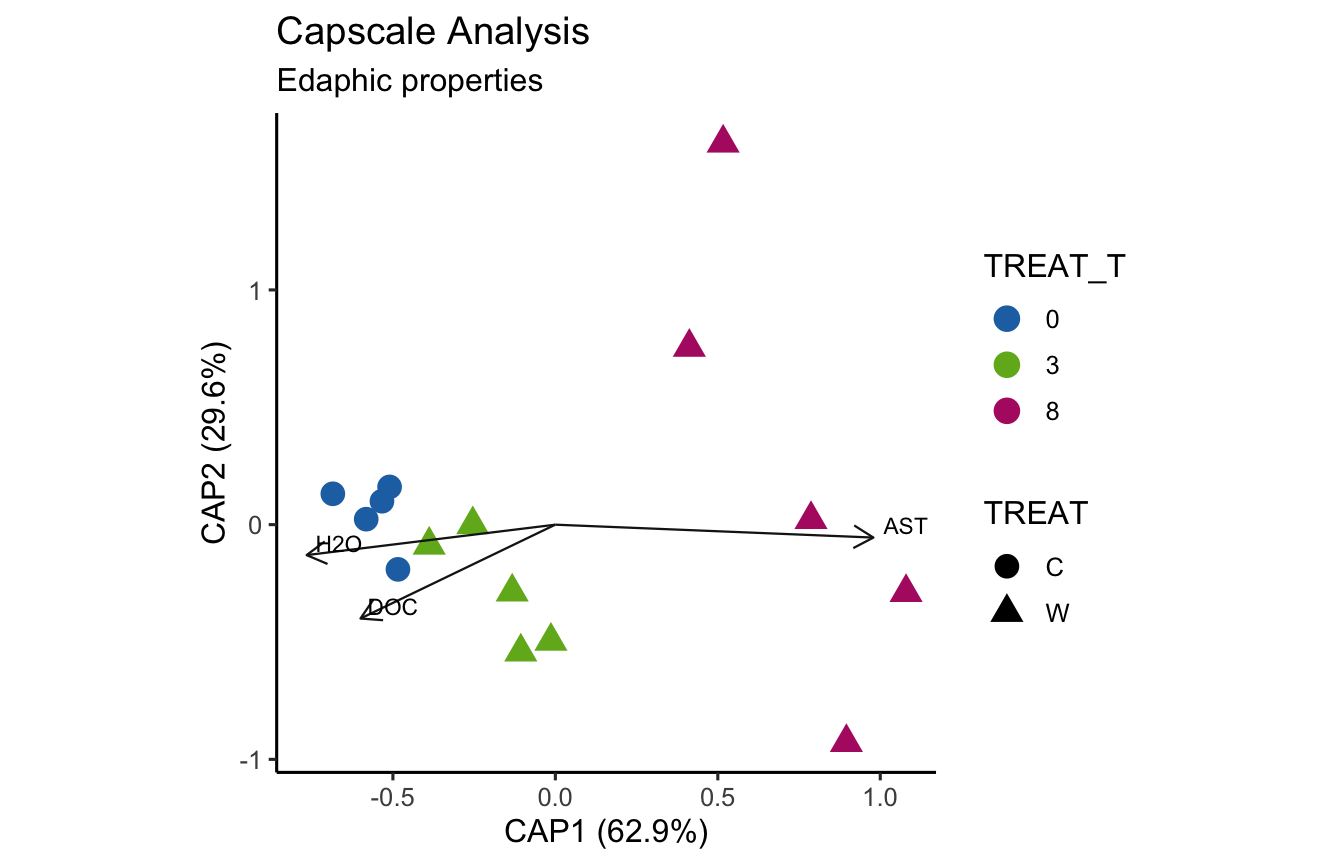(16S rRNA) Figure 7 | Distance-based Redundancy Analysis (db-RDA) of PIME filtered data based on Bray-Curtis dissimilarity showing the relationships between community composition change versus edaphic properties.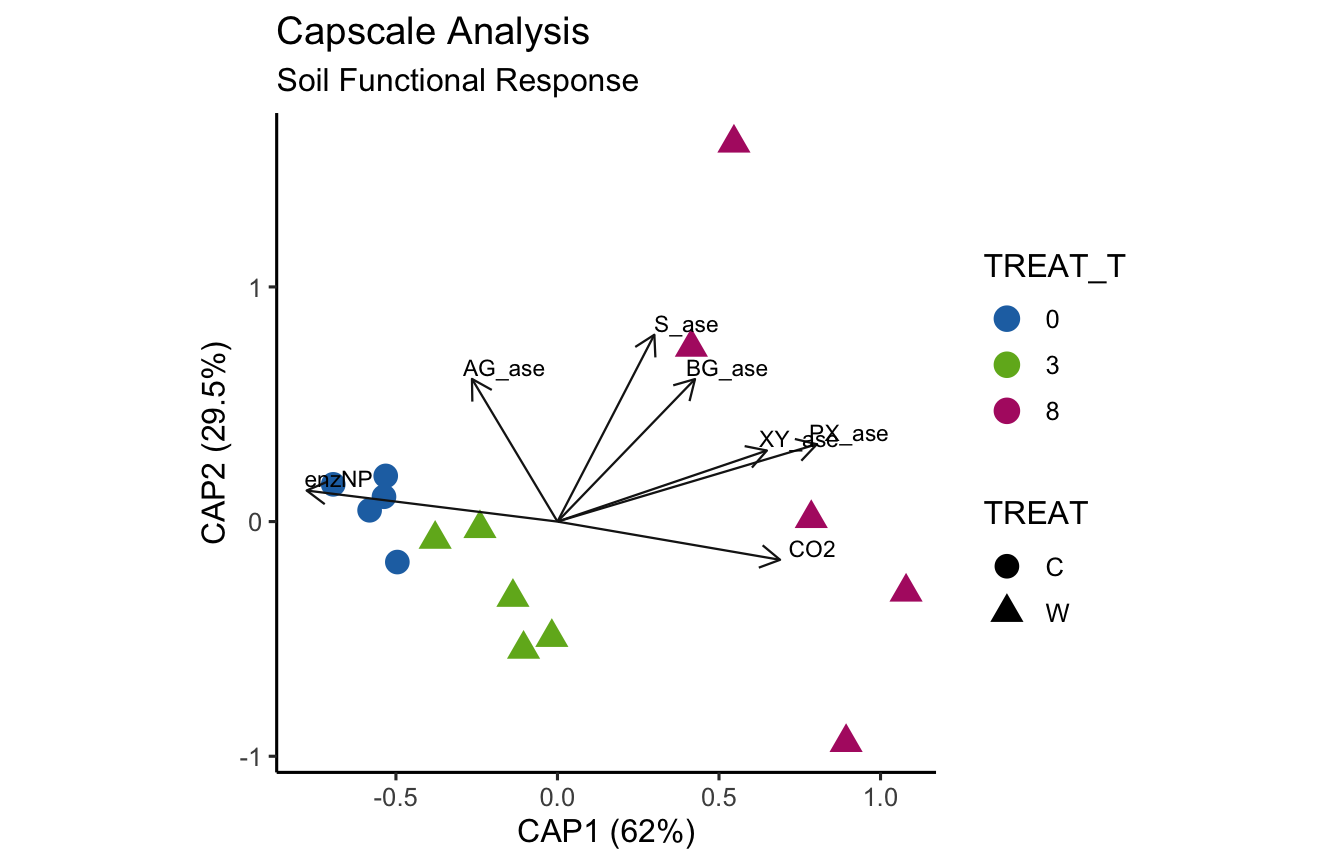(16S rRNA) Figure 8 | Distance-based Redundancy Analysis (db-RDA) of PIME filtered data based on Bray-Curtis dissimilarity showing the relationships between community composition change versus microbial functional response.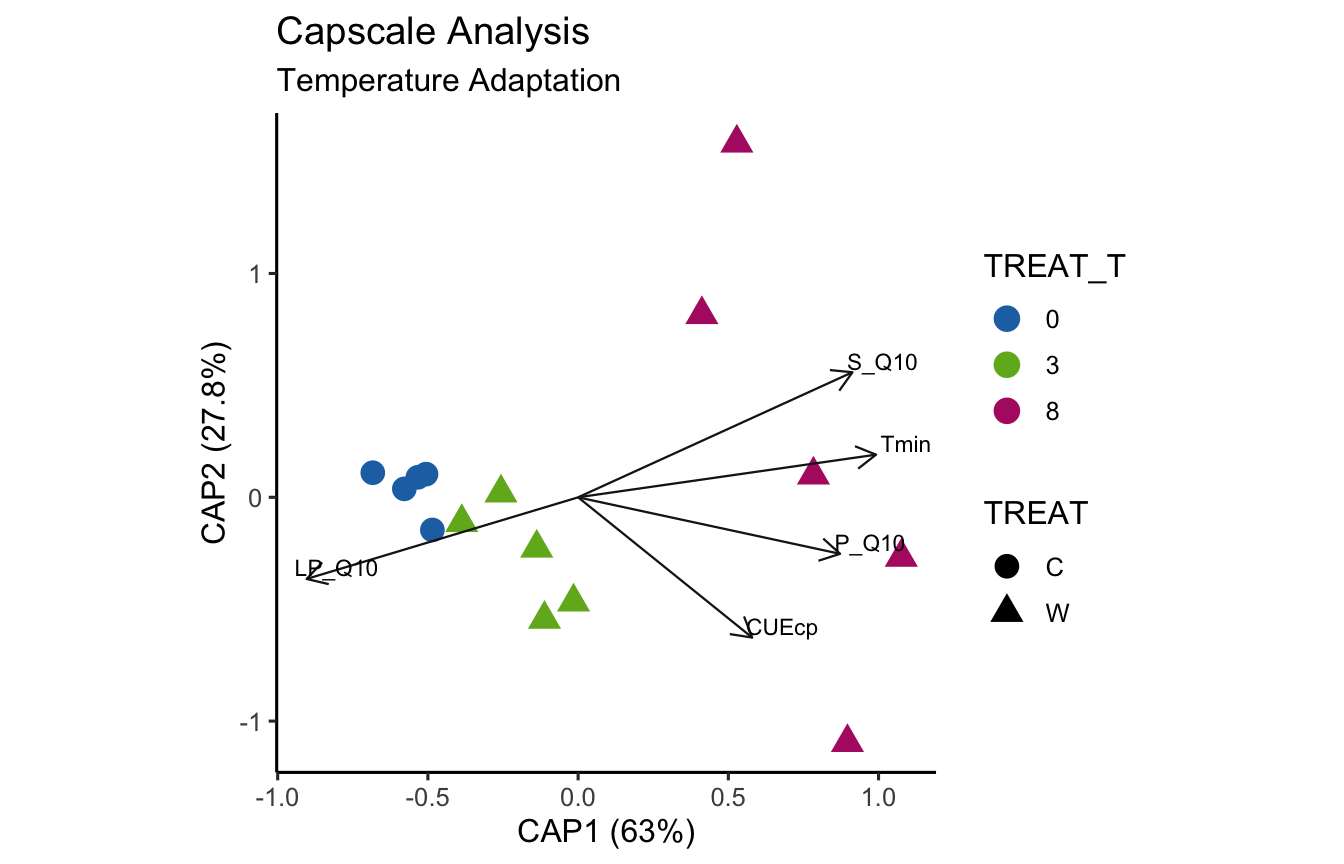(16S rRNA) Figure 9 | Distance-based Redundancy Analysis (db-RDA) of PIME filtered data based on Bray-Curtis dissimilarity showing the relationships between community composition change versus temperature adaptation.

# ITS

## Data Formating

1. Transform `ps` Objects

The first step is to transform the phyloseq objects.

Code
``````samp_ps <- c("its18_ps_work", "its18_ps_pime", "its18_ps_perfect",
"its18_ps_work_otu", "its18_ps_pime_otu", "its18_ps_perfect_otu")
for (i in samp_ps) {
tmp_get <- get(i)
tmp_ps <- transform_sample_counts(tmp_get, function(otu) 1e5 * otu/sum(otu))
tmp_ps@phy_tree <- NULL
tmp_ps <- prune_samples(sample_sums(tmp_ps) > 0, tmp_ps)
tmp_samp <- data.frame(sample_data(tmp_get))
tmp_samp <- tmp_samp %>% dplyr::rename("TREAT_T" = "TEMP")
tmp_ps <- merge_phyloseq(tmp_ps, sample_data)
sample_data(tmp_ps) <- tmp_samp
tmp_name <- purrr::map_chr(i, ~ paste0(., "_prop"))
print(tmp_name)
assign(tmp_name, tmp_ps)
rm(list = ls(pattern = "tmp_"))
}``````
1. Format for `mctoolsr`

Then a bit of formatting to make the data compatible with `mctoolsr`.

Code
``````for (i in samp_ps) {
tmp_get <- get(purrr::map_chr(i, ~ paste0(., "_prop")))
tmp_tax <- data.frame(tax_table(tmp_get))
tmp_tax\$ASV_SEQ <- NULL

tmp_col_names <- colnames(tmp_tax)
tmp_tax_merge <- tmp_tax %>% tidyr::unite(taxonomy,
all_of(tmp_col_names),
sep = ";")
tmp_tax_merge <- tmp_tax_merge %>% tibble::rownames_to_column("#OTU_ID")
tmp_otu <- data.frame(t(otu_table(tmp_get)))
tmp_otu <- tmp_otu %>% tibble::rownames_to_column("#OTU_ID")
tmp_otu_tax <- dplyr::left_join(tmp_otu, tmp_tax_merge, by = "#OTU_ID")

tmp_samp <- data.frame(sample_data(tmp_get))
tmp_samp[,c(1,3)] <- NULL
tmp_samp <- tmp_samp %>% tibble::rownames_to_column("#SampleID")

tmp_md <- dplyr::left_join(tmp_samp, tmp_metad, by = "#SampleID")

tmp_otu_name <- purrr::map_chr(i, ~ paste0(., "_otu_tax"))
print(tmp_otu_name)
assign(tmp_otu_name, tmp_otu_tax)

tmp_md_name <- purrr::map_chr(i, ~ paste0(., "_md"))
print(tmp_md_name)
assign(tmp_md_name, tmp_md)

write_delim(tmp_otu_tax, paste(tmp_path, tmp_otu_name, ".txt", sep = ""), delim = "\t")
write_delim(tmp_md, paste(tmp_path, tmp_md_name, ".txt", sep = ""), delim = "\t")
rm(list = ls(pattern = "tmp_"))
}``````
Code
``````for (i in samp_ps) {
tmp_otu_name <- purrr::map_chr(i, ~ paste0(., "_otu_tax"))
tmp_md_name <- purrr::map_chr(i, ~ paste0(., "_md"))
tmp_tax_table_fp <- paste(tmp_path, tmp_otu_name, ".txt", sep = "")
tmp_map_fp <- paste(tmp_path, tmp_md_name, ".txt", sep = "")
tmp_input_name <- purrr::map_chr(i, ~ paste0(., "_mc"))
print(tmp_input_name)
assign(tmp_input_name, tmp_input)
rm(list = ls(pattern = "tmp_"))
}
rm(list = ls(pattern = "_md"))
rm(list = ls(pattern = "_otu_tax"))``````

## Choose Data Set

At this point in the code we need to choose a data set to use, formatted with `mctoolsr`. Remember, there are four choices:

1. Complete ASV data set.
2. Arbitrary filtered ASV data set.
3. PERfect filtered ASV data set.
4. PIME filtered ASV data set.

This way, if we want to test other data sets we only need to change the name here.

``````objects(pattern = "_mc")
its18_select_mc <- its18_ps_pime_mc``````

## Normality Tests

Before proceeding, we need to test each parameter in the metadata to see which ones are and are not normally distributed. For that, we use the Shapiro-Wilk Normality Test. Here we only need one of the metadata files.

Code
``````temp_md <- its18_select_mc\$map_loaded
temp_md[,1:9] <- NULL
shap_results <- NULL

for (i in colnames(temp_md)) {
tmp_shap <- shapiro.test(temp_md[[i]])
tmp_p <- round(tmp_shap\$p.value, digits = 5)
tmp_res <- eval(isTRUE(tmp_shap\$p.value < 0.05))
shap_results <- rbind(shap_results, data.frame(i, tmp_p, tmp_res))
rm(list = ls(pattern = "tmp_"))
}
colnames(shap_results) <- c("parameter", "p-value", "tranform")
shap_results
dplyr::filter(shap_results, tranform == "TRUE")
md_to_tranform <- shap_results\$parameter[shap_results\$tranform == TRUE]
rm(list = ls(pattern = "temp_md"))``````
Show the results of each normality test for metadata parameters

(ITS) Table 2 | Results of the Shapiro-Wilk Normality Tests. P-values in red are significance (p-value < 0.05) meaning the parameter needs to be normalized.

Looks like we need to transform 21 metadata parameters.

## Normalize Parameters

Here we use the R package `bestNormalize` to find and execute the best normalizing transformation. The function will test the following normalizing transformations:

• `arcsinh_x` performs an arcsinh transformation.

• `boxcox` Perform a Box-Cox transformation and center/scale a vector to attempt normalization. `boxcox` estimates the optimal value of lambda for the Box-Cox transformation. The function will return an error if a user attempt to transform nonpositive data.

• `yeojohnson` Perform a Yeo-Johnson Transformation and center/scale a vector to attempt normalization. `yeojohnson` estimates the optimal value of lambda for the Yeo-Johnson transformation. The Yeo-Johnson is similar to the Box-Cox method, however it allows for the transformation of nonpositive data as well.

• `orderNorm` The Ordered Quantile (ORQ) normalization transformation, `orderNorm()`, is a rank-based procedure by which the values of a vector are mapped to their percentile, which is then mapped to the same percentile of the normal distribution. Without the presence of ties, this essentially guarantees that the transformation leads to a uniform distribution.

• `log_x` performs a simple log transformation. The parameter a is essentially estimated by the training set by default (estimated as the minimum possible to some extent epsilon), while the base must be specified beforehand. The default base of the log is 10.

• `sqrt_x` performs a simple square-root transformation. The parameter a is essentially estimated by the training set by default (estimated as the minimum possible), while the base must be specified beforehand.

• `exp_x` performs a simple exponential transformation.

See this GitHub issue (#5) for a description on getting reproducible results. Apparently, you can get different results because the `bestNormalize()` function uses repeated cross-validation (and doesn’t automatically set the seed), so the results will be slightly different each time the function is executed.

Code
``````set.seed(119)

for (i in md_to_tranform) {
tmp_best_norm <- bestNormalize(tmp_md[[i]], r = 1, k = 5, loo = TRUE)
tmp_name <- purrr::map_chr(i, ~ paste0(., "_best_norm_test"))
assign(tmp_name, tmp_best_norm)
print(tmp_name)
rm(list = ls(pattern = "tmp_"))
}``````
Show the chosen transformations
``````
## bestNormalize Chosen transformation of AST ##
orderNorm Transformation with 13 nonmissing obs and no ties
- Original quantiles:
0%   25%   50%   75%  100%
25.80 26.26 28.80 29.90 41.77
_____________________________________

## bestNormalize Chosen transformation of H2O ##
orderNorm Transformation with 13 nonmissing obs and no ties
- Original quantiles:
0%   25%   50%   75%  100%
0.204 0.261 0.377 0.385 0.401
_____________________________________

## bestNormalize Chosen transformation of Al ##
Standardized asinh(x) Transformation with 13 nonmissing obs.:
Relevant statistics:
- mean (before standardization) = 0.005384372
- sd (before standardization) = 0.007762057
_____________________________________

## bestNormalize Chosen transformation of Fe ##
Standardized asinh(x) Transformation with 13 nonmissing obs.:
Relevant statistics:
- mean (before standardization) = 0.01076821
- sd (before standardization) = 0.0103749
_____________________________________

## bestNormalize Chosen transformation of TEB ##
orderNorm Transformation with 13 nonmissing obs and ties
- 10 unique values
- Original quantiles:
0%  25%  50%  75% 100%
41.6 43.3 45.1 48.7 61.7
_____________________________________

## bestNormalize Chosen transformation of ECEC ##
orderNorm Transformation with 13 nonmissing obs and ties
- 10 unique values
- Original quantiles:
0%  25%  50%  75% 100%
41.7 43.3 45.3 48.8 61.8
_____________________________________

## bestNormalize Chosen transformation of minNO3 ##
orderNorm Transformation with 13 nonmissing obs and no ties
- Original quantiles:
0%    25%    50%    75%   100%
11.31  19.67  22.71  37.33 106.42
_____________________________________

## bestNormalize Chosen transformation of minTIN ##
orderNorm Transformation with 13 nonmissing obs and ties
- 12 unique values
- Original quantiles:
0%    25%    50%    75%   100%
12.96  21.50  23.99  42.08 110.75
_____________________________________

## bestNormalize Chosen transformation of DOC ##
orderNorm Transformation with 13 nonmissing obs and no ties
- Original quantiles:
0%    25%    50%    75%   100%
33.20  44.30  56.68  63.79 118.74
_____________________________________

## bestNormalize Chosen transformation of BG_ase ##
orderNorm Transformation with 13 nonmissing obs and no ties
- Original quantiles:
0%  25%  50%  75% 100%
3.04 3.92 4.22 4.62 9.09
_____________________________________

## bestNormalize Chosen transformation of BP_ase ##
orderNorm Transformation with 13 nonmissing obs and no ties
- Original quantiles:
0%  25%  50%  75% 100%
1.77 2.33 3.02 3.50 7.28
_____________________________________

## bestNormalize Chosen transformation of P_ase ##
Standardized Box Cox Transformation with 13 nonmissing obs.:
Estimated statistics:
- lambda = -0.9999576
- mean (before standardization) = 0.9480149
- sd (before standardization) = 0.01593697
_____________________________________

## bestNormalize Chosen transformation of N_ase ##
orderNorm Transformation with 13 nonmissing obs and no ties
- Original quantiles:
0%  25%  50%  75% 100%
2.57 2.95 3.58 4.52 8.34
_____________________________________

## bestNormalize Chosen transformation of XY_ase ##
orderNorm Transformation with 13 nonmissing obs and ties
- 11 unique values
- Original quantiles:
0%  25%  50%  75% 100%
0.66 0.76 1.11 1.36 2.52
_____________________________________

## bestNormalize Chosen transformation of BG_Q10 ##
orderNorm Transformation with 13 nonmissing obs and ties
- 11 unique values
- Original quantiles:
0%  25%  50%  75% 100%
1.31 1.36 1.39 1.56 1.76
_____________________________________

## bestNormalize Chosen transformation of CE_Q10 ##
orderNorm Transformation with 13 nonmissing obs and ties
- 12 unique values
- Original quantiles:
0%  25%  50%  75% 100%
1.76 1.87 1.95 2.00 2.36
_____________________________________

## bestNormalize Chosen transformation of CO2 ##
Standardized asinh(x) Transformation with 13 nonmissing obs.:
Relevant statistics:
- mean (before standardization) = 2.626573
- sd (before standardization) = 0.7396036
_____________________________________

## bestNormalize Chosen transformation of PX_ase ##
orderNorm Transformation with 13 nonmissing obs and no ties
- Original quantiles:
0%    25%    50%    75%   100%
81.25  94.13 119.75 211.18 294.39
_____________________________________

## bestNormalize Chosen transformation of PX_Q10 ##
Standardized Yeo-Johnson Transformation with 13 nonmissing obs.:
Estimated statistics:
- lambda = -4.999963
- mean (before standardization) = 0.1968145
- sd (before standardization) = 0.001394949
_____________________________________

## bestNormalize Chosen transformation of CUEcp ##
orderNorm Transformation with 13 nonmissing obs and ties
- 9 unique values
- Original quantiles:
0%  25%  50%  75% 100%
0.15 0.17 0.19 0.21 0.33
_____________________________________

## bestNormalize Chosen transformation of PUE ##
orderNorm Transformation with 13 nonmissing obs and ties
- 6 unique values
- Original quantiles:
0%  25%  50%  75% 100%
0.77 0.88 0.90 0.91 0.92
_____________________________________``````
Show the complete `bestNormalize` results
``````
## Results of bestNormalize for AST ##
Best Normalizing transformation with 13 Observations
Estimated Normality Statistics (Pearson P / df, lower => more normal):
- arcsinh(x): 3.2051
- Box-Cox: 3.2051
- Center+scale: 4.1282
- Exp(x): 17.9744
- Log_b(x+a): 3.2051
- orderNorm (ORQ): 0.1282
- sqrt(x + a): 4.1282
- Yeo-Johnson: 2.2821
Estimation method: Out-of-sample via leave-one-out CV

Based off these, bestNormalize chose:
orderNorm Transformation with 13 nonmissing obs and no ties
- Original quantiles:
0%   25%   50%   75%  100%
25.80 26.26 28.80 29.90 41.77
_____________________________________

## Results of bestNormalize for H2O ##
Best Normalizing transformation with 13 Observations
Estimated Normality Statistics (Pearson P / df, lower => more normal):
- arcsinh(x): 7.2051
- Box-Cox: 6.2821
- Center+scale: 8.1282
- Exp(x): 6.2821
- Log_b(x+a): 7.2051
- orderNorm (ORQ): 0.4359
- sqrt(x + a): 7.2051
- Yeo-Johnson: 6.2821
Estimation method: Out-of-sample via leave-one-out CV

Based off these, bestNormalize chose:
orderNorm Transformation with 13 nonmissing obs and no ties
- Original quantiles:
0%   25%   50%   75%  100%
0.204 0.261 0.377 0.385 0.401
_____________________________________

## Results of bestNormalize for Al ##
Best Normalizing transformation with 13 Observations
Estimated Normality Statistics (Pearson P / df, lower => more normal):
- arcsinh(x): 7.5128
- Center+scale: 7.5128
- Exp(x): 7.5128
- Log_b(x+a): 9.359
- orderNorm (ORQ): 7.5128
- sqrt(x + a): 7.5128
- Yeo-Johnson: 7.5128
Estimation method: Out-of-sample via leave-one-out CV

Based off these, bestNormalize chose:
Standardized asinh(x) Transformation with 13 nonmissing obs.:
Relevant statistics:
- mean (before standardization) = 0.005384372
- sd (before standardization) = 0.007762057
_____________________________________

## Results of bestNormalize for Fe ##
Best Normalizing transformation with 13 Observations
Estimated Normality Statistics (Pearson P / df, lower => more normal):
- arcsinh(x): 7.2051
- Center+scale: 7.2051
- Exp(x): 7.2051
- Log_b(x+a): 7.2051
- orderNorm (ORQ): 7.2051
- sqrt(x + a): 7.2051
- Yeo-Johnson: 7.2051
Estimation method: Out-of-sample via leave-one-out CV

Based off these, bestNormalize chose:
Standardized asinh(x) Transformation with 13 nonmissing obs.:
Relevant statistics:
- mean (before standardization) = 0.01076821
- sd (before standardization) = 0.0103749
_____________________________________

## Results of bestNormalize for TEB ##
Best Normalizing transformation with 13 Observations
Estimated Normality Statistics (Pearson P / df, lower => more normal):
- arcsinh(x): 1.6667
- Box-Cox: 1.6667
- Center+scale: 4.7436
- Exp(x): 17.9744
- Log_b(x+a): 1.6667
- orderNorm (ORQ): 0.4359
- sqrt(x + a): 3.2051
- Yeo-Johnson: 1.359
Estimation method: Out-of-sample via leave-one-out CV

Based off these, bestNormalize chose:
orderNorm Transformation with 13 nonmissing obs and ties
- 10 unique values
- Original quantiles:
0%  25%  50%  75% 100%
41.6 43.3 45.1 48.7 61.7
_____________________________________

## Results of bestNormalize for ECEC ##
Best Normalizing transformation with 13 Observations
Estimated Normality Statistics (Pearson P / df, lower => more normal):
- arcsinh(x): 1.6667
- Box-Cox: 1.6667
- Center+scale: 3.2051
- Exp(x): 17.9744
- Log_b(x+a): 1.6667
- orderNorm (ORQ): 0.4359
- sqrt(x + a): 3.2051
- Yeo-Johnson: 1.359
Estimation method: Out-of-sample via leave-one-out CV

Based off these, bestNormalize chose:
orderNorm Transformation with 13 nonmissing obs and ties
- 10 unique values
- Original quantiles:
0%  25%  50%  75% 100%
41.7 43.3 45.3 48.8 61.8
_____________________________________

## Results of bestNormalize for minNO3 ##
Best Normalizing transformation with 13 Observations
Estimated Normality Statistics (Pearson P / df, lower => more normal):
- arcsinh(x): 3.5128
- Box-Cox: 3.5128
- Center+scale: 5.359
- Exp(x): 17.9744
- Log_b(x+a): 3.5128
- orderNorm (ORQ): 0.7436
- sqrt(x + a): 2.5897
- Yeo-Johnson: 3.5128
Estimation method: Out-of-sample via leave-one-out CV

Based off these, bestNormalize chose:
orderNorm Transformation with 13 nonmissing obs and no ties
- Original quantiles:
0%    25%    50%    75%   100%
11.31  19.67  22.71  37.33 106.42
_____________________________________

## Results of bestNormalize for minTIN ##
Best Normalizing transformation with 13 Observations
Estimated Normality Statistics (Pearson P / df, lower => more normal):
- arcsinh(x): 1.359
- Box-Cox: 2.2821
- Center+scale: 4.1282
- Exp(x): 17.9744
- Log_b(x+a): 1.359
- orderNorm (ORQ): 0.7436
- sqrt(x + a): 2.2821
- Yeo-Johnson: 2.2821
Estimation method: Out-of-sample via leave-one-out CV

Based off these, bestNormalize chose:
orderNorm Transformation with 13 nonmissing obs and ties
- 12 unique values
- Original quantiles:
0%    25%    50%    75%   100%
12.96  21.50  23.99  42.08 110.75
_____________________________________

## Results of bestNormalize for DOC ##
Best Normalizing transformation with 13 Observations
Estimated Normality Statistics (Pearson P / df, lower => more normal):
- arcsinh(x): 2.8974
- Box-Cox: 2.8974
- Center+scale: 3.5128
- Exp(x): 17.9744
- Log_b(x+a): 2.8974
- orderNorm (ORQ): 0.4359
- sqrt(x + a): 1.9744
- Yeo-Johnson: 2.8974
Estimation method: Out-of-sample via leave-one-out CV

Based off these, bestNormalize chose:
orderNorm Transformation with 13 nonmissing obs and no ties
- Original quantiles:
0%    25%    50%    75%   100%
33.20  44.30  56.68  63.79 118.74
_____________________________________

## Results of bestNormalize for BG_ase ##
Best Normalizing transformation with 13 Observations
Estimated Normality Statistics (Pearson P / df, lower => more normal):
- arcsinh(x): 3.8205
- Box-Cox: 2.2821
- Center+scale: 4.1282
- Exp(x): 14.8974
- Log_b(x+a): 3.8205
- orderNorm (ORQ): 0.4359
- sqrt(x + a): 3.5128
- Yeo-Johnson: 1.6667
Estimation method: Out-of-sample via leave-one-out CV

Based off these, bestNormalize chose:
orderNorm Transformation with 13 nonmissing obs and no ties
- Original quantiles:
0%  25%  50%  75% 100%
3.04 3.92 4.22 4.62 9.09
_____________________________________

## Results of bestNormalize for BP_ase ##
Best Normalizing transformation with 13 Observations
Estimated Normality Statistics (Pearson P / df, lower => more normal):
- arcsinh(x): 1.6667
- Box-Cox: 1.6667
- Center+scale: 4.1282
- Exp(x): 5.6667
- Log_b(x+a): 1.6667
- orderNorm (ORQ): 0.4359
- sqrt(x + a): 1.6667
- Yeo-Johnson: 1.6667
Estimation method: Out-of-sample via leave-one-out CV

Based off these, bestNormalize chose:
orderNorm Transformation with 13 nonmissing obs and no ties
- Original quantiles:
0%  25%  50%  75% 100%
1.77 2.33 3.02 3.50 7.28
_____________________________________

## Results of bestNormalize for P_ase ##
Best Normalizing transformation with 13 Observations
Estimated Normality Statistics (Pearson P / df, lower => more normal):
- arcsinh(x): 1.6667
- Box-Cox: 0.4359
- Center+scale: 3.2051
- Exp(x): 17.9744
- Log_b(x+a): 1.6667
- orderNorm (ORQ): 0.4359
- sqrt(x + a): 1.9744
- Yeo-Johnson: 0.4359
Estimation method: Out-of-sample via leave-one-out CV

Based off these, bestNormalize chose:
Standardized Box Cox Transformation with 13 nonmissing obs.:
Estimated statistics:
- lambda = -0.9999576
- mean (before standardization) = 0.9480149
- sd (before standardization) = 0.01593697
_____________________________________

## Results of bestNormalize for N_ase ##
Best Normalizing transformation with 13 Observations
Estimated Normality Statistics (Pearson P / df, lower => more normal):
- arcsinh(x): 1.9744
- Box-Cox: 2.2821
- Center+scale: 2.8974
- Exp(x): 17.9744
- Log_b(x+a): 1.9744
- orderNorm (ORQ): 0.4359
- sqrt(x + a): 2.8974
- Yeo-Johnson: 0.4359
Estimation method: Out-of-sample via leave-one-out CV

Based off these, bestNormalize chose:
orderNorm Transformation with 13 nonmissing obs and no ties
- Original quantiles:
0%  25%  50%  75% 100%
2.57 2.95 3.58 4.52 8.34
_____________________________________

## Results of bestNormalize for XY_ase ##
Best Normalizing transformation with 13 Observations
Estimated Normality Statistics (Pearson P / df, lower => more normal):
- arcsinh(x): 1.0513
- Box-Cox: 0.4359
- Center+scale: 2.8974
- Exp(x): 9.0513
- Log_b(x+a): 1.0513
- orderNorm (ORQ): 0.1282
- sqrt(x + a): 1.359
- Yeo-Johnson: 0.4359
Estimation method: Out-of-sample via leave-one-out CV

Based off these, bestNormalize chose:
orderNorm Transformation with 13 nonmissing obs and ties
- 11 unique values
- Original quantiles:
0%  25%  50%  75% 100%
0.66 0.76 1.11 1.36 2.52
_____________________________________

## Results of bestNormalize for BG_Q10 ##
Best Normalizing transformation with 13 Observations
Estimated Normality Statistics (Pearson P / df, lower => more normal):
- arcsinh(x): 1.9744
- Box-Cox: 1.9744
- Center+scale: 2.2821
- Exp(x): 5.359
- Log_b(x+a): 1.9744
- orderNorm (ORQ): 0.4359
- sqrt(x + a): 1.9744
- Yeo-Johnson: 1.9744
Estimation method: Out-of-sample via leave-one-out CV

Based off these, bestNormalize chose:
orderNorm Transformation with 13 nonmissing obs and ties
- 11 unique values
- Original quantiles:
0%  25%  50%  75% 100%
1.31 1.36 1.39 1.56 1.76
_____________________________________

## Results of bestNormalize for CE_Q10 ##
Best Normalizing transformation with 13 Observations
Estimated Normality Statistics (Pearson P / df, lower => more normal):
- arcsinh(x): 1.6667
- Box-Cox: 1.6667
- Center+scale: 1.6667
- Exp(x): 4.1282
- Log_b(x+a): 1.6667
- orderNorm (ORQ): 0.4359
- sqrt(x + a): 1.6667
- Yeo-Johnson: 0.7436
Estimation method: Out-of-sample via leave-one-out CV

Based off these, bestNormalize chose:
orderNorm Transformation with 13 nonmissing obs and ties
- 12 unique values
- Original quantiles:
0%  25%  50%  75% 100%
1.76 1.87 1.95 2.00 2.36
_____________________________________

## Results of bestNormalize for CO2 ##
Best Normalizing transformation with 13 Observations
Estimated Normality Statistics (Pearson P / df, lower => more normal):
- arcsinh(x): 1.0513
- Box-Cox: 3.5128
- Center+scale: 11.5128
- Exp(x): 17.9744
- Log_b(x+a): 1.0513
- orderNorm (ORQ): 1.0513
- sqrt(x + a): 2.8974
- Yeo-Johnson: 1.9744
Estimation method: Out-of-sample via leave-one-out CV

Based off these, bestNormalize chose:
Standardized asinh(x) Transformation with 13 nonmissing obs.:
Relevant statistics:
- mean (before standardization) = 2.626573
- sd (before standardization) = 0.7396036
_____________________________________

## Results of bestNormalize for PX_ase ##
Best Normalizing transformation with 13 Observations
Estimated Normality Statistics (Pearson P / df, lower => more normal):
- arcsinh(x): 1.9744
- Box-Cox: 2.2821
- Center+scale: 4.1282
- Exp(x): 17.9744
- Log_b(x+a): 1.9744
- orderNorm (ORQ): 0.4359
- sqrt(x + a): 1.9744
- Yeo-Johnson: 2.2821
Estimation method: Out-of-sample via leave-one-out CV

Based off these, bestNormalize chose:
orderNorm Transformation with 13 nonmissing obs and no ties
- Original quantiles:
0%    25%    50%    75%   100%
81.25  94.13 119.75 211.18 294.39
_____________________________________

## Results of bestNormalize for PX_Q10 ##
Best Normalizing transformation with 13 Observations
Estimated Normality Statistics (Pearson P / df, lower => more normal):
- arcsinh(x): 14.5897
- Box-Cox: 3.2051
- Center+scale: 17.9744
- Exp(x): 17.9744
- Log_b(x+a): 11.5128
- orderNorm (ORQ): 2.2821
- sqrt(x + a): 17.9744
- Yeo-Johnson: 1.0513
Estimation method: Out-of-sample via leave-one-out CV

Based off these, bestNormalize chose:
Standardized Yeo-Johnson Transformation with 13 nonmissing obs.:
Estimated statistics:
- lambda = -4.999963
- mean (before standardization) = 0.1968145
- sd (before standardization) = 0.001394949
_____________________________________

## Results of bestNormalize for CUEcp ##
Best Normalizing transformation with 13 Observations
Estimated Normality Statistics (Pearson P / df, lower => more normal):
- arcsinh(x): 3.5128
- Box-Cox: 1.359
- Center+scale: 3.5128
- Exp(x): 4.4359
- Log_b(x+a): 1.359
- orderNorm (ORQ): 0.7436
- sqrt(x + a): 3.5128
- Yeo-Johnson: 2.5897
Estimation method: Out-of-sample via leave-one-out CV

Based off these, bestNormalize chose:
orderNorm Transformation with 13 nonmissing obs and ties
- 9 unique values
- Original quantiles:
0%  25%  50%  75% 100%
0.15 0.17 0.19 0.21 0.33
_____________________________________

## Results of bestNormalize for PUE ##
Best Normalizing transformation with 13 Observations
Estimated Normality Statistics (Pearson P / df, lower => more normal):
- arcsinh(x): 5.9744
- Box-Cox: 7.2051
- Center+scale: 5.9744
- Exp(x): 5.9744
- Log_b(x+a): 7.2051
- orderNorm (ORQ): 2.5897
- sqrt(x + a): 5.9744
- Yeo-Johnson: 5.9744
Estimation method: Out-of-sample via leave-one-out CV

Based off these, bestNormalize chose:
orderNorm Transformation with 13 nonmissing obs and ties
- 6 unique values
- Original quantiles:
0%  25%  50%  75% 100%
0.77 0.88 0.90 0.91 0.92
_____________________________________``````

Great, now we can add the normalized transformed data back to our `mctoolsr` metadata file.

Code
``````its18_select_mc_norm <- its18_select_mc

for (i in md_to_tranform) {
tmp_get <- get(purrr::map_chr(i, ~ paste0(i, "_best_norm_test")))
tmp_new_data <- tmp_get\$x.t
rm(list = ls(pattern = "tmp_"))
}     ``````

And rerun the Shapiro Tests.

Code
``````temp_md_norm <- its18_select_mc_norm\$map_loaded
temp_md_norm[,1:9] <- NULL
shap_results_norm <- NULL

for (i in colnames(temp_md_norm)) {
tmp_shap <- shapiro.test(temp_md_norm[[i]])
tmp_p <- round(tmp_shap\$p.value, digits = 5)
tmp_res <- eval(isTRUE(tmp_shap\$p.value < 0.05))
shap_results_norm <- rbind(shap_results_norm, data.frame(i, tmp_p, tmp_res))
rm(list = ls(pattern = "tmp_"))
}
colnames(shap_results_norm) <- c("parameter", "p-value", "tranform")
shap_results_norm
rm(list = ls(pattern = "temp_md_norm"))``````

And check if there are any parameters that are still significant for the normality test.

``shap_results\$parameter[shap_results_norm\$tranform == TRUE]``
`` "Al" "Fe"``

Ok. Looks like `bestNormalize` was unable to find a suitable transformation for `Al` and `Fe`. This is likely because there is very little variation in these metadata and/or there are too few significant digits.

Finally, here is a new summary table that includes all of the normalized data.

(ITS) Table 3 | Results of bestNormalize function applied to each parameter.

## Autocorrelation Tests

Next, we test the metadata for autocorrelations. Do we do this on the original data or the transformed data? No idea, so let’s do both.

We need to split the data into different groups.

1. Environmental and edaphic properties
2. Microbial functional responses

We first create lists of metadata parameters.

Code
``````div <- c("PLOT", "TREAT", "TREAT_T", "PAIR", "Observed", "Shannon_exp",
"InvSimpson", "ATAP")

edaphic <- c("AST", "H2O", "N", "P", "Al",
"Ca", "Fe", "K", "Mg", "Mn", "Na", "TEB", "ECEC", "pH",
"NH4", "NO3", "PO4", "DOC", "DON", "DOCN")

soil_funct <- c("micC", "micN", "micP", "micCN", "micCP", "micNP",
"AG_ase", "BG_ase", "BP_ase", "CE_ase", "P_ase", "N_ase",
"S_ase", "XY_ase",  "LP_ase", "PX_ase", "CO2",
"enzCN", "enzCP", "enzNP")

temp_adapt <- c("AG_Q10", "BG_Q10", "BP_Q10", "CE_Q10", "P_Q10", "N_Q10",
"S_Q10", "XY_Q10", "LP_Q10", "PX_Q10", "CUEcn", "CUEcp",
"NUE", "PUE", "Tmin", "SI")

# NoT uSed: minPO4, minNH4, minNO3, minTIN``````

And then use the lists to split the data sets by metadata group. Here, we do this for the original metadata and the metadata after normalization.

Code
``````select_md <- c("its18_select_mc", "its18_select_mc_norm")
for (i in select_md) {
#tmp_get <- get(purrr::map_chr(i, ~ paste0(i, "_mc")))
tmp_get <- get(i)
tmp_div <- tmp_md_all %>% dplyr::select(all_of(div))
tmp_div <- tmp_div %>% tibble::rownames_to_column("SampleID")
## edaphic
tmp_sub_edaphic <- tmp_md_all %>% dplyr::select(all_of(edaphic))
tmp_sub_edaphic <- tmp_sub_edaphic %>% tibble::rownames_to_column("SampleID")
tmp_edaphic <- dplyr::left_join(tmp_div, tmp_sub_edaphic, by = "SampleID")
tmp_edaphic <- tmp_edaphic %>% tibble::column_to_rownames("SampleID")
## soil_funct
tmp_sub_soil_funct <- tmp_md_all %>% dplyr::select(all_of(soil_funct))
tmp_sub_soil_funct <- tmp_sub_soil_funct %>% tibble::rownames_to_column("SampleID")
tmp_soil_funct <- dplyr::left_join(tmp_div, tmp_sub_soil_funct, by = "SampleID")
tmp_soil_funct <- tmp_soil_funct %>% tibble::column_to_rownames("SampleID")
## combine
edaphic = tmp_edaphic,
soil_funct = tmp_soil_funct,
tmp_name <- purrr::map_chr(i, ~ paste0(., "_split"))
print(tmp_name)
assign(tmp_name, tmp_list)
rm(list = ls(pattern = "tmp_"))
}``````

### Generate Autocorrelation Plots

A little housekeeping to get rid of parameters we don’t need (e.g., plot number, pair, etc.).

Code
``````edaphic_cor <- its18_select_mc_split\$edaphic
edaphic_cor[,1:8] <- NULL
edaphic_norm_cor <- its18_select_mc_norm_split\$edaphic
edaphic_norm_cor[,1:8] <- NULL

soil_funct_cor <- its18_select_mc_split\$soil_funct
soil_funct_cor[,1:8] <- NULL
soil_funct_norm_cor <- its18_select_mc_norm_split\$soil_funct
soil_funct_norm_cor[,1:8] <- NULL

And finally the code to create the plots.

Code
``````for (i in objects(pattern = "_cor\$")) {
tmp_get <- get(i)
tmp_cormat <- round(cor(tmp_get), 2)
tmp_melted_cormat <- reshape2::melt(tmp_cormat)

tmp_get_lower_tri <- function(tmp_cormat){
tmp_cormat[upper.tri(tmp_cormat)] <- NA
return(tmp_cormat)
}
# Get upper triangle of the correlation matrix
tmp_get_upper_tri <- function(tmp_cormat){
tmp_cormat[lower.tri(tmp_cormat)] <- NA
return(tmp_cormat)
}
tmp_upper_tri <- tmp_get_upper_tri(tmp_cormat)
tmp_melted_cormat <- reshape2::melt(tmp_upper_tri, na.rm = TRUE)
ggplot(data = tmp_melted_cormat, aes(x = Var1, y = Var2, fill = value)) +
geom_tile()

tmp_ggheatmap <- ggplot(data = tmp_melted_cormat, aes(Var2, Var1, fill = value)) +
geom_tile(color = "white") +
scale_fill_gradient2(low = "blue", high = "red", mid = "white",
midpoint = 0, limit = c(-1,1), space = "Lab",
name="Pearson\nCorrelation") +
theme_minimal() +
theme(axis.text.x = element_text(angle = 45, vjust = 1,
size = 7, hjust = 1),
axis.text.y = element_text(vjust = 1, size = 7, hjust = 1)) +
coord_fixed() +
geom_text(aes(Var2, Var1, label = value), color = "black", size = 1.75) +
theme(
axis.title.x = element_blank(),
axis.title.y = element_blank(),
panel.grid.major = element_blank(),
panel.border = element_blank(),
panel.background = element_blank(),
axis.ticks = element_blank(),
legend.justification = c(1, 0),
legend.position = c(0.6, 0.7),
legend.direction = "horizontal") +
guides(fill = guide_colorbar(barwidth = 7, barheight = 1,
title.position = "top",
title.hjust = 0.5))
tmp_name <- purrr::map_chr(i, ~ paste0(., "_ggheatmap"))
assign(tmp_name, tmp_ggheatmap)
print(tmp_name)
rm(list = ls(pattern = "tmp_"))
}
objects(pattern = "_ggheatmap")``````

## Autocorrelation Plots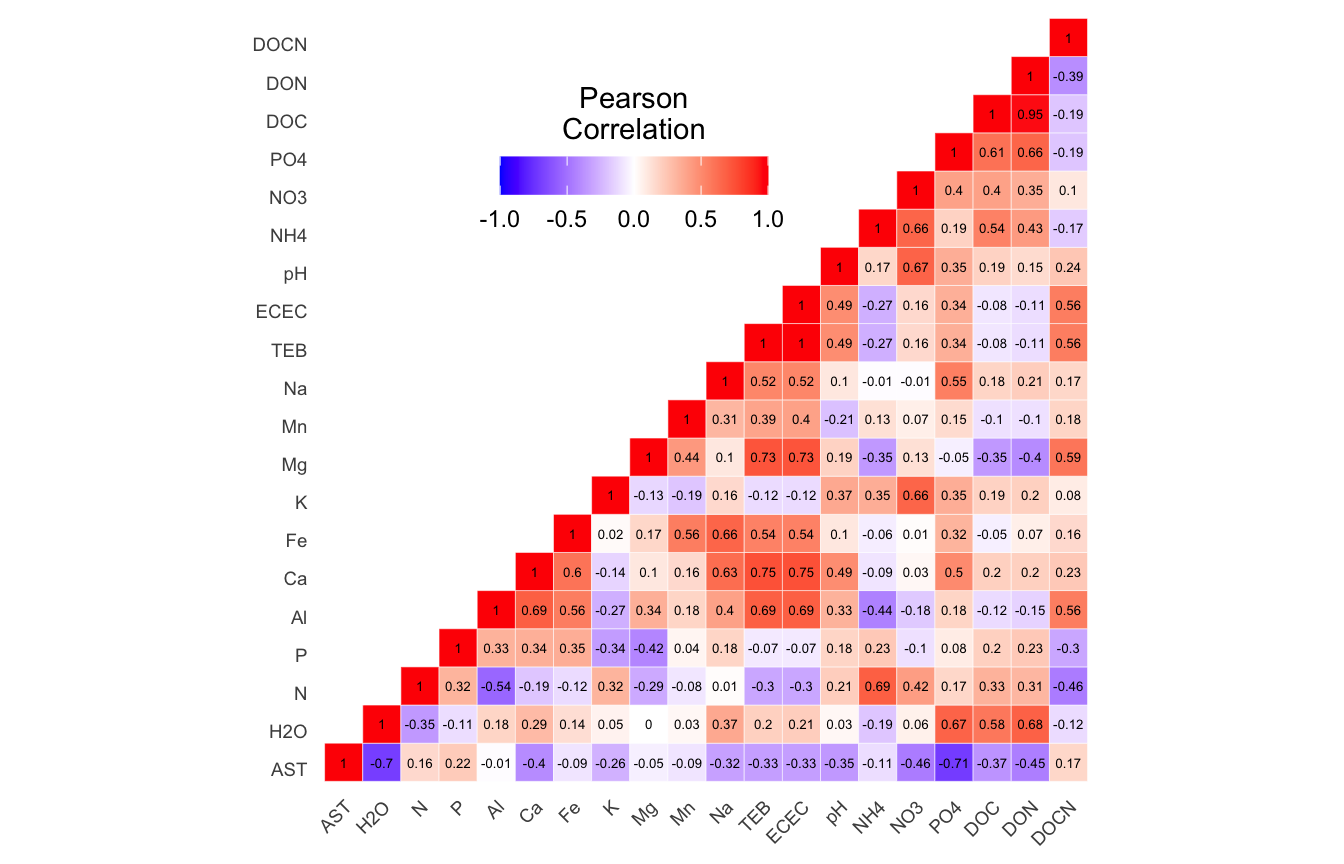(ITS) Figure 1 | Results of autocorrelation analysis for non-normalized Environmental and edaphic parameters.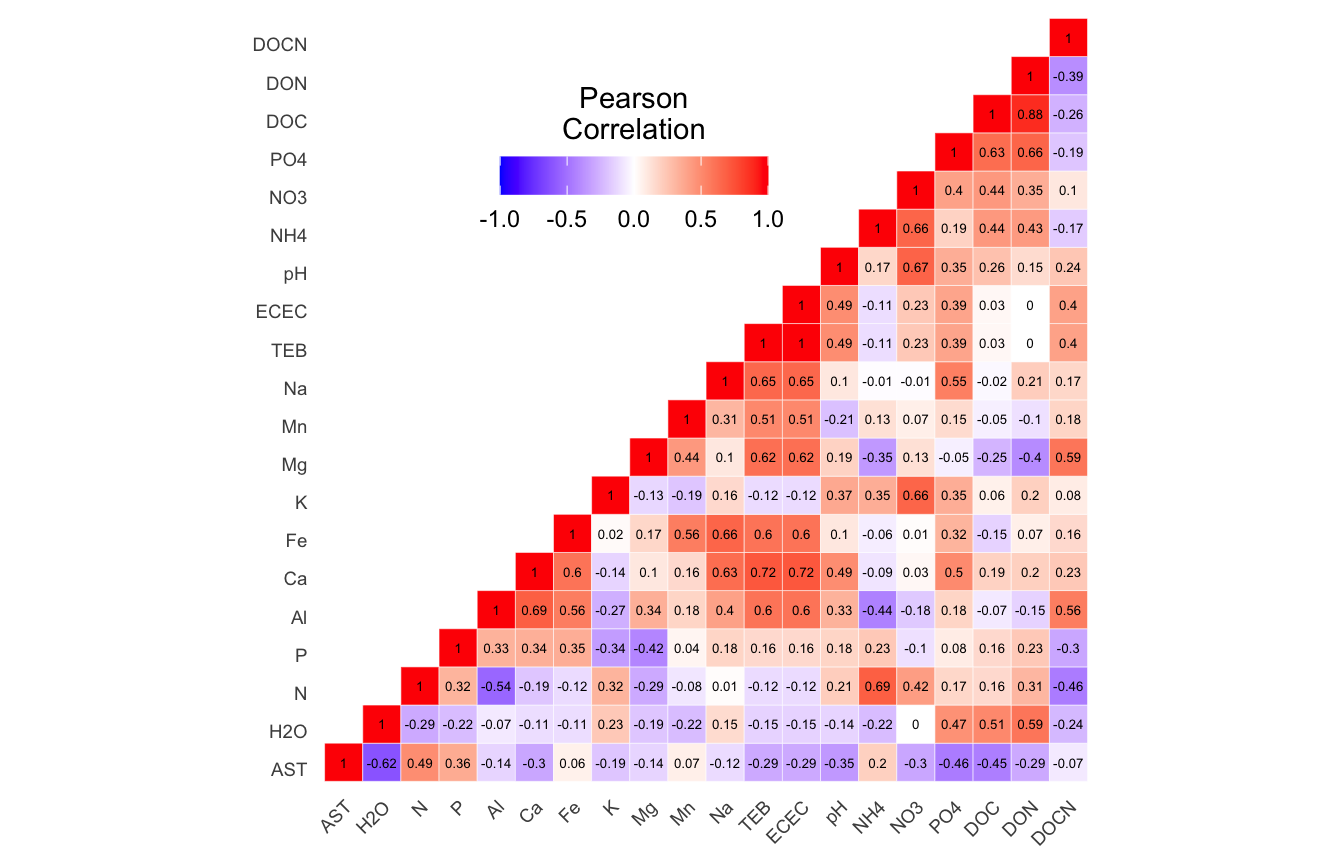(ITS) Figure 2 | Results of autocorrelation analysis for normalized Environmental and edaphic parameters.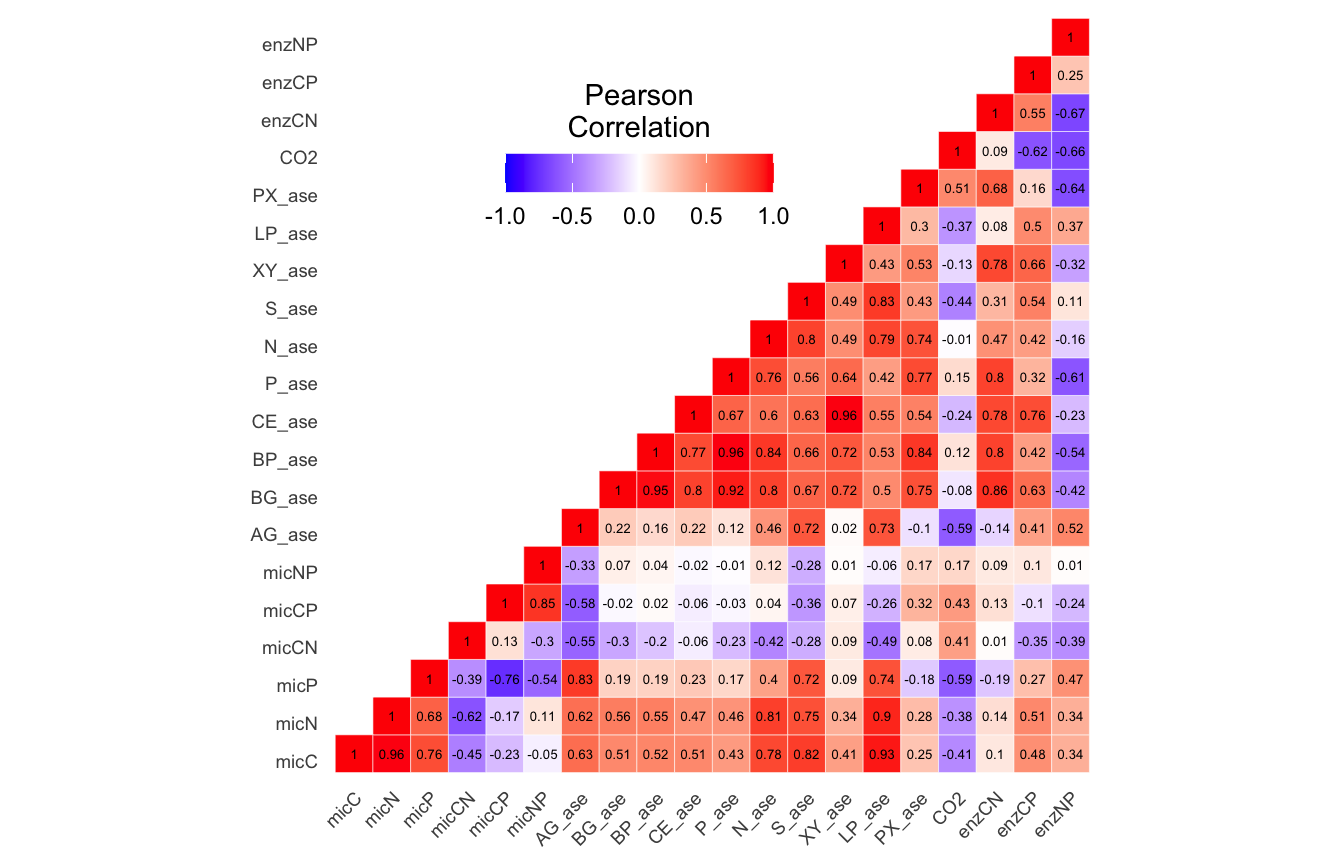(ITS) Figure 3 | Results of autocorrelation analysis for non-normalized Functional response parameters.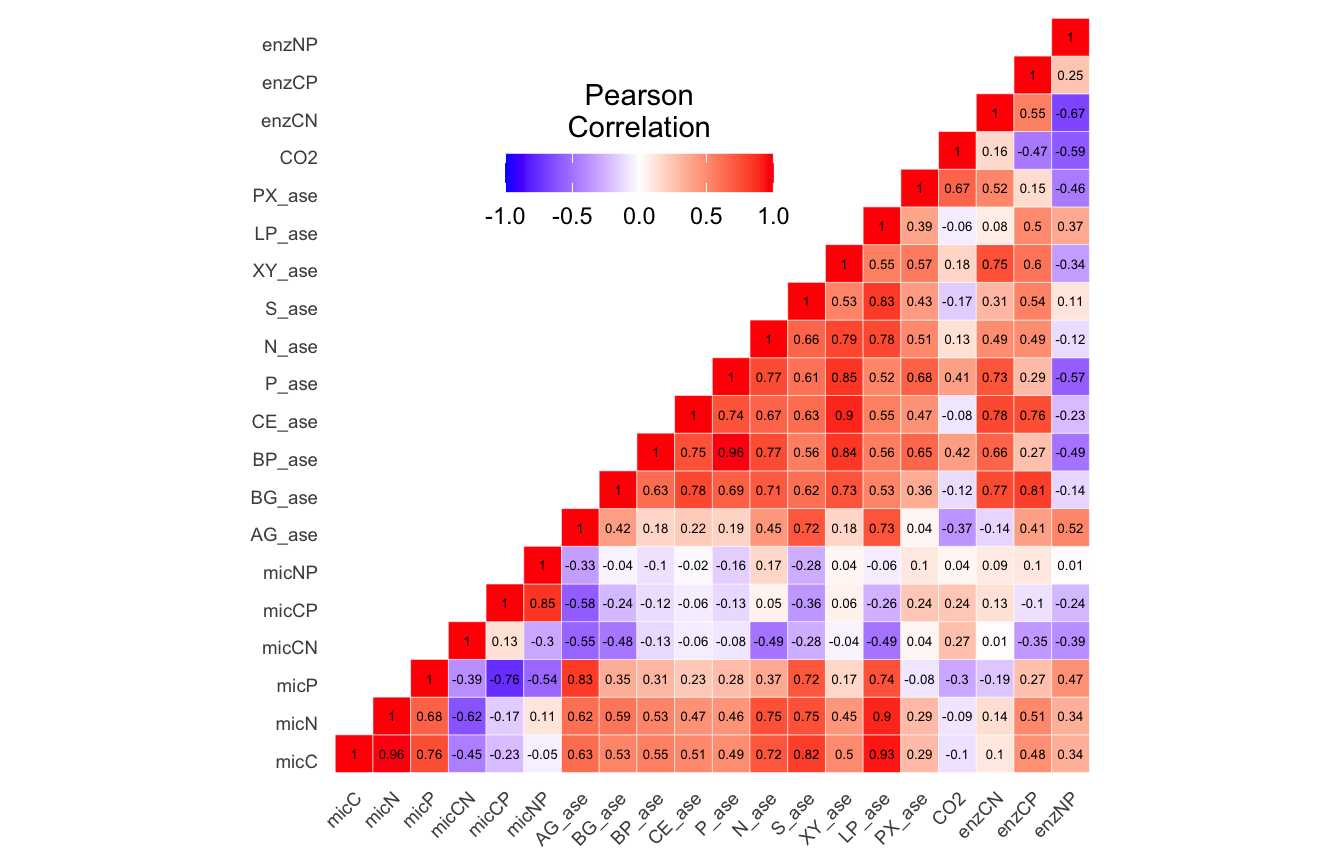(ITS) Figure 4 | Results of autocorrelation analysis for normalized Functional response parameters.(ITS) Figure 5 | Results of autocorrelation analysis for non-normalized Temperature adaptation parameters.(ITS) Figure 6 | Results of autocorrelation analysis for normalized Temperature adaptation parameters.

Now we can remove parameters based on the autocorrelation analysis:

1. Environmental and edaphic properties: TEB, DON, Na, Al, Ca.
2. Microbial functional responses: micN, micNP, enzCN, enzCP, BP_ase, CE_ase, LP_ase, N_ase, P_ase.
3. Temperature adaptation properties: NUE, PUE, P_Q10, SI.
``````edaphic_remove <- c("TEB", "DON", "Na", "Al", "Ca")
soil_funct_remove <- c("micN", "micNP", "enzCN", "enzCP", "BP_ase",
"CE_ase", "LP_ase", "N_ase", "P_ase")
temp_adapt_remove <- c("NUE", "PUE", "P_Q10", "SI")``````
Code
``````tmp_df <- its18_select_mc_split
tmp_df\$edaphic <- tmp_df\$edaphic[, ! names(tmp_df\$edaphic) %in%  edaphic_remove]
tmp_df\$soil_funct <- tmp_df\$soil_funct[, ! names(tmp_df\$soil_funct) %in%  soil_funct_remove]
its18_select_mc_split_no_ac <- tmp_df
rm(list = ls(pattern = "tmp_"))

tmp_df <- its18_select_mc_norm_split
tmp_df\$edaphic <- tmp_df\$edaphic[, ! names(tmp_df\$edaphic) %in%  edaphic_remove]
tmp_df\$soil_funct <- tmp_df\$soil_funct[, ! names(tmp_df\$soil_funct) %in%  soil_funct_remove]
its18_select_mc_norm_split_no_ac <- tmp_df
rm(list = ls(pattern = "tmp_"))``````

## Dissimilarity Correlation Tests

Let’s see if any on the metadata groups are significantly correlated with the community data. Basically, we create distance matrices for the community data and each metadata group and then run Mantel tests for all comparisons. For the community data we calculate Bray-Curtis distances for the community data and Euclidean distances for the metadata. We use the function `mantel.test` from the `ape` package and `mantel` from the `vegan` package for the analyses.

In summary, we test both `mantel.test` and `mantel` on Bray-Curtis distance community distances against Euclidean distances for each metadata group (`edaphic`, `soil_funct`, `temp_adapt`) a) before normalizing and before removing autocorrelated parameters, b) before normalizing and after removing autocorrelated parameters, c) after normalizing and before removing autocorrelated parameters, and d) after normalizing and after removing autocorrelated parameters.

Code
``````man_df <- c("its18_select_mc_split", "its18_select_mc_split_no_ac",
"its18_select_mc_norm_split", "its18_select_mc_norm_split_no_ac")

for (i in man_df) {
tmp_get <- get(i)
method = "bray", binary = FALSE,
diag = TRUE, upper = TRUE, na.rm = FALSE))
# EDAPHIC
tmp_dm_md_edaphic <- as.matrix(vegdist(tmp_get\$edaphic[, 8:ncol(tmp_get\$edaphic)],
method ="euclidean", binary = FALSE,
diag = TRUE, upper = TRUE, na.rm = FALSE))

tmp_man1_edaphic <- mantel.test(tmp_dm_otu, tmp_dm_md_edaphic, nperm = 999,
graph = FALSE, alternative = "two.sided")
tmp_man2_edaphic <- mantel(tmp_dm_otu, tmp_dm_md_edaphic, permutations = 999)
# SOIL FUNCT
tmp_dm_md_soil_funct <- as.matrix(vegdist(tmp_get\$soil_funct[, 8:ncol(tmp_get\$soil_funct)],
method = "euclidean", binary = FALSE,
diag = TRUE, upper = TRUE, na.rm = FALSE))

tmp_man1_soil_funct <- mantel.test(tmp_dm_otu, tmp_dm_md_soil_funct, nperm = 999,
graph = FALSE, alternative = "two.sided")
tmp_man2_soil_funct <- mantel(tmp_dm_otu, tmp_dm_md_soil_funct, permutations = 999)

method = "euclidean", binary = FALSE,
diag = TRUE, upper = TRUE, na.rm = FALSE))

graph = FALSE, alternative = "two.sided")

tmp_name <- purrr::map_chr(i, ~ paste0(., "_mantel_tests"))
tmp_df <- list(edaphic_ape_man = tmp_man1_edaphic,
edaphic_vegan_man = tmp_man2_edaphic,
soil_funct_ape_man = tmp_man1_soil_funct,
soil_funct_vegan_man = tmp_man2_soil_funct,
assign(tmp_name, tmp_df)
print(tmp_name)
rm(list = ls(pattern = "tmp_"))
}``````

### Dissimilarity Correlation Results

(ITS) Table 4 | Summary of Dissimilarity Correlation Tests using `mantel.test` from the ape package and `mantel` from the vegan. P-values in red indicate significance (p-value < 0.05)

Moving on.

## Best Subset of Variables

Now we want to know which of the metadata parameters are the most strongly correlated with the community data. For this we use the `bioenv` function from the `vegan` package. `bioenv`Best Subset of Environmental Variables with Maximum (Rank) Correlation with Community Dissimilarities—finds the best subset of environmental variables, so that the Euclidean distances of scaled environmental variables have the maximum (rank) correlation with community dissimilarities.

Since we know that each of the Mantel tests we ran above are significant, here we will use the metadata set where autocorrelated parameters were removed and the remainder of the parameters were normalized (where applicable based on the Shapiro tests).

We run `bioenv` against the three groups of metadata parameters. We then run `bioenv` again, but this time against the individual parameters identified as significantly correlated.

### Edaphic Properties

Code
``````tmp_comm <- data.frame(t(its18_select_mc_norm_split_no_ac\$data_loaded))
tmp_env <- data.frame(its18_select_mc_norm_split_no_ac\$edaphic)
tmp_env[,1:8] <- NULL

edaphic_bioenv <- bioenv(wisconsin(tmp_comm), tmp_env,
method = "spearman", index = "bray",
upto = ncol(tmp_env), metric = "euclidean")

bioenv_list <- edaphic_bioenv\$models[[edaphic_bioenv\$whichbest]]\$best
bioenv_best <- bioenvdist(edaphic_bioenv, which = "best")

for (i in bioenv_list) {
tmp_dp <- data.frame(edaphic_bioenv\$x)
tmp_md <- as.matrix(vegdist(tmp_dp[[i]],
method = "euclidean", binary = FALSE,
diag = TRUE, upper = TRUE, na.rm = FALSE))
tmp_man <- mantel(bioenv_best, tmp_md,
permutations = 999, method = "spearman")
tmp_md_name <- names(tmp_dp)[[i]]
tmp_name <- purrr::map_chr(tmp_md_name, ~ paste0(., "_bioenv_mantel_test"))
assign(tmp_name, tmp_man)
rm(list = ls(pattern = "tmp_"))
}
objects(pattern = "_bioenv_mantel_test")

edaphic_bioenv_ind_mantel <- list(AST = AST_bioenv_mantel_test)
rm(list = ls(pattern = "_bioenv_mantel_test"))``````
``````
Call:
bioenv(comm = wisconsin(tmp_comm), env = tmp_env, method = "spearman",      index = "bray", upto = ncol(tmp_env), metric = "euclidean")

Subset of environmental variables with best correlation to community data.

Correlations:    spearman
Dissimilarities: bray
Metric:          euclidean

Best model has 1 parameters (max. 15 allowed):
AST
with correlation  0.65665 ``````
Show the results of individual edaphic metadata Mantel tests
``````\$AST

Mantel statistic based on Spearman's rank correlation rho

Call:
mantel(xdis = bioenv_best, ydis = tmp_md, method = "spearman",      permutations = 999)

Mantel statistic r:     1
Significance: 0.001

Upper quantiles of permutations (null model):
90%   95% 97.5%   99%
0.192 0.242 0.272 0.339
Permutation: free
Number of permutations: 999``````

`bioenv` found the following edaphic properties significantly correlated with the community data: AST

### Soil Functional Response

Code
``````tmp_comm <- data.frame(t(its18_select_mc_norm_split_no_ac\$data_loaded))
tmp_env <- data.frame(its18_select_mc_norm_split_no_ac\$soil_funct)
tmp_env[,1:8] <- NULL

soil_funct_bioenv <- bioenv(wisconsin(tmp_comm), tmp_env,
method = "spearman", index = "bray",
upto = ncol(tmp_env), metric = "euclidean")

bioenv_list <- soil_funct_bioenv\$models[[soil_funct_bioenv\$whichbest]]\$best
bioenv_best <- bioenvdist(soil_funct_bioenv, which = "best")

for (i in bioenv_list) {
tmp_dp <- data.frame(soil_funct_bioenv\$x)
tmp_md <- as.matrix(vegdist(tmp_dp[[i]],
method = "euclidean", binary = FALSE,
diag = TRUE, upper = TRUE, na.rm = FALSE))
tmp_man <- mantel(bioenv_best, tmp_md,
permutations = 999, method = "spearman")
tmp_md_name <- names(tmp_dp)[[i]]
tmp_name <- purrr::map_chr(tmp_md_name, ~ paste0(., "_bioenv_mantel_test"))
assign(tmp_name, tmp_man)
rm(list = ls(pattern = "tmp_"))
}
objects(pattern = "_bioenv_mantel_test")

soil_funct_bioenv_ind_mantel <- list(enzNP = enzNP_bioenv_mantel_test,
PX_ase = PX_ase_bioenv_mantel_test,
XY_ase = XY_ase_bioenv_mantel_test)
rm(list = ls(pattern = "_bioenv_mantel_test"))``````
``````
Call:
bioenv(comm = wisconsin(tmp_comm), env = tmp_env, method = "spearman",      index = "bray", upto = ncol(tmp_env), metric = "euclidean")

Subset of environmental variables with best correlation to community data.

Correlations:    spearman
Dissimilarities: bray
Metric:          euclidean

Best model has 3 parameters (max. 11 allowed):
XY_ase PX_ase enzNP
with correlation  0.7491622 ``````
Show the results of individual functional response metadata Mantel tests
``````\$enzNP

Mantel statistic based on Spearman's rank correlation rho

Call:
mantel(xdis = bioenv_best, ydis = tmp_md, method = "spearman",      permutations = 999)

Mantel statistic r: 0.5529
Significance: 0.001

Upper quantiles of permutations (null model):
90%   95% 97.5%   99%
0.202 0.277 0.330 0.417
Permutation: free
Number of permutations: 999

\$PX_ase

Mantel statistic based on Spearman's rank correlation rho

Call:
mantel(xdis = bioenv_best, ydis = tmp_md, method = "spearman",      permutations = 999)

Mantel statistic r: 0.6854
Significance: 0.001

Upper quantiles of permutations (null model):
90%   95% 97.5%   99%
0.209 0.282 0.361 0.412
Permutation: free
Number of permutations: 999

\$XY_ase

Mantel statistic based on Spearman's rank correlation rho

Call:
mantel(xdis = bioenv_best, ydis = tmp_md, method = "spearman",      permutations = 999)

Mantel statistic r: 0.5054
Significance: 0.002

Upper quantiles of permutations (null model):
90%   95% 97.5%   99%
0.202 0.261 0.308 0.359
Permutation: free
Number of permutations: 999``````

`bioenv` found the following soil functions significantly correlated with the community data: enzNP, PX_ase, XY_ase

Code
``````tmp_comm <- data.frame(t(its18_select_mc_norm_split_no_ac\$data_loaded))
tmp_env[,1:8] <- NULL

method = "spearman", index = "bray",
upto = ncol(tmp_env), metric = "euclidean")

bioenv_best <- bioenvdist(temp_adapt_bioenv, which = "best")

for (i in bioenv_list) {
tmp_md <- as.matrix(vegdist(tmp_dp[[i]],
method = "euclidean", binary = FALSE,
diag = TRUE, upper = TRUE, na.rm = FALSE))
tmp_man <- mantel(bioenv_best, tmp_md,
permutations = 999, method = "spearman")
tmp_md_name <- names(tmp_dp)[[i]]
tmp_name <- purrr::map_chr(tmp_md_name, ~ paste0(., "_bioenv_mantel_test"))
assign(tmp_name, tmp_man)
rm(list = ls(pattern = "tmp_"))
}
objects(pattern = "_bioenv_mantel_test")

Tmin = Tmin_bioenv_mantel_test)
rm(list = ls(pattern = "_bioenv_mantel_test"))``````
``````
Call:
bioenv(comm = wisconsin(tmp_comm), env = tmp_env, method = "spearman",      index = "bray", upto = ncol(tmp_env), metric = "euclidean")

Subset of environmental variables with best correlation to community data.

Correlations:    spearman
Dissimilarities: bray
Metric:          euclidean

Best model has 2 parameters (max. 12 allowed):
XY_Q10 Tmin
with correlation  0.5637527 ``````
``````\$XY_Q10

Mantel statistic based on Spearman's rank correlation rho

Call:
mantel(xdis = bioenv_best, ydis = tmp_md, method = "spearman",      permutations = 999)

Mantel statistic r: 0.7258
Significance: 0.001

Upper quantiles of permutations (null model):
90%   95% 97.5%   99%
0.134 0.200 0.241 0.316
Permutation: free
Number of permutations: 999

\$Tmin

Mantel statistic based on Spearman's rank correlation rho

Call:
mantel(xdis = bioenv_best, ydis = tmp_md, method = "spearman",      permutations = 999)

Mantel statistic r: 0.616
Significance: 0.001

Upper quantiles of permutations (null model):
90%   95% 97.5%   99%
0.189 0.245 0.297 0.360
Permutation: free
Number of permutations: 999``````

`bioenv` found the following temperature adaptations significantly correlated with the community data: XY_Q10, Tmin

## Distance-based Redundancy

Now we turn our attention to distance-based redundancy analysis (dbRDA), an ordination method similar to Redundancy Analysis (rda) but it allows non-Euclidean dissimilarity indices, such as Manhattan or Bray–Curtis distance. For this, we use `capscale` from the `vegan` package. `capscale` is a constrained versions of metric scaling (principal coordinates analysis), which are based on the Euclidean distance but can be used, and are more useful, with other dissimilarity measures. The functions can also perform unconstrained principal coordinates analysis, optionally using extended dissimilarities.

For each of the three metadata subsets, we perform the following steps:

1. Run `rankindex` to compare metadata and community dissimilarity indices for gradient detection. This will help us select the best dissimilarity metric to use.
2. Run `capscale` for distance-based redundancy analysis.
3. Run `envfit` to fit environmental parameters onto the ordination. This function basically calculates correlation scores between the metadata parameters and the ordination axes.
4. Select metadata parameters significant for `bioenv` (see above) and/or `envfit` analyses.
5. Run `envfit` on ASVs.
6. Plot the ordination and vector overlays.

### Edaphic Properties

Code
``````tmp_md <- its18_select_mc_norm_split_no_ac\$edaphic
tmp_md\$TREAT_T <- as.character(tmp_md\$TREAT_T)
edaphic_rank <- rankindex(tmp_md[, 8:ncol(tmp_md)], tmp_comm,
indices = c("euc", "man", "gow","bra", "kul"),
stepacross = FALSE, method = "spearman")``````
``````        euc         man         gow         bra         kul
0.15398526  0.29983940 -0.02940098  0.29983940  0.29983940 ``````

Let’s run `capscale` using Bray-Curtis. Note, we have 15 metadata parameters in this group but, for some reason, `capscale` only works with 13 parameters. This may have to do with degrees of freedom?

• Starting properties: AST, H2O, N, P, Al, Ca, Fe, K, Mg, Mn, Na, TEB, ECEC, pH, NH4, NO3, PO4, DOC, DON, DOCN.
• Autocorrelated removed: TEB, DON, Na, Al, Ca.
• Remove for capscale: Mg, Mn, Na, Al, Fe, K
``````edaphic_cap <- capscale(tmp_comm ~ AST + H2O + N + P + ECEC + pH +
NH4 + NO3 + PO4 + DOC + DOCN,
tmp_md, dist = "bray")
colnames(tmp_md)``````
``````Call: capscale(formula = tmp_comm ~ AST + H2O + N + P + ECEC + pH + NH4
+ NO3 + PO4 + DOC + DOCN, data = tmp_md, distance = "bray")

Inertia Proportion Rank
Total         3.88624    1.00000
Constrained   3.66503    0.94308   11
Unconstrained 0.22120    0.05692    1
Inertia is squared Bray distance
Species scores projected from 'tmp_comm'

Eigenvalues for constrained axes:
CAP1   CAP2   CAP3   CAP4   CAP5   CAP6   CAP7   CAP8   CAP9  CAP10  CAP11
0.9655 0.5791 0.4327 0.3671 0.2987 0.2608 0.2301 0.1867 0.1339 0.1135 0.0970

Eigenvalues for unconstrained axes:
MDS1
0.2212 ``````

Now we can look at the variance against each principal component.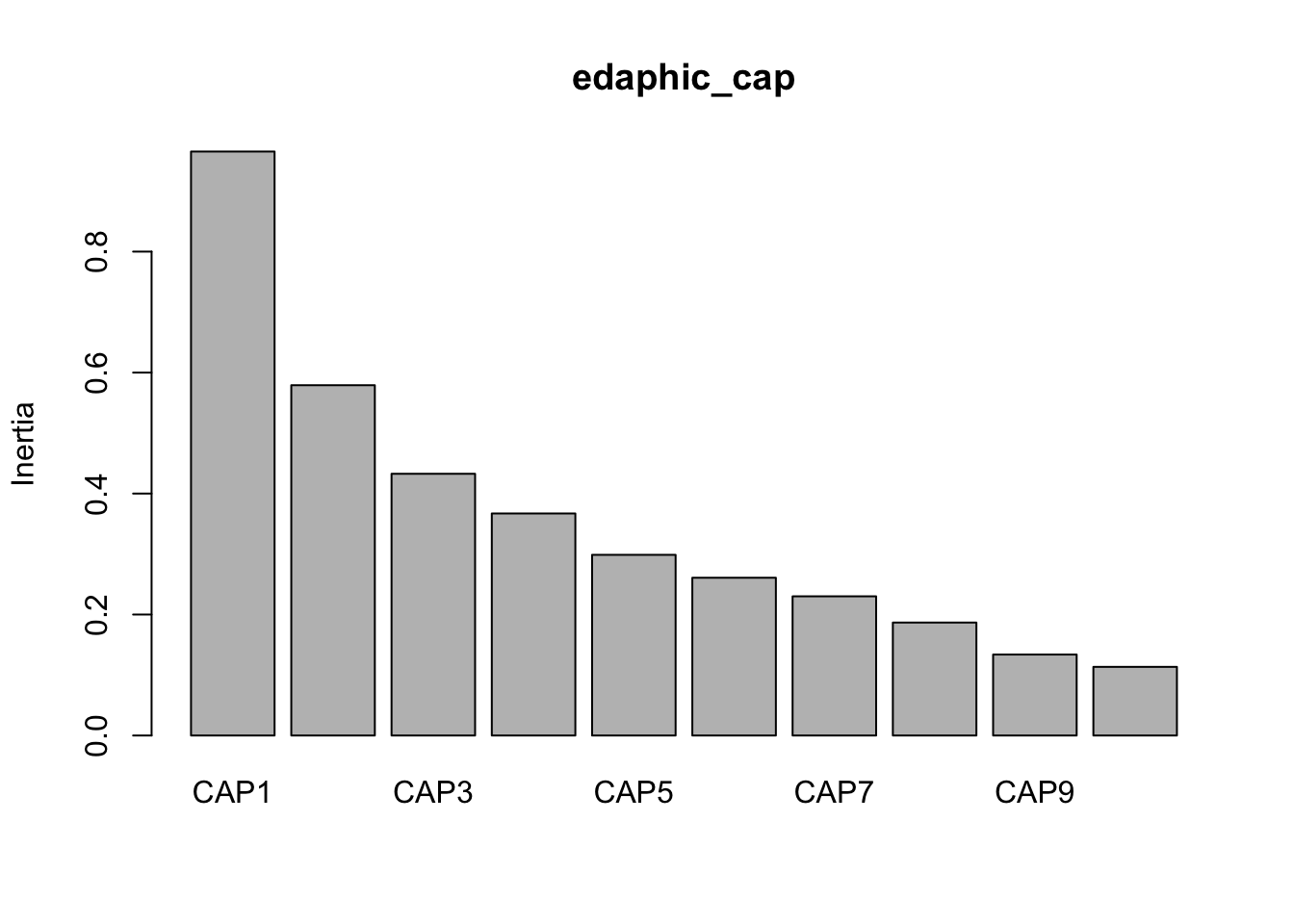And then make some quick and dirty plots. This will also come in handy later when we need to parse out data a better plot visualization. The `ggplot` function `autoplot` stores these data in a more accessible way than the raw results from `capscale`

``base::plot(edaphic_cap) ``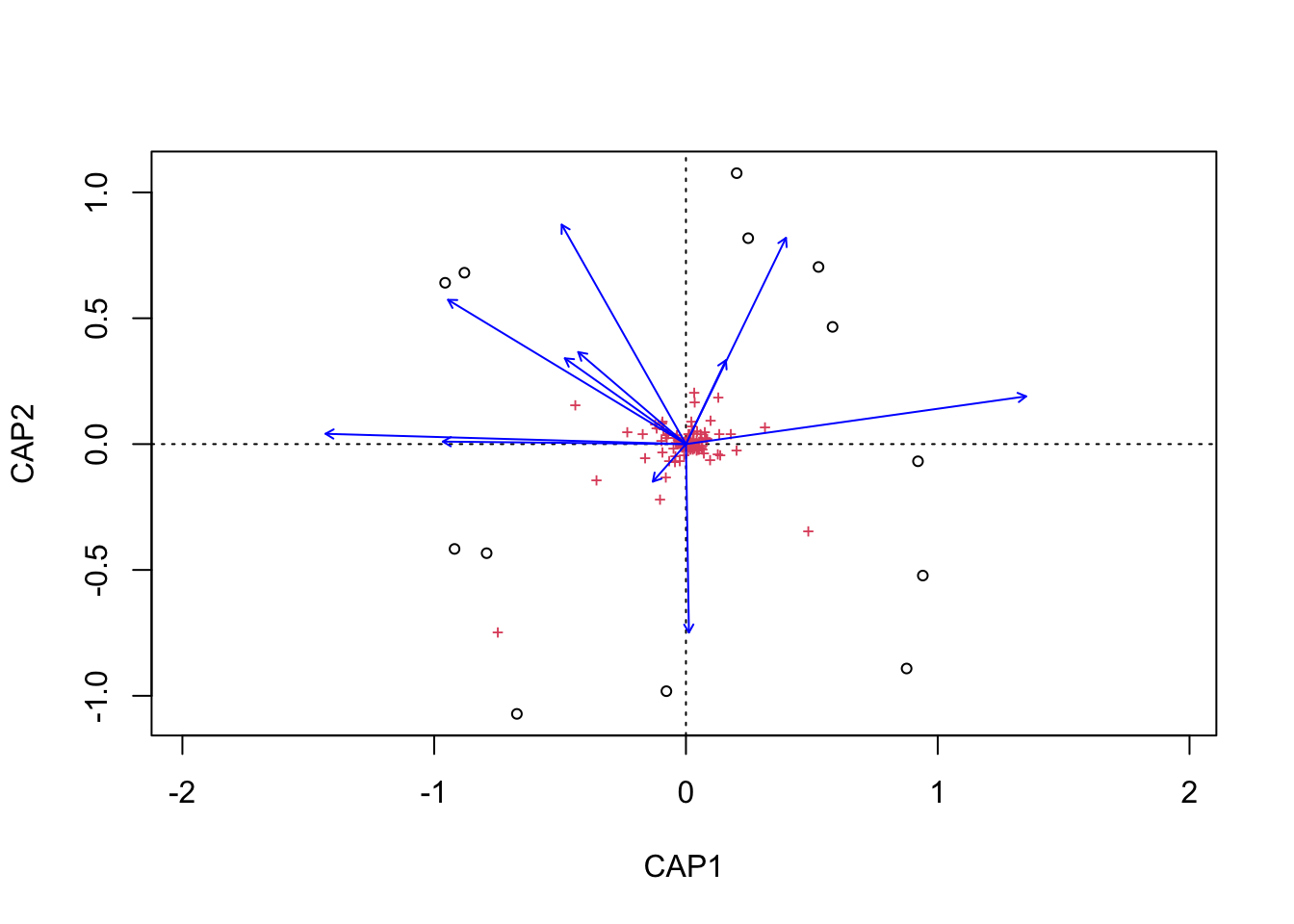``````tmp_auto_plt <- ggplot2::autoplot(edaphic_cap, arrows = TRUE)
tmp_auto_plt``````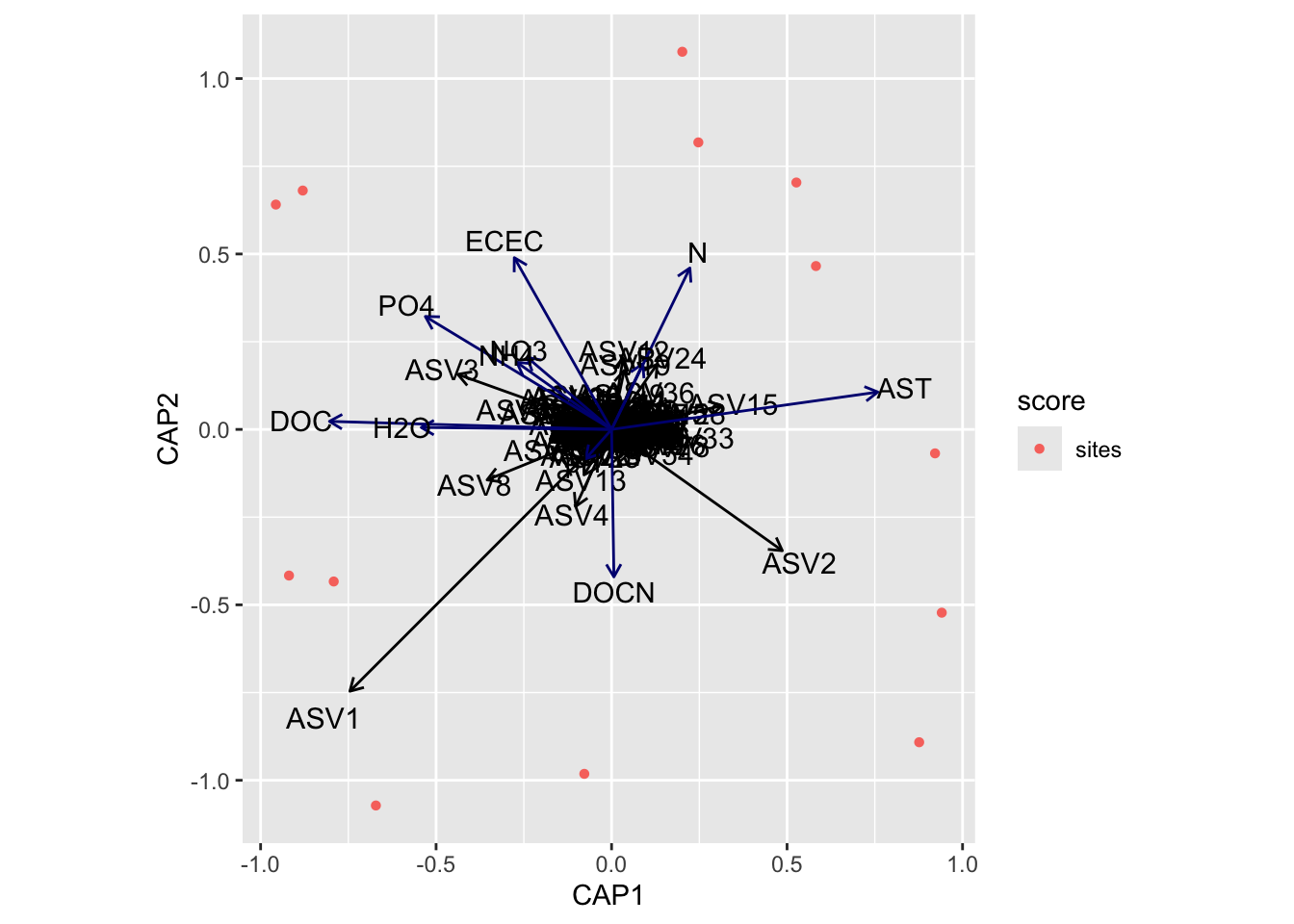Next, we need to grab capscale scores for the samples and create a data frame of the first two dimensions. We will also need to add some of the sample details to the data frame. For this we use the vegan function `scores` which gets species or site scores from the ordination.

Code
``````tmp_samp_scores <- dplyr::filter(tmp_auto_plt\$plot_env\$obj, Score == "sites")
tmp_samp_scores[,1] <- NULL
tmp_samp_scores <- tmp_samp_scores %>% dplyr::rename(SampleID = Label)

tmp_md_sub <- tmp_md[, 1:4]
tmp_md_sub <- tmp_md_sub %>% tibble::rownames_to_column("SampleID")
edaphic_plot_data <- dplyr::left_join(tmp_md_sub, tmp_samp_scores, by = "SampleID")``````

Now we have a new data frame that contains sample details and capscale values.

``````          SampleID PLOT TREAT TREAT_T PAIR        CAP1        CAP2
1  P10_D00_010_C0E  P10     C       0    E -0.67150210 -1.07183211
2  P02_D00_010_C0A  P02     C       0    A -0.91911652 -0.41647565
3  P04_D00_010_C0B  P04     C       0    B -0.95654588  0.64087450
4  P06_D00_010_C0C  P06     C       0    C -0.87992057  0.68082267
5  P08_D00_010_C0D  P08     C       0    D -0.07766291 -0.98166349
6  P01_D00_010_W3A  P01     W       3    A -0.79165161 -0.43340347
7  P03_D00_010_W3B  P03     W       3    B  0.24722812  0.81805851
8  P07_D00_010_W3D  P07     W       3    D  0.20151965  1.07639049
9  P09_D00_010_W3E  P09     W       3    E  0.58233467  0.46562279
10 P01_D00_010_W8A  P01     W       8    A  0.92158009 -0.06825914
11 P03_D00_010_W8B  P03     W       8    B  0.94079184 -0.52231363
12 P05_D00_010_W8C  P05     W       8    C  0.87642984 -0.89155825
13 P07_D00_010_W8D  P07     W       8    D  0.52651539  0.70373678``````

We can then do the same with the metadata vectors. Here though we only need the scores and parameter name.

Code
``````edaphic_md_scores <- dplyr::filter(tmp_auto_plt\$plot_env\$obj, Score == "biplot")
edaphic_md_scores[,1] <- NULL
edaphic_md_scores <- edaphic_md_scores %>%
dplyr::mutate(parameters = Label, .before = CAP1) %>%
tibble::column_to_rownames("Label")``````
``````     parameters         CAP1         CAP2
AST         AST  0.834527196  0.117196419
H2O         H2O -0.596509128  0.006131247
N             N  0.245428273  0.506082992
P             P  0.097845581  0.205793005
ECEC       ECEC -0.304860202  0.538475609
pH           pH -0.081297235 -0.092037201
NH4         NH4 -0.297079795  0.210528906
NO3         NO3 -0.264254841  0.225782469
PO4         PO4 -0.583698114  0.354036263
DOC         DOC -0.884261492  0.025360275
DOCN       DOCN  0.007258364 -0.462404778``````

Let’s run some quick correlations of metadata with ordination axes to see which parameters are significant. For this we use the vegan function `envfit`.

Code
``````tmp_samp_scores_sub <- edaphic_plot_data[, 6:7]
tmp_samp_scores_sub <- as.matrix(tmp_samp_scores_sub)
tmp_param_list <- edaphic_md_scores\$parameters

tmp_md_sub <- subset(tmp_md,  select =  tmp_param_list)

envfit_edaphic_md <- envfit(tmp_samp_scores_sub, tmp_md_sub,
perm = 1000, choices = c(1, 2))``````
``````
***VECTORS

CAP1     CAP2     r2  Pr(>r)
AST   0.99126  0.13189 0.4849 0.03696 *
H2O  -0.99989  0.01511 0.2434 0.25275
N     0.43981  0.89809 0.2124 0.27473
P     0.43273  0.90152 0.0349 0.86014
ECEC -0.50151  0.86515 0.2595 0.21778
pH   -0.66815 -0.74403 0.0102 0.94705
NH4  -0.81970  0.57279 0.0906 0.60140
NO3  -0.76538  0.64357 0.0824 0.64635
PO4  -0.85783  0.51393 0.3187 0.15185
DOC  -0.99945  0.03317 0.5354 0.02797 *
DOCN  0.02108 -0.99978 0.1437 0.47552
---
Signif. codes:  0 '***' 0.001 '**' 0.01 '*' 0.05 '.' 0.1 ' ' 1
Permutation: free
Number of permutations: 1000``````
Code
``````edaphic_md_signif_hits <- base::subset(envfit_edaphic_md\$vectors\$pvals,
c(envfit_edaphic_md\$vectors\$pvals
< 0.05 & envfit_edaphic_md\$vectors\$r > 0.4))
edaphic_md_signif_hits <- data.frame(edaphic_md_signif_hits)
edaphic_md_signif_hits <- rownames(edaphic_md_signif_hits)
edaphic_md_signif <- edaphic_md_scores[edaphic_md_scores\$parameters %in% edaphic_md_signif_hits,]
edaphic_md_signif\$parameters``````

`envfit` found that AST, DOC were significantly correlated.

Now let’s see if the same parameters are significant for the `envfit` and `bioenv` analyses.

`````` "Significant parameters from bioenv analysis."
 "AST"
_____________________________________
 "Significant parameters from envfit analysis."
 "AST" "DOC"
_____________________________________
 "Found in bioenv but not envfit."
character(0)
_____________________________________
 "Found in envfit but not bioenv."
 "DOC"
_____________________________________
 "Found in envfit and bioenv."
 "AST" "DOC"``````
Code
``````new_edaphic_md_signif_hits <- edaphic_sig_diff
#new_edaphic_md_signif_hits <- append(edaphic_md_signif_hits, edaphic_sig_diff)
edaphic_md_signif_all <- edaphic_md_scores[edaphic_md_scores\$parameters %in% new_edaphic_md_signif_hits,]``````

Check. Next, we run `envfit` for the ASVs.

Code
``````envfit_edaphic_asv <- envfit(tmp_samp_scores_sub,
tmp_comm[, order(colSums(-tmp_comm))][, 1:10],
perm = 1000, choices = c(1, 2))
edaphic_asv_scores <- dplyr::filter(tmp_auto_plt\$plot_env\$obj, Score == "species")
edaphic_asv_scores <- edaphic_asv_scores %>%
dplyr::mutate(parameters = Label, .before = CAP1) %>%
tibble::column_to_rownames("Label")
edaphic_asv_scores[,1] <- NULL``````
``````
***VECTORS

CAP1     CAP2     r2   Pr(>r)
ASV1  -0.61940 -0.78508 0.3539 0.010989 *
ASV2   0.73098 -0.68240 0.5575 0.003996 **
ASV4  -0.33832 -0.94103 0.4713 0.010989 *
ASV3  -0.89855  0.43888 0.4897 0.002997 **
ASV15  0.95614  0.29292 0.2269 0.192807
ASV8  -0.87871 -0.47735 0.1267 0.795205
ASV9   0.73176  0.68156 0.1410 0.468531
ASV24  0.45502  0.89048 0.1661 0.459540
ASV12  0.12678  0.99193 0.7513 0.001998 **
ASV13 -0.42157 -0.90680 0.4286 0.060939 .
---
Signif. codes:  0 '***' 0.001 '**' 0.01 '*' 0.05 '.' 0.1 ' ' 1
Permutation: free
Number of permutations: 1000``````
Code
``````edaphic_asv_signif_hits <- base::subset(envfit_edaphic_asv\$vectors\$pvals,
c(envfit_edaphic_asv\$vectors\$pvals
< 0.05 & envfit_edaphic_asv\$vectors\$r > 0.5))
edaphic_asv_signif_hits <- data.frame(edaphic_asv_signif_hits)
edaphic_asv_signif_hits <- rownames(edaphic_asv_signif_hits)
edaphic_asv_signif <- edaphic_asv_scores[edaphic_asv_scores\$parameters %in% edaphic_asv_signif_hits,]``````
``````      parameters       CAP1       CAP2 variable_type
ASV12      ASV12 0.03291551  0.2041479           ASV
ASV2        ASV2 0.48655008 -0.3456690           ASV``````
Code
``````edaphic_md_signif_all\$variable_type <- "metadata"
edaphic_asv_signif\$variable_type <- "ASV"
edaphic_bioplot_data <- rbind(edaphic_md_signif_all, edaphic_asv_signif)``````

The last thing to do is categorize parameters scores and ASV scores into different variable types for plotting.

Code
``````edaphic_bioplot_data_md <- subset(edaphic_bioplot_data,
edaphic_bioplot_data_asv <- subset(edaphic_bioplot_data,
edaphic_bioplot_data\$variable_type == "ASV")``````
Show the code for the plot

### Soil Functional Response

Code
``````tmp_md <- its18_select_mc_norm_split_no_ac\$soil_funct
tmp_md\$TREAT_T <- as.character(tmp_md\$TREAT_T)
soil_funct_rank <- rankindex(tmp_md[, 8:ncol(tmp_md)], tmp_comm,
indices = c("euc", "man", "gow", "bra", "kul"),
stepacross = FALSE, method = "spearman")``````
``````        euc         man         gow         bra         kul
0.06025620  0.38790324 -0.06200129  0.38790324  0.38790324 ``````

Let’s run `capscale` using Bray-Curtis Note, we have 11 metadata parameters in this group but, for some reason, `capscale` only works with 13 parameters. This may have to do with degrees of freedom?

• Starting properties: micC, micN, micP, micCN, micCP, micNP, AG_ase, BG_ase, BP_ase, CE_ase, P_ase, N_ase, S_ase, XY_ase, LP_ase, PX_ase, CO2, enzCN, enzCP, enzNP
• Autocorrelated removed: micN, micNP, enzCN, enzCP, BP_ase, CE_ase, LP_ase, N_ase, P_ase
• Remove for capscale: NONE
``````soil_funct_cap <- capscale(tmp_comm ~  micC + micP + micCN + micCP + AG_ase + BG_ase +
S_ase + XY_ase + PX_ase + CO2 + enzNP,
tmp_md, dist = "bray")``````
``````Call: capscale(formula = tmp_comm ~ micC + micP + micCN + micCP +
AG_ase + BG_ase + S_ase + XY_ase + PX_ase + CO2 + enzNP, data = tmp_md,
distance = "bray")

Inertia Proportion Rank
Total         3.88624    1.00000
Constrained   3.59902    0.92609   11
Unconstrained 0.28722    0.07391    1
Inertia is squared Bray distance
Species scores projected from 'tmp_comm'

Eigenvalues for constrained axes:
CAP1   CAP2   CAP3   CAP4   CAP5   CAP6   CAP7   CAP8   CAP9  CAP10  CAP11
0.9666 0.5891 0.3939 0.3377 0.2686 0.2635 0.2306 0.1940 0.1389 0.1143 0.1018

Eigenvalues for unconstrained axes:
MDS1
0.28722 ``````

Now we can look at the variance against each principal component.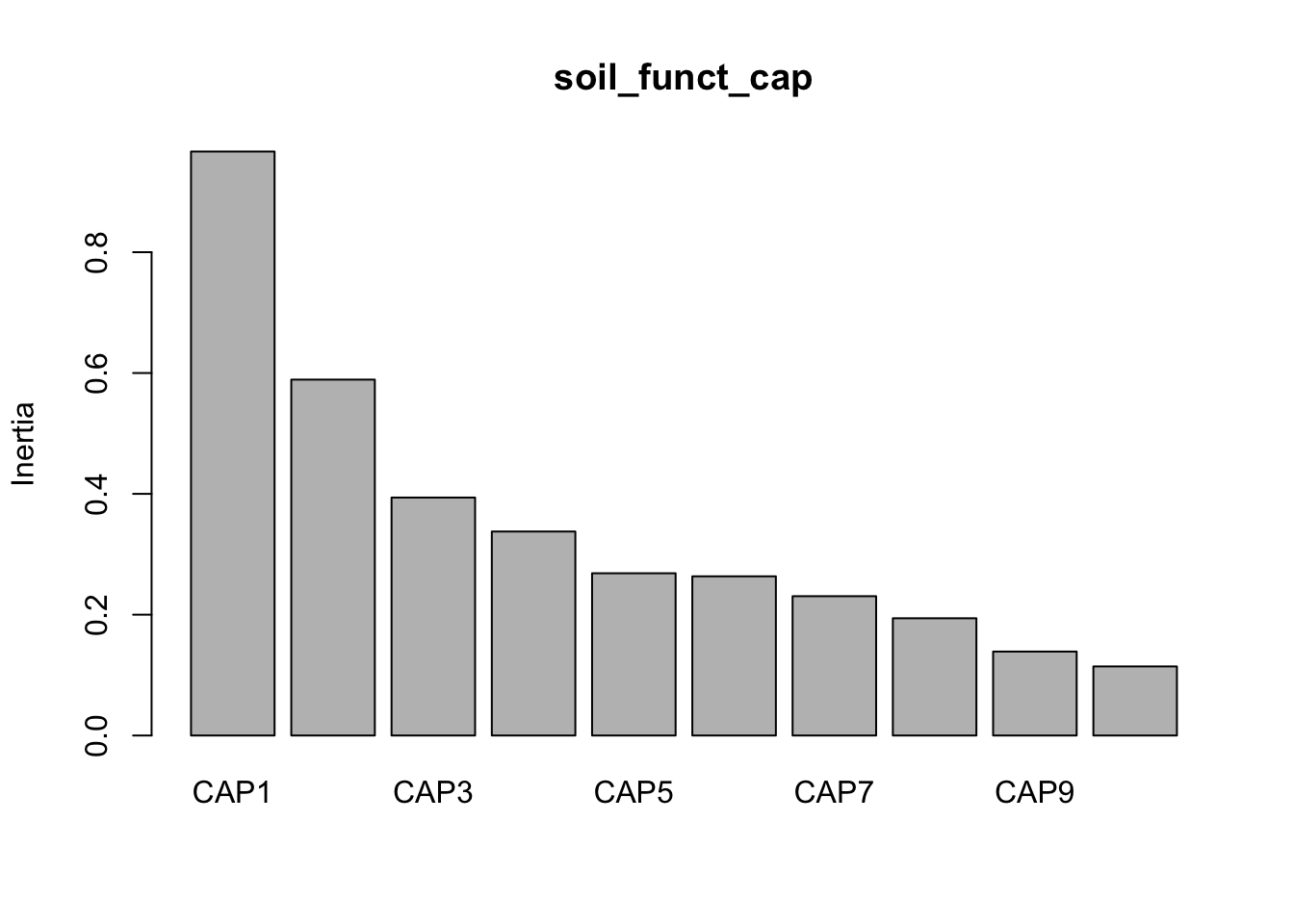And then make some quick and dirty plots. This will also come in handy later when we need to parse out data a better plot visualization. The `ggplot` function `autoplot` stores these data in a more accessible way than the raw results from `capscale`

``base::plot(soil_funct_cap) ``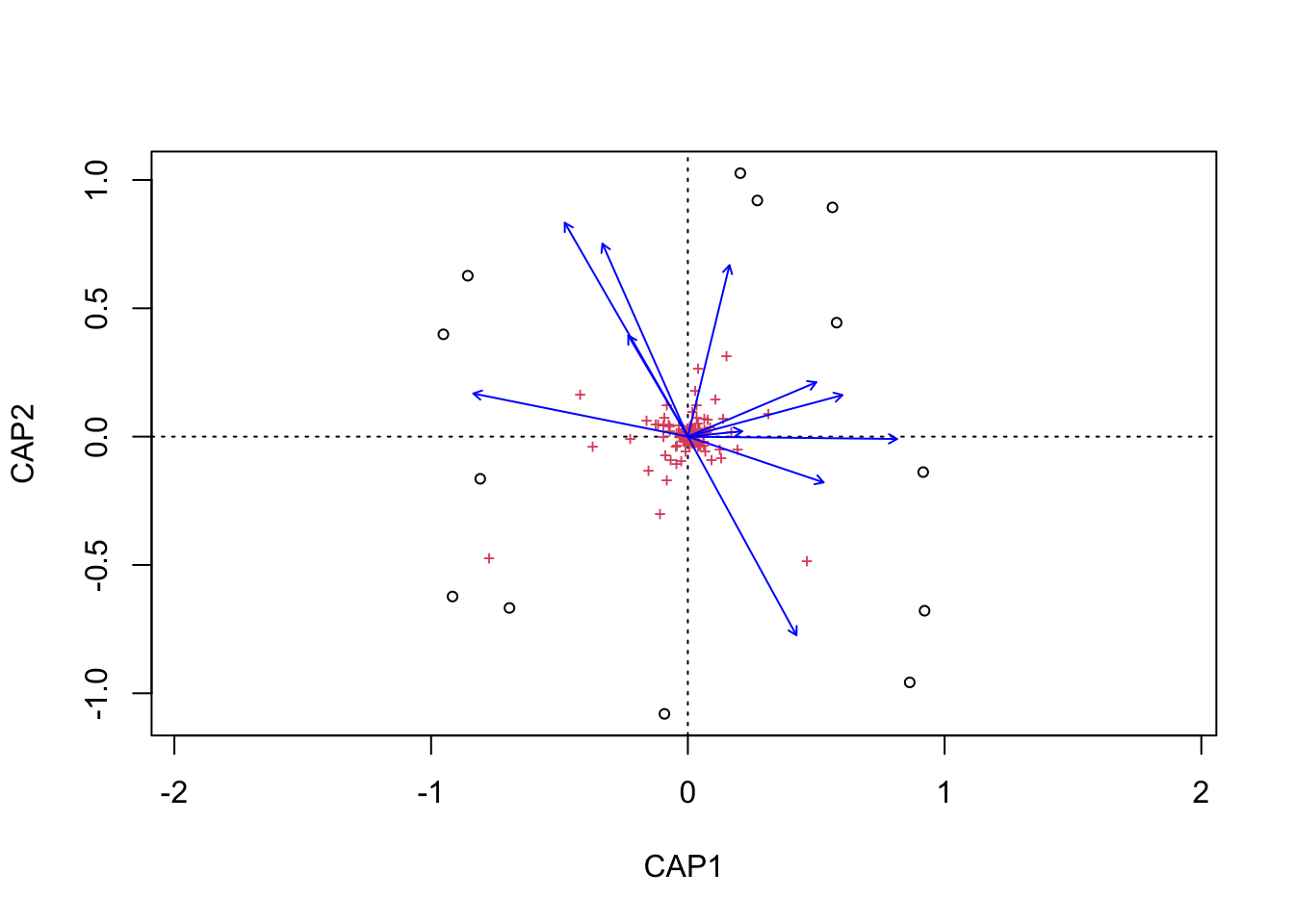``````tmp_auto_plt <- autoplot(soil_funct_cap, arrows = TRUE)
tmp_auto_plt``````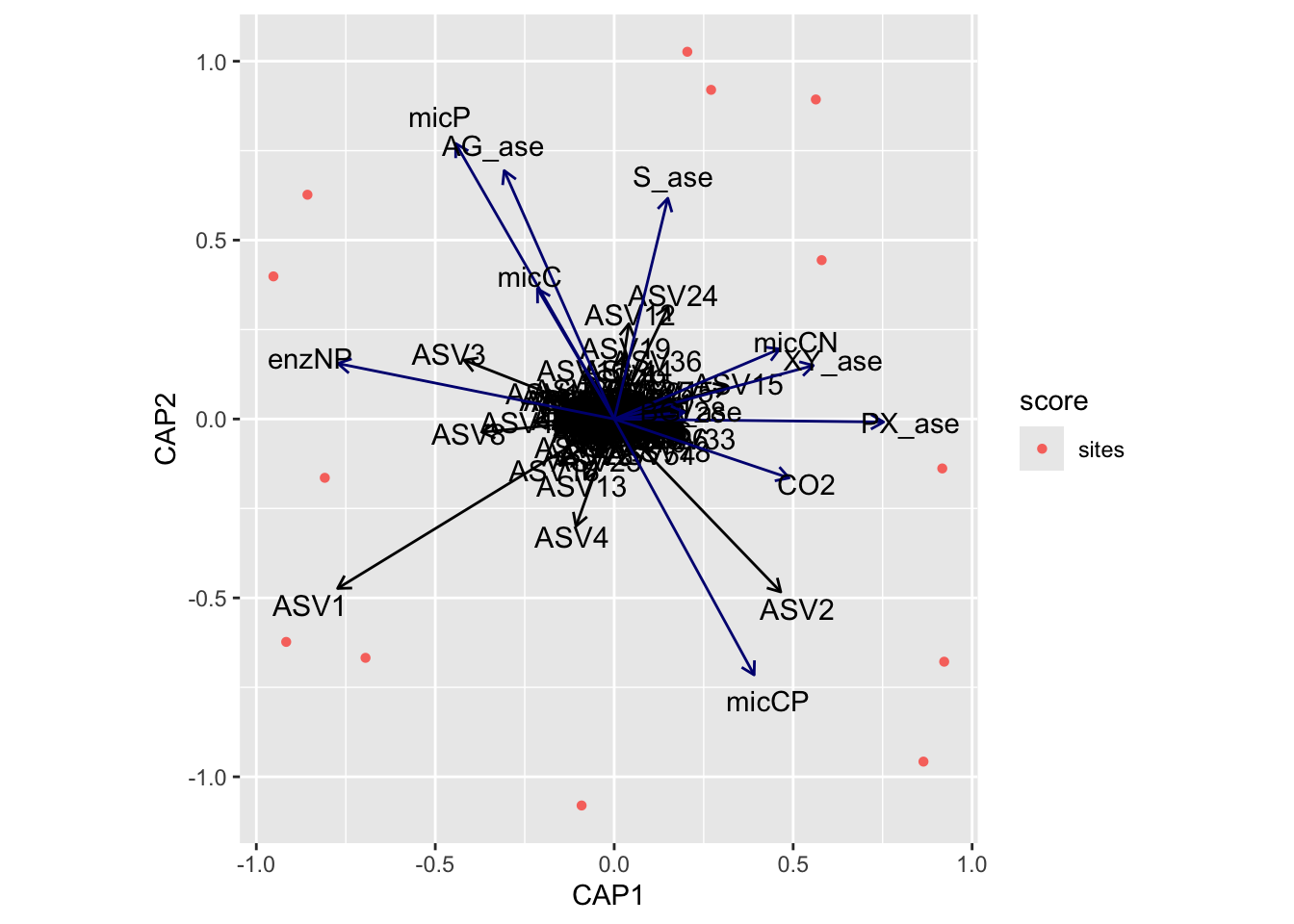Next, we need to grab capscale scores for the samples and create a data frame of the first two dimensions. We will also need to add some of the sample details to the data frame. For this we use the vegan function `scores` which gets species or site scores from the ordination.

Code
``````tmp_samp_scores <- dplyr::filter(tmp_auto_plt\$plot_env\$obj, Score == "sites")
tmp_samp_scores[,1] <- NULL
tmp_samp_scores <- tmp_samp_scores %>% dplyr::rename(SampleID = Label)

tmp_md_sub <- tmp_md[, 1:4]
tmp_md_sub <- tmp_md_sub %>% tibble::rownames_to_column("SampleID")
soil_funct_plot_data <- dplyr::left_join(tmp_md_sub, tmp_samp_scores,
by = "SampleID")``````

Now we have a new data frame that contains sample details and capscale values.

``````          SampleID PLOT TREAT TREAT_T PAIR        CAP1       CAP2
1  P10_D00_010_C0E  P10     C       0    E -0.69489162 -0.6675049
2  P02_D00_010_C0A  P02     C       0    A -0.91653485 -0.6229848
3  P04_D00_010_C0B  P04     C       0    B -0.95221897  0.3986135
4  P06_D00_010_C0C  P06     C       0    C -0.85713972  0.6268677
5  P08_D00_010_C0D  P08     C       0    D -0.09115803 -1.0802252
6  P01_D00_010_W3A  P01     W       3    A -0.80868973 -0.1643386
7  P03_D00_010_W3B  P03     W       3    B  0.27067347  0.9200087
8  P07_D00_010_W3D  P07     W       3    D  0.20427135  1.0264780
9  P09_D00_010_W3E  P09     W       3    E  0.57963547  0.4440199
10 P01_D00_010_W8A  P01     W       8    A  0.91663981 -0.1382072
11 P03_D00_010_W8B  P03     W       8    B  0.92199435 -0.6783194
12 P05_D00_010_W8C  P05     W       8    C  0.86406950 -0.9575454
13 P07_D00_010_W8D  P07     W       8    D  0.56334898  0.8931377``````

We can then do the same with the metadata vectors. Here though we only need the scores and parameter name.

Code
``````soil_funct_md_scores <- dplyr::filter(tmp_auto_plt\$plot_env\$obj, Score == "biplot")
soil_funct_md_scores[,1] <- NULL
soil_funct_md_scores <- soil_funct_md_scores %>%
dplyr::mutate(parameters = Label, .before = CAP1) %>%
tibble::column_to_rownames("Label")``````
``````       parameters       CAP1         CAP2
micC         micC -0.2359175  0.400384343
micP         micP -0.4872005  0.846844390
micCN       micCN  0.5085406  0.215263003
micCP       micCP  0.4299937 -0.786734788
AG_ase     AG_ase -0.3381241  0.763769448
BG_ase     BG_ase  0.2150249  0.021210923
S_ase       S_ase  0.1648172  0.678698244
XY_ase     XY_ase  0.6119362  0.164529372
PX_ase     PX_ase  0.8280069 -0.009182309
CO2           CO2  0.5373302 -0.181241128
enzNP       enzNP -0.8493447  0.170758052``````

Let’s run some quick correlations of metadata with ordination axes to see which parameters are significant. For this we use the vegan function `envfit`.

Code
``````tmp_samp_scores_sub <- soil_funct_plot_data[, 6:7]
tmp_samp_scores_sub <- as.matrix(tmp_samp_scores_sub)
tmp_param_list <- soil_funct_md_scores\$parameters

tmp_md_sub <- subset(tmp_md,  select =  tmp_param_list)

envfit_soil_funct_md <- envfit(tmp_samp_scores_sub, tmp_md_sub,
perm = 1000, choices = c(1, 2))``````
``````
***VECTORS

CAP1     CAP2     r2   Pr(>r)
micC   -0.50911  0.86070 0.1567 0.442557
micP   -0.50009  0.86597 0.6925 0.001998 **
micCN   0.92125  0.38897 0.2225 0.261738
micCP   0.48092 -0.87676 0.5831 0.015984 *
AG_ase -0.40574  0.91399 0.5060 0.036963 *
BG_ase  0.99507  0.09917 0.0341 0.832168
S_ase   0.23862  0.97111 0.3542 0.099900 .
XY_ase  0.96571  0.25962 0.2931 0.170829
PX_ase  0.99996 -0.00944 0.5002 0.034965 *
CO2     0.94850 -0.31679 0.2342 0.250749
enzNP  -0.98088  0.19460 0.5471 0.013986 *
---
Signif. codes:  0 '***' 0.001 '**' 0.01 '*' 0.05 '.' 0.1 ' ' 1
Permutation: free
Number of permutations: 1000``````
Code
``````soil_funct_md_signif_hits <- base::subset(envfit_soil_funct_md\$vectors\$pvals,
c(envfit_soil_funct_md\$vectors\$pvals
< 0.05 & envfit_soil_funct_md\$vectors\$r > 0.4))
soil_funct_md_signif_hits <- data.frame(soil_funct_md_signif_hits)
soil_funct_md_signif_hits <- rownames(soil_funct_md_signif_hits)
soil_funct_md_signif <- soil_funct_md_scores[soil_funct_md_scores\$parameters %in%
soil_funct_md_signif_hits,]
soil_funct_md_signif\$parameters``````

`envfit` found that micP, micCP, AG_ase, PX_ase, enzNP were significantly correlated.

Now let’s see if the same parameters are significant for the `envfit` and `bioenv` analyses.

`````` "Significant parameters from bioenv analysis."
 "enzNP"  "PX_ase" "XY_ase"
_____________________________________
 "Significant parameters from envfit analysis."
 "micP"   "micCP"  "AG_ase" "PX_ase" "enzNP"
_____________________________________
 "Found in bioenv but not envfit."
 "XY_ase"
_____________________________________
 "Found in envfit but not bioenv."
 "micP"   "micCP"  "AG_ase"
_____________________________________
 "Found in envfit and bioenv."
 "micP"   "micCP"  "AG_ase" "PX_ase" "enzNP"  "XY_ase"``````
Code
``````#new_soil_funct_md_signif_hits <- append(soil_funct_md_signif_hits, soil_funct_sig_diff)
new_soil_funct_md_signif_hits <- soil_funct_sig_diff
soil_funct_md_signif_all <- soil_funct_md_scores[soil_funct_md_scores\$parameters %in%
new_soil_funct_md_signif_hits,]``````

Check. Next, we run `envfit` for the ASVs.

Code
``````envfit_soil_funct_asv <- envfit(tmp_samp_scores_sub,
tmp_comm[, order(colSums(-tmp_comm))][, 1:10],
perm = 1000, choices = c(1, 2))
soil_funct_asv_scores <- dplyr::filter(tmp_auto_plt\$plot_env\$obj, Score == "species")
soil_funct_asv_scores <- soil_funct_asv_scores %>%
dplyr::mutate(parameters = Label, .before = CAP1) %>%
tibble::column_to_rownames("Label")
soil_funct_asv_scores[,1] <- NULL``````
``````
***VECTORS

CAP1     CAP2     r2   Pr(>r)
ASV1  -0.79963 -0.60050 0.2256 0.245754
ASV2   0.68178 -0.73156 0.6129 0.000999 ***
ASV4  -0.33208 -0.94325 0.5433 0.000999 ***
ASV3  -0.92213  0.38687 0.4516 0.010989 *
ASV15  0.95847  0.28518 0.2287 0.159840
ASV8  -0.97867 -0.20544 0.1064 0.930070
ASV9   0.76380  0.64546 0.1326 0.481518
ASV24  0.40983  0.91216 0.2438 0.065934 .
ASV12  0.14788  0.98901 0.7872 0.000999 ***
ASV13 -0.42711 -0.90420 0.4546 0.044955 *
---
Signif. codes:  0 '***' 0.001 '**' 0.01 '*' 0.05 '.' 0.1 ' ' 1
Permutation: free
Number of permutations: 1000``````
Code
``````soil_funct_asv_signif_hits <- base::subset(envfit_soil_funct_asv\$vectors\$pvals,
c(envfit_soil_funct_asv\$vectors\$pvals
< 0.05 & envfit_soil_funct_asv\$vectors\$r > 0.5))
soil_funct_asv_signif_hits <- data.frame(soil_funct_asv_signif_hits)
soil_funct_asv_signif_hits <- rownames(soil_funct_asv_signif_hits)
soil_funct_asv_signif <- soil_funct_asv_scores[soil_funct_asv_scores\$parameters %in%
soil_funct_asv_signif_hits,]``````
``````      parameters        CAP1       CAP2 variable_type
ASV4        ASV4 -0.10677580 -0.2996742           ASV
ASV12      ASV12  0.04088024  0.2662493           ASV
ASV2        ASV2  0.46539405 -0.4834130           ASV``````
Code
``````soil_funct_md_signif_all\$variable_type <- "metadata"
soil_funct_asv_signif\$variable_type <- "ASV"
soil_funct_bioplot_data <- rbind(soil_funct_md_signif_all, soil_funct_asv_signif)``````

The last thing to do is categorize parameters scores and ASV scores into different variable types for plotting.

Code
``````soil_funct_bioplot_data_md <- subset(soil_funct_bioplot_data,
soil_funct_bioplot_data_asv <- subset(soil_funct_bioplot_data,
soil_funct_bioplot_data\$variable_type == "ASV")``````
Show the code for the plot
``````soil_funct_cap_vals <- data.frame(soil_funct_cap\$CCA\$eig[1:2])
soil_funct_cap1 <- signif((soil_funct_cap_vals[1,] * 100), digits=3)
soil_funct_cap2 <- signif((soil_funct_cap_vals[2,] * 100), digits=3)

cpa1_lab <- paste("CAP1", " (", soil_funct_cap1, "%)", sep = "")
cpa2_lab <- paste("CAP2", " (", soil_funct_cap2, "%)", sep = "")

swel_col <- c("#2271B2", "#71B222", "#B22271")

soil_funct_plot <- ggplot(soil_funct_plot_data) +
geom_point(mapping = aes(x = CAP1, y = CAP2, shape = TREAT,
colour = TREAT_T), size = 4) +
scale_colour_manual(values = swel_col) +
#  geom_text(data = soil_funct_plot_data, aes(x = CAP1, y = CAP2, #UNCOMMENT to add sample labels
#                              label = SampleID), size = 3) +
geom_segment(aes(x = 0, y = 0, xend = CAP1, yend = CAP2),
data = soil_funct_bioplot_data_md, linetype = "solid",
arrow = arrow(length = unit(0.3, "cm")), size = 0.4,
color = "#191919") +
geom_text(data = soil_funct_bioplot_data_md,
aes(x = CAP1, y = CAP2, label = parameters), size = 3,
nudge_x = 0.1, nudge_y = 0.05) +
## USE this code to overlay ASV vestors
#geom_segment(aes(x = 0, y = 0, xend = CAP1, yend = CAP2),
#             data = soil_funct_bioplot_data_asv, linetype = "solid",
#             arrow = arrow(length = unit(0.3, "cm")), size = 0.2,
#             color = "#676767") +
#geom_text(data = soil_funct_bioplot_data_asv,
#          aes(x = CAP1, y = CAP2, label = parameters), size = 2.5,
#          nudge_x = 0.05, nudge_y = 0.05) +
theme_classic(base_size = 12) +
labs(title = "Capscale Analysis",
subtitle = "Soil Functional Response",
x = cpa1_lab,
y = cpa2_lab)
soil_funct_plot <- soil_funct_plot + coord_fixed() + theme(aspect.ratio=1)
soil_funct_plot
height = 16, width = 20, units = 'cm', res = 600, bg = "white")
soil_funct_plot
invisible(dev.off())
height = 5, width = 6)
soil_funct_plot
dev.off()``````

Code
``````tmp_md <- its18_select_mc_norm_split_no_ac\$temp_adapt
tmp_md\$TREAT_T <- as.character(tmp_md\$TREAT_T)
indices = c("euc", "man", "gow", "bra", "kul"),
stepacross = FALSE, method = "spearman")``````
``````       euc        man        gow        bra        kul
0.05132842 0.39465598 0.27457353 0.39465598 0.39465598 ``````

Let’s run `capscale` using Bray-Curtis. Note, we have 12 metadata parameters in this group but, for some reason, `capscale` only works with 13 parameters. This may have to do with degrees of freedom?

• Starting properties: AG_Q10, BG_Q10, BP_Q10, CE_Q10, P_Q10, N_Q10, S_Q10, XY_Q10, LP_Q10, PX_Q10, CUEcn, CUEcp, NUE, PUE, Tmin, SI
• Autocorrelated removed: NUE, PUE, P_Q10, SI
• Remove for capscale: S_Q10
``````temp_adapt_cap <- capscale(tmp_comm ~  AG_Q10 + BG_Q10 + BP_Q10 + CE_Q10 +
N_Q10 + XY_Q10 + LP_Q10 + PX_Q10 +
CUEcn + CUEcp + Tmin,
tmp_md, dist = "bray")``````
``````Call: capscale(formula = tmp_comm ~ AG_Q10 + BG_Q10 + BP_Q10 + CE_Q10 +
N_Q10 + XY_Q10 + LP_Q10 + PX_Q10 + CUEcn + CUEcp + Tmin, data = tmp_md,
distance = "bray")

Inertia Proportion Rank
Total         3.88624    1.00000
Constrained   3.64416    0.93771   11
Unconstrained 0.24208    0.06229    1
Inertia is squared Bray distance
Species scores projected from 'tmp_comm'

Eigenvalues for constrained axes:
CAP1   CAP2   CAP3   CAP4   CAP5   CAP6   CAP7   CAP8   CAP9  CAP10  CAP11
0.9367 0.5976 0.4447 0.3685 0.3136 0.2644 0.2093 0.1488 0.1377 0.1235 0.0994

Eigenvalues for unconstrained axes:
MDS1
0.24208 ``````

Now we can look at the variance against each principal component.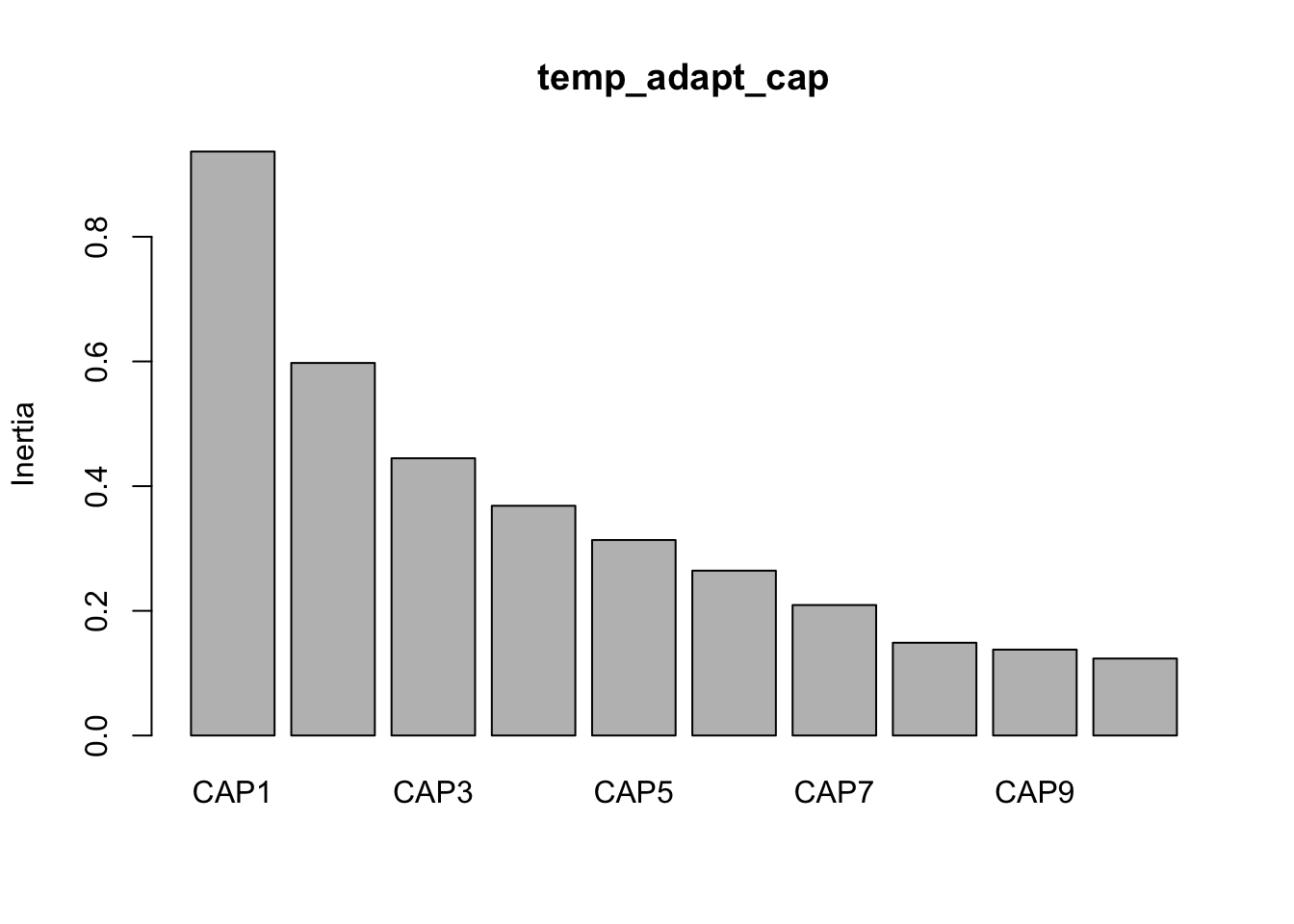And then make some quick and dirty plots. This will also come in handy later when we need to parse out data a better plot visualization. The `ggplot` function `autoplot` stores these data in a more accessible way than the raw results from `capscale`

``base::plot(temp_adapt_cap) ``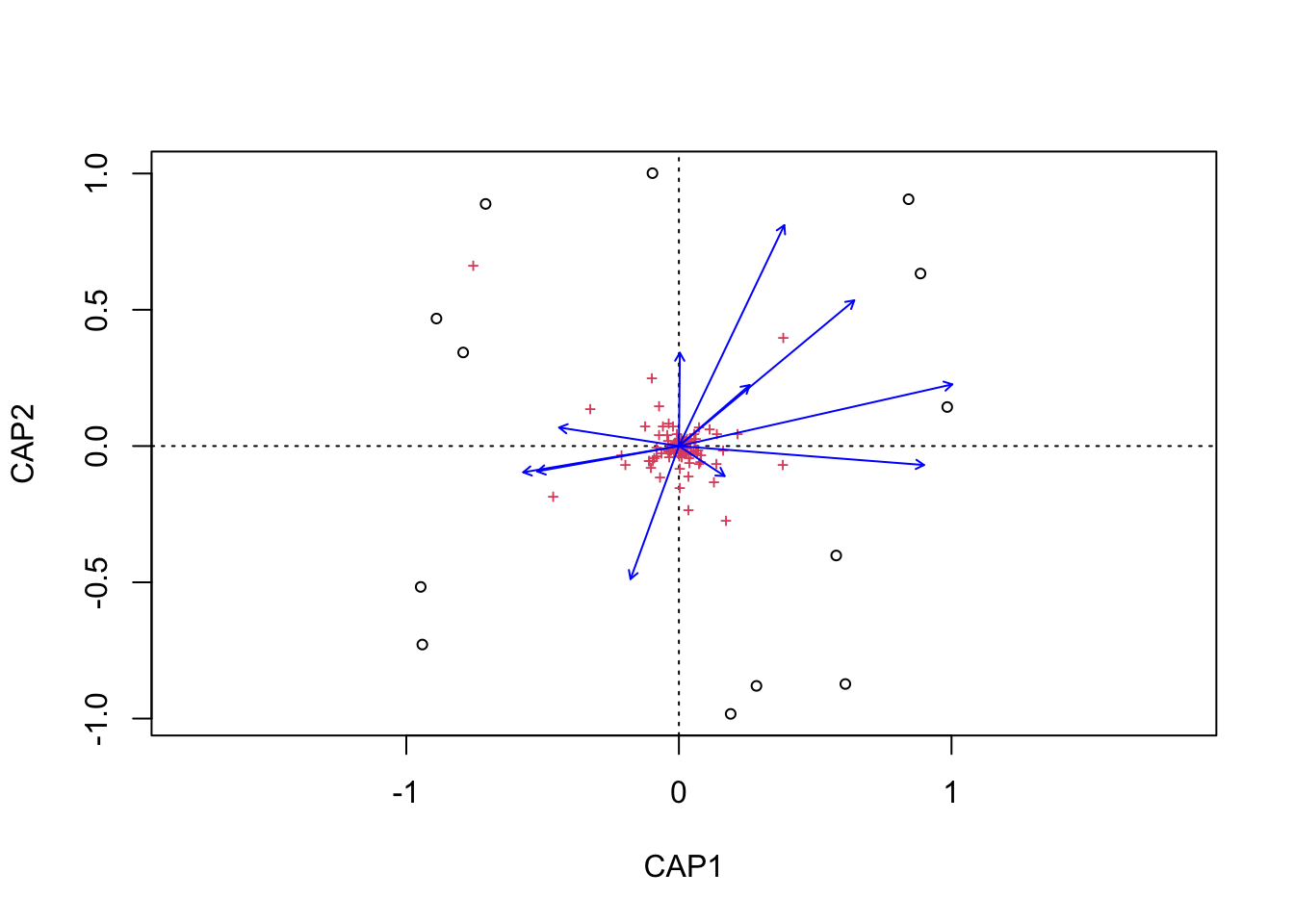``````tmp_auto_plt <- autoplot(temp_adapt_cap, arrows = TRUE)
tmp_auto_plt``````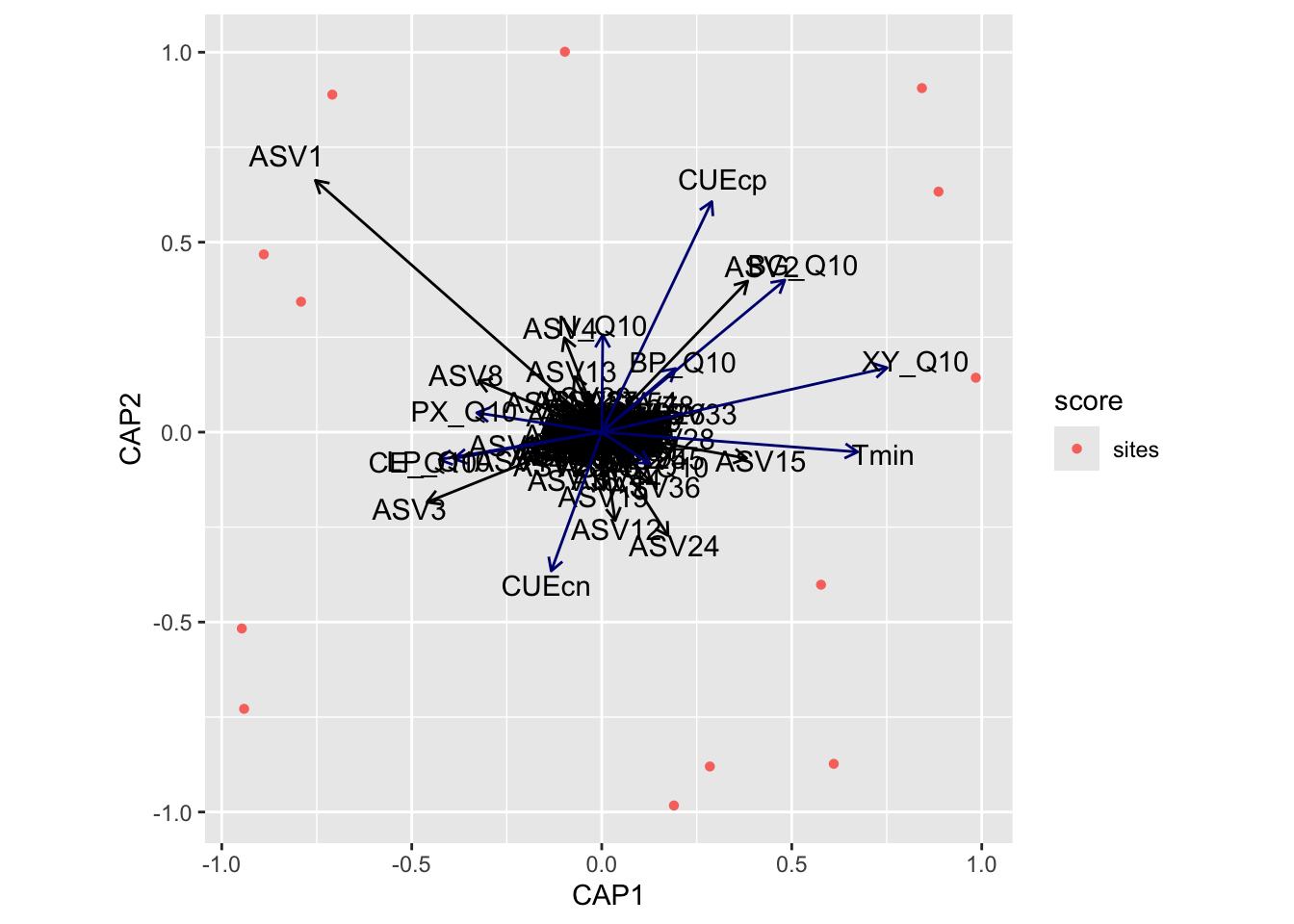Next, we need to grab capscale scores for the samples and create a data frame of the first two dimensions. We will also need to add some of the sample details to the data frame. For this we use the vegan function `scores` which gets species or site scores from the ordination.

Code
``````tmp_samp_scores <- dplyr::filter(tmp_auto_plt\$plot_env\$obj, Score == "sites")
tmp_samp_scores[,1] <- NULL
tmp_samp_scores <- tmp_samp_scores %>% dplyr::rename(SampleID = Label)

tmp_md_sub <- tmp_md[, 1:4]
tmp_md_sub <- tmp_md_sub %>% tibble::rownames_to_column("SampleID")
by = "SampleID")``````

Now we have a new data frame that contains sample details and capscale values.

``````          SampleID PLOT TREAT TREAT_T PAIR        CAP1       CAP2
1  P10_D00_010_C0E  P10     C       0    E -0.70909404  0.8884734
2  P02_D00_010_C0A  P02     C       0    A -0.88932428  0.4678685
3  P04_D00_010_C0B  P04     C       0    B -0.94720017 -0.5168746
4  P06_D00_010_C0C  P06     C       0    C -0.94117253 -0.7283971
5  P08_D00_010_C0D  P08     C       0    D -0.09697067  1.0013016
6  P01_D00_010_W3A  P01     W       3    A -0.79138504  0.3434287
7  P03_D00_010_W3B  P03     W       3    B  0.28447425 -0.8800769
8  P07_D00_010_W3D  P07     W       3    D  0.18992920 -0.9828048
9  P09_D00_010_W3E  P09     W       3    E  0.57704340 -0.4016378
10 P01_D00_010_W8A  P01     W       8    A  0.98434765  0.1432237
11 P03_D00_010_W8B  P03     W       8    B  0.88625427  0.6330378
12 P05_D00_010_W8C  P05     W       8    C  0.84253827  0.9056297
13 P07_D00_010_W8D  P07     W       8    D  0.61055970 -0.8731722``````

We can then do the same with the metadata vectors. Here though we only need the scores and parameter name.

Code
``````temp_adapt_md_scores <- dplyr::filter(tmp_auto_plt\$plot_env\$obj, Score == "biplot")
dplyr::mutate(parameters = Label, .before = CAP1) %>%
tibble::column_to_rownames("Label")``````
``````       parameters         CAP1        CAP2
AG_Q10     AG_Q10  0.138111424 -0.09067317
BG_Q10     BG_Q10  0.529871661  0.44030269
BP_Q10     BP_Q10  0.213674949  0.18420563
CE_Q10     CE_Q10 -0.470374849 -0.07935444
N_Q10       N_Q10  0.002856862  0.28186886
XY_Q10     XY_Q10  0.826073837  0.18643092
LP_Q10     LP_Q10 -0.428296135 -0.07664534
PX_Q10     PX_Q10 -0.361920412  0.05606159
CUEcn       CUEcn -0.146194994 -0.40205479
CUEcp       CUEcp  0.318510258  0.66752667
Tmin         Tmin  0.740618311 -0.05737920``````

Let’s run some quick correlations of metadata with ordination axes to see which parameters are significant. For this we use the vegan function `envfit`.

Code
``````tmp_samp_scores_sub <- temp_adapt_plot_data[, 6:7]
tmp_samp_scores_sub <- as.matrix(tmp_samp_scores_sub)

tmp_md_sub <- subset(tmp_md,  select =  tmp_param_list)

perm = 1000, choices = c(1, 2))``````
``````
***VECTORS

CAP1     CAP2     r2  Pr(>r)
AG_Q10  0.82904 -0.55918 0.0237 0.89710
BG_Q10  0.76182  0.64778 0.4109 0.07792 .
BP_Q10  0.74983  0.66163 0.0689 0.71528
CE_Q10 -0.98593 -0.16714 0.1956 0.34865
N_Q10   0.00657  0.99998 0.0700 0.71029
XY_Q10  0.97498  0.22227 0.6166 0.00999 **
LP_Q10 -0.98418 -0.17719 0.1627 0.44955
PX_Q10 -0.98713  0.15993 0.1155 0.54246
CUEcn  -0.33206 -0.94326 0.1605 0.40460
CUEcp   0.42027  0.90740 0.4786 0.03497 *
Tmin    0.99659 -0.08248 0.4749 0.02797 *
---
Signif. codes:  0 '***' 0.001 '**' 0.01 '*' 0.05 '.' 0.1 ' ' 1
Permutation: free
Number of permutations: 1000``````
Code
``````temp_adapt_md_signif_hits <- base::subset(envfit_temp_adapt_md\$vectors\$pvals,
< 0.05 & envfit_temp_adapt_md\$vectors\$r > 0.4))

`envfit` found that XY_Q10, CUEcp, Tmin were significantly correlated.

Now let’s see if the same parameters are significant for the `envfit` and `bioenv` analyses.

`````` "Significant parameters from bioenv analysis."
 "XY_Q10" "Tmin"
_____________________________________
 "Significant parameters from envfit analysis."
 "XY_Q10" "CUEcp"  "Tmin"
_____________________________________
 "Found in bioenv but not envfit."
character(0)
_____________________________________
 "Found in envfit but not bioenv."
 "CUEcp"
_____________________________________
 "Found in envfit and  bioenv."
 "XY_Q10" "CUEcp"  "Tmin"  ``````
Code
``````#new_temp_adapt_md_signif_hits <- base::append(temp_adapt_md_signif_hits, temp_adapt_sig_diff)

Check. Next, we run `envfit` for the ASVs.

Code
``````envfit_temp_adapt_asv <- envfit(tmp_samp_scores_sub,
tmp_comm[, order(colSums(-tmp_comm))][, 1:10],
perm = 1000, choices = c(1, 2))

temp_adapt_asv_scores <- dplyr::filter(tmp_auto_plt\$plot_env\$obj, Score == "species")

dplyr::mutate(parameters = Label, .before = CAP1) %>%
tibble::column_to_rownames("Label")
``````
***VECTORS

CAP1     CAP2     r2   Pr(>r)
ASV1  -0.68969  0.72410 0.2975 0.051948 .
ASV2   0.68393  0.72955 0.5592 0.003996 **
ASV4  -0.34226  0.93961 0.4935 0.005994 **
ASV3  -0.89727 -0.44148 0.5062 0.000999 ***
ASV15  0.96528 -0.26123 0.2497 0.122877
ASV8  -0.90985  0.41495 0.1146 0.923077
ASV9   0.76209 -0.64748 0.1338 0.474525
ASV24  0.44129 -0.89736 0.2375 0.095904 .
ASV12  0.14568 -0.98933 0.7737 0.002997 **
ASV13 -0.41856  0.90819 0.4357 0.056943 .
---
Signif. codes:  0 '***' 0.001 '**' 0.01 '*' 0.05 '.' 0.1 ' ' 1
Permutation: free
Number of permutations: 1000``````
Code
``````temp_adapt_asv_signif_hits <- base::subset(envfit_temp_adapt_asv\$vectors\$pvals,
< 0.05 & envfit_temp_adapt_asv\$vectors\$r > 0.5))
``````      parameters        CAP1       CAP2 variable_type
ASV12      ASV12  0.03533894 -0.2327656           ASV
ASV2        ASV2  0.38413654  0.3977765           ASV
ASV3        ASV3 -0.45977392 -0.1843674           ASV``````
Code
``````temp_adapt_md_signif_all\$variable_type <- "metadata"

The last thing to do is categorize parameters scores and ASV scores into different variable types for plotting.

Code
``````temp_adapt_bioplot_data_md <- subset(temp_adapt_bioplot_data,
Show the code for the plot
``````temp_adapt_cap_vals <- data.frame(temp_adapt_cap\$CCA\$eig[1:2])

cpa1_lab <- paste("CAP1", " (", temp_adapt_cap1, "%)", sep = "")
cpa2_lab <- paste("CAP2", " (", temp_adapt_cap2, "%)", sep = "")

swel_col <- c("#2271B2", "#71B222", "#B22271")

geom_point(mapping = aes(x = CAP1, y = CAP2, shape = TREAT,
colour = TREAT_T), size = 4) +
scale_colour_manual(values = swel_col) +
#  geom_text(data = temp_adapt_plot_data, aes(x = CAP1, y = CAP2, #UNCOMMENT to add sample labels
#                              label = SampleID), size = 3) +
geom_segment(aes(x = 0, y = 0, xend = CAP1, yend = CAP2),
data = temp_adapt_bioplot_data_md, linetype = "solid",
arrow = arrow(length = unit(0.3, "cm")), size = 0.4,
color = "#191919", inherit.aes = FALSE) +
aes(x = CAP1, y = CAP2, label = parameters), size = 3,
nudge_x = 0.1, nudge_y = 0.05) +
# uSe to include ASV vectors
#geom_segment(aes(x = 0, y = 0, xend = CAP1, yend = CAP2),
#             data = temp_adapt_bioplot_data_asv, linetype = "solid",
#             arrow = arrow(length = unit(0.3, "cm")), size = 0.2,
#             color = "#676767") +
#          aes(x = CAP1, y = CAP2, label = parameters), size = 2.5,
#          nudge_x = 0.05, nudge_y = 0.05) +
theme_classic(base_size = 12) +
labs(title = "Capscale Analysis",
x = cpa1_lab,
y = cpa2_lab)
height = 16, width = 20, units = 'cm', res = 600, bg = "white")
invisible(dev.off())
height = 5, width = 6)
dev.off()``````

## Capscale Plots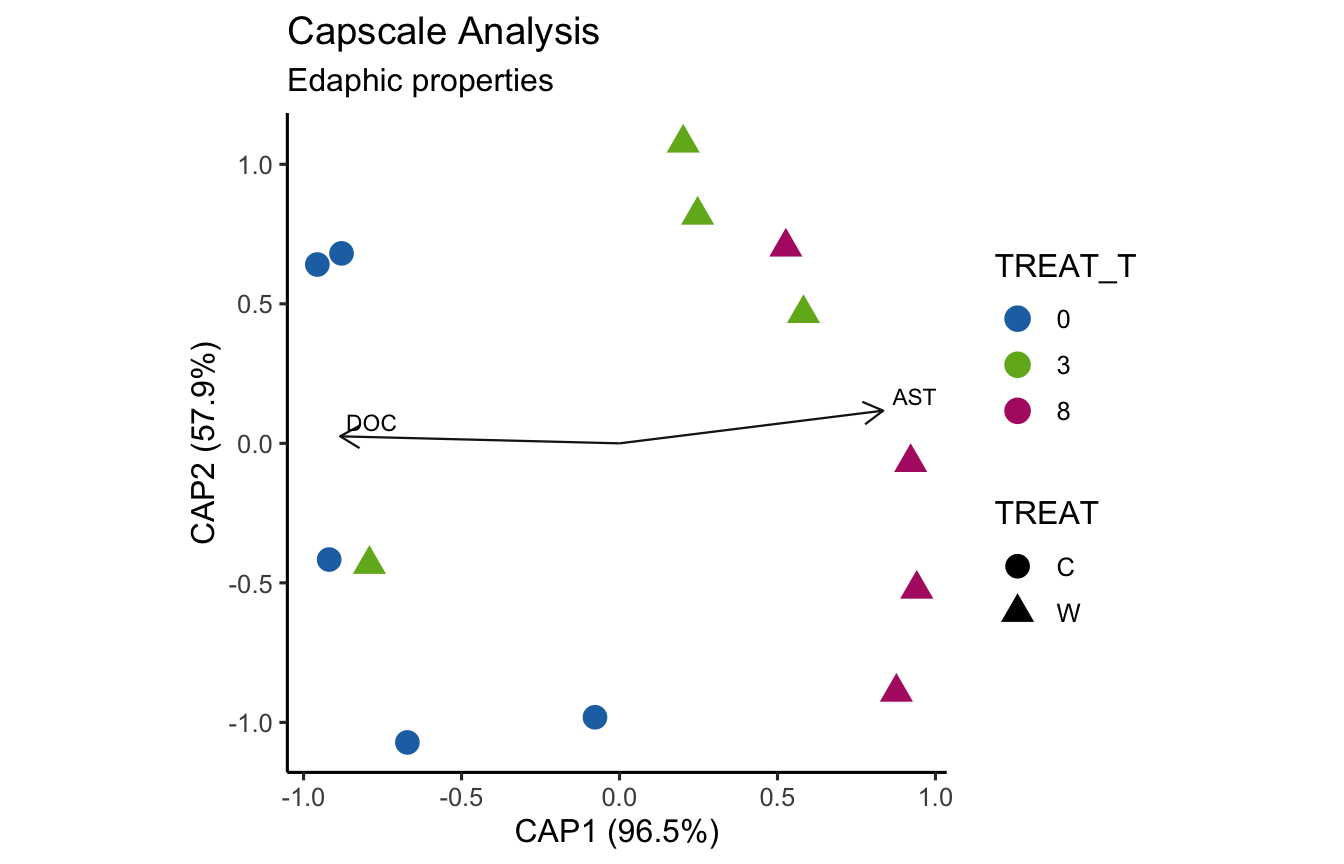(ITS) Figure 7 | Distance-based Redundancy Analysis (db-RDA) of PIME filtered data based on Bray-Curtis dissimilarity showing the relationships between community composition change versus edaphic properties.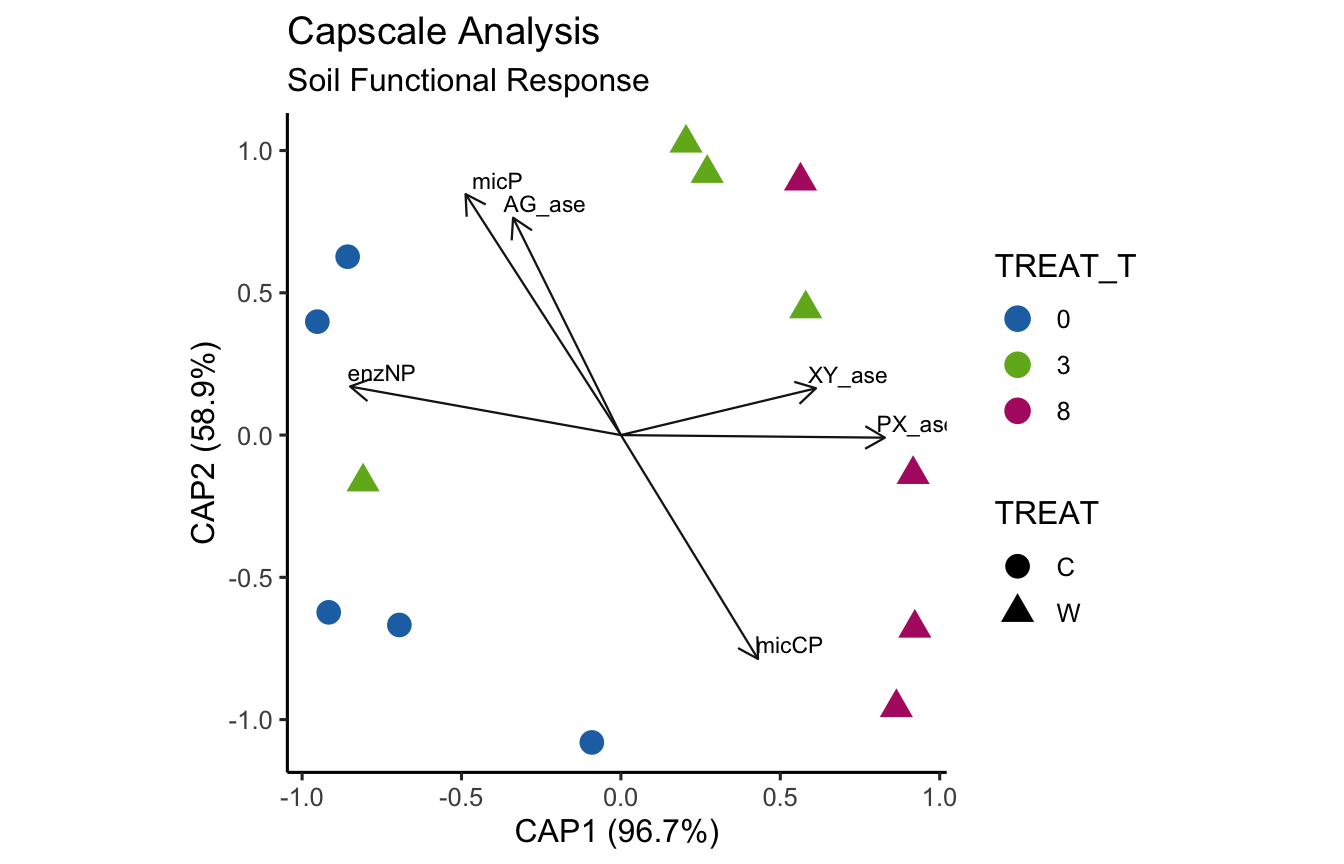(ITS) Figure 8 | Distance-based Redundancy Analysis (db-RDA) of PIME filtered data based on Bray-Curtis dissimilarity showing the relationships between community composition change versus microbial functional response.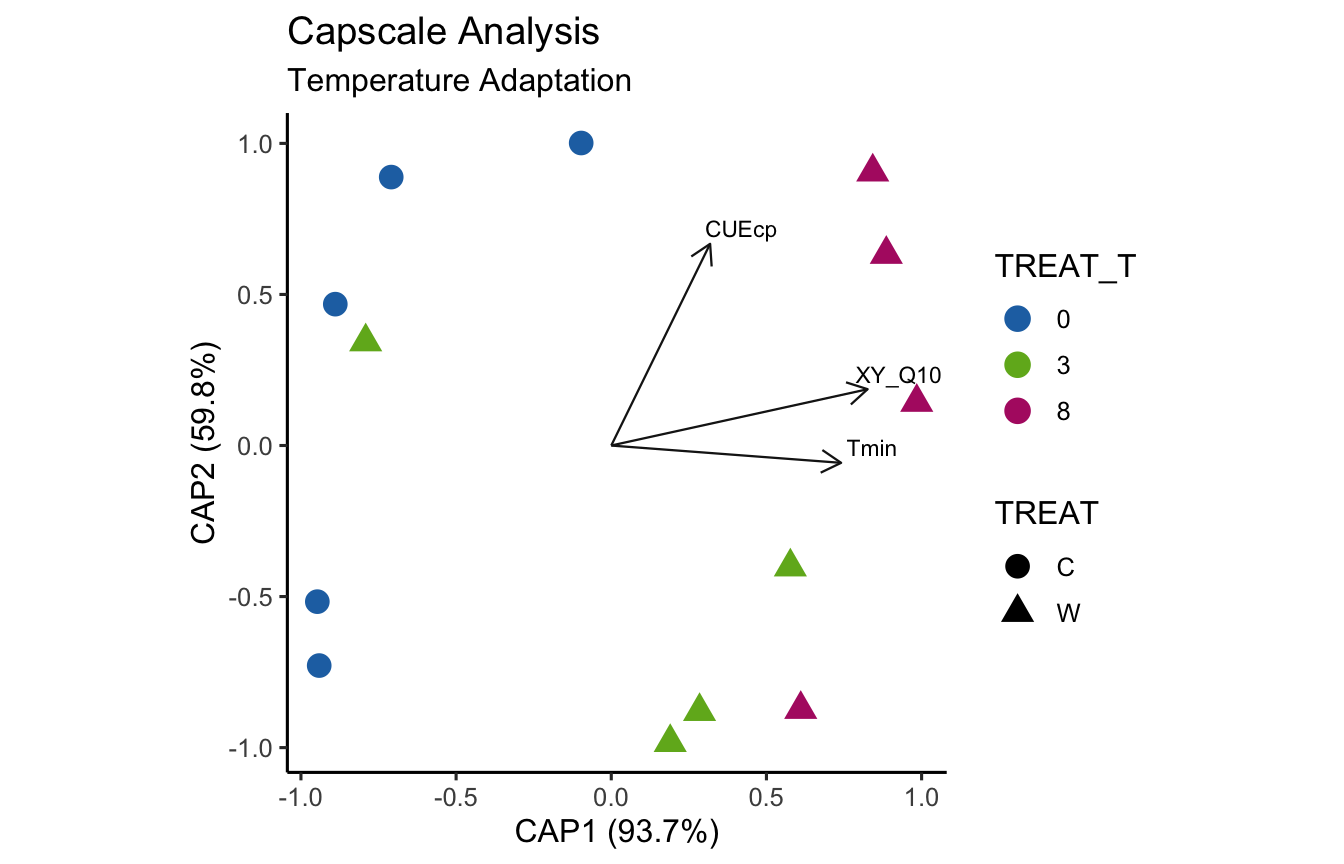(ITS) Figure 9 | Distance-based Redundancy Analysis (db-RDA) of PIME filtered data based on Bray-Curtis dissimilarity showing the relationships between community composition change versus temperature adaptation.

# Workflow Output

## Source Code

`` "2022-06-29 07:22:52 EST"``